# 8 RSA on Pattern Similarity Change

In order to assess changes in representational similarity due to the day learning task, which took place in between the two picture viewing tasks, we quantified pattern similarity changes as the difference of the respective correlation coefficients for every pair of events between the post-learning picture viewing task and its pre-learning baseline equivalent (Figure 3). Then, we analyzed how these difference values related to temporal relations between events, which we quantified using the absolute differences in virtual time (“virtual time”) between events (Figure 1C, bottom right). We further tested whether the effect of virtual time on anterior hippocampal pattern similarity change persisted when including the absolute difference between sequence positions (“order”) and the interval in seconds between events (“real time”) as control predictors of no interest in the model. We separately tested the effect of virtual time for event pairs from the same or different sequences and thus use a Bonferroni-corrected α-level of 0.025 for these tests. Time metrics were z-scored within each participant prior to analysis.

Next, we can run our analyses of representational change in the ROIs. Let’s load the data.

# load the data
col_types_list <- cols_only(
sub_id = col_character(),
day1 = col_integer(), day2 = col_integer(),
event1 = col_integer(), event2 = col_integer(),
pic1 = col_integer(), pic2 = col_integer(),
virtual_time1 = col_double(), virtual_time2 = col_double(),
real_time1 = col_double(), real_time2 = col_double(),
memory_time1 = col_double(), memory_time2 = col_double(),
memory_order1 = col_double(), memory_order2 = col_double(),
sorted_day1 = col_integer(), sorted_day2 = col_integer(),
pre_corrs  = col_double(), post_corrs = col_double(),
ps_change = col_double(), roi = col_factor())

fov_fn <- file.path(dirs$data4analysis, "mni1mm_masks", "fov_mask_mni.nii.gz") fov_nii <- readNIfTI2(fov_fn) fov_nii[fov_nii >0] <- 1 fov_nii[fov_nii !=1 ] <- 0 # make a mask for the brain area outside our FOV out_fov <- (fov_nii == 0) & (mni_nii>0) mni_nii[out_fov] <- scales::rescale(mni_nii[out_fov], from=range(mni_nii[out_fov]), to=c(6000, 8207)) mni_nii[mni_nii == 0] <- NA ### Anterior hippocampus ROI # load the ROI roi_fn <- file.path(dirs$data4analysis, "mni1mm_masks", "aHPC_lr_group_prob_mni1mm.nii.gz")
roi_nii[roi_nii == 0] <- NA

# choose coordinates (these are chosen manually now)
coords <- mni2vox(c(-24,-15,-20))

# f4a
# get ggplot object for template as background
ggTemplate<-getggTemplate(col_template=rev(RColorBrewer::brewer.pal(8, 'Greys')),brains=mni_nii,
all_brain_and_time_inds_one=TRUE,
mar=c(1,2,3), mar_ind=coords, col_ind = c(1,1,1),
row_ind=sprintf("%s=%d",c("x","y","z"),vox2mni(coords)), center_coords=TRUE)

# get data frame for the ROI image
roi_df <- getBrainFrame(brains=roi_nii, mar=c(1,2,3),
row_ind = sprintf("%s=%d",c("x","y","z"),vox2mni(coords)),
#row_ind = c(1,2,3), col_ind = c(1,1,1),

label_df <- data.frame(row_ind = sprintf("%s=%d",c("x","y","z"),vox2mni(coords)),
label = sprintf("%s=%d",c("x","y","z"),vox2mni(coords)))

f4a <- ggTemplate +
geom_tile(data=roi_df, aes(x=row,y=col,fill=value))+
facet_wrap(~row_ind, nrow=3, ncol=1, strip.position = "top", scales = "free") +
scico::scale_fill_scico(palette = "roma",
begin = 0, end=1,
name = "probability",
limits = round(range(roi_df$value), digits=2), breaks = round(range(roi_df$value), digits=2)) +
theme_cowplot(line_size = NA) +
theme(strip.background = element_blank(), strip.text.x = element_blank(),
text = element_text(size=10), axis.text = element_text(size=8),
legend.key.size = unit(0.015, "npc"),
legend.position = "bottom", legend.justification = c(0.5, 0.5),
aspect.ratio = 1)+
guides(fill = guide_colorbar(
direction = "horizontal",
title.position = "top",
title.hjust = 0.5,
label.position = "bottom"
))+
xlab(element_blank()) + ylab(element_blank()) +
coord_cartesian(clip = "off") +
geom_text(data = label_df, x=c(0,0,0), y=c(-84.7,-67.25,-84.45), aes(label = label),
size = 8/.pt, family=font2use, vjust=1) +
# y coords found via command below (to keep text aligned across panels):
# ggplot_build(f6g)$layout$panel_scales_y[]$range$range-diff(ggplot_build(f6g)$layout$panel_scales_y[]$range$range)/100*3
theme_no_ticks()
print(f4a)### Anterior-lateral entorhinal cortex

# load the ROI
roi_fn <- file.path(dirs$data4analysis, "mni1mm_masks", "alEC_lr_group_prob_mni1mm.nii.gz") roi_nii <- readNIfTI2(roi_fn) roi_nii[roi_nii == 0] <- NA # choose coordinates (these are chosen manually now) coords <- mni2vox(c(18,1,-35)) # f6a # get ggplot object for template as background ggTemplate<-getggTemplate(col_template=rev(RColorBrewer::brewer.pal(8, 'Greys')),brains=mni_nii, all_brain_and_time_inds_one=TRUE, mar=c(1,2,3), mar_ind=coords, col_ind = c(1,1,1), row_ind=sprintf("%s=%d",c("x","y","z"),vox2mni(coords)), center_coords=TRUE) #row_ind=c(1,2,3), center_coords=TRUE) # get data frame for the ROI image roi_df <- getBrainFrame(brains=roi_nii, mar=c(1,2,3), row_ind = sprintf("%s=%d",c("x","y","z"),vox2mni(coords)), #row_ind = c(1,2,3), col_ind = c(1,1,1), mar_ind=coords, mask=NULL, center_coords=TRUE) label_df <- data.frame(row_ind = sprintf("%s=%d",c("x","y","z"),vox2mni(coords)), label = sprintf("%s=%d",c("x","y","z"),vox2mni(coords))) f6a <- ggTemplate + geom_tile(data=roi_df, aes(x=row,y=col,fill=value))+ facet_wrap(~row_ind, nrow=3, ncol=1, strip.position = "top", scales = "free") + scico::scale_fill_scico(palette = "roma", begin = 0, end=1, name = "probability", limits = round(range(roi_df$value), digits=2),
breaks = round(range(roi_dfvalue), digits=2)) + theme_cowplot(line_size = NA) + theme(strip.background = element_blank(), strip.text.x = element_blank(), text = element_text(size=10), axis.text = element_text(size=8), legend.key.size = unit(0.015, "npc"), legend.position = "bottom", legend.justification = c(0.5, 0.5), aspect.ratio = 1 )+ guides(fill = guide_colorbar( direction = "horizontal", title.position = "top", title.hjust = 0.5, label.position = "bottom"))+ xlab(element_blank()) + ylab(element_blank()) + coord_cartesian(clip = "off") + geom_text(data = label_df, x=c(0,0,0), y=c(-85.82,-72.31,-78.1), aes(label = label), size = 8/.pt, family=font2use, vjust=1) + # y coords found via command below (to keep text aligned across panels): # ggplot_build(f6g)layout$panel_scales_y[]$range$range-diff(ggplot_build(f6g)$layout$panel_scales_y[]$range$range)/100*3 theme_no_ticks() print(f6a)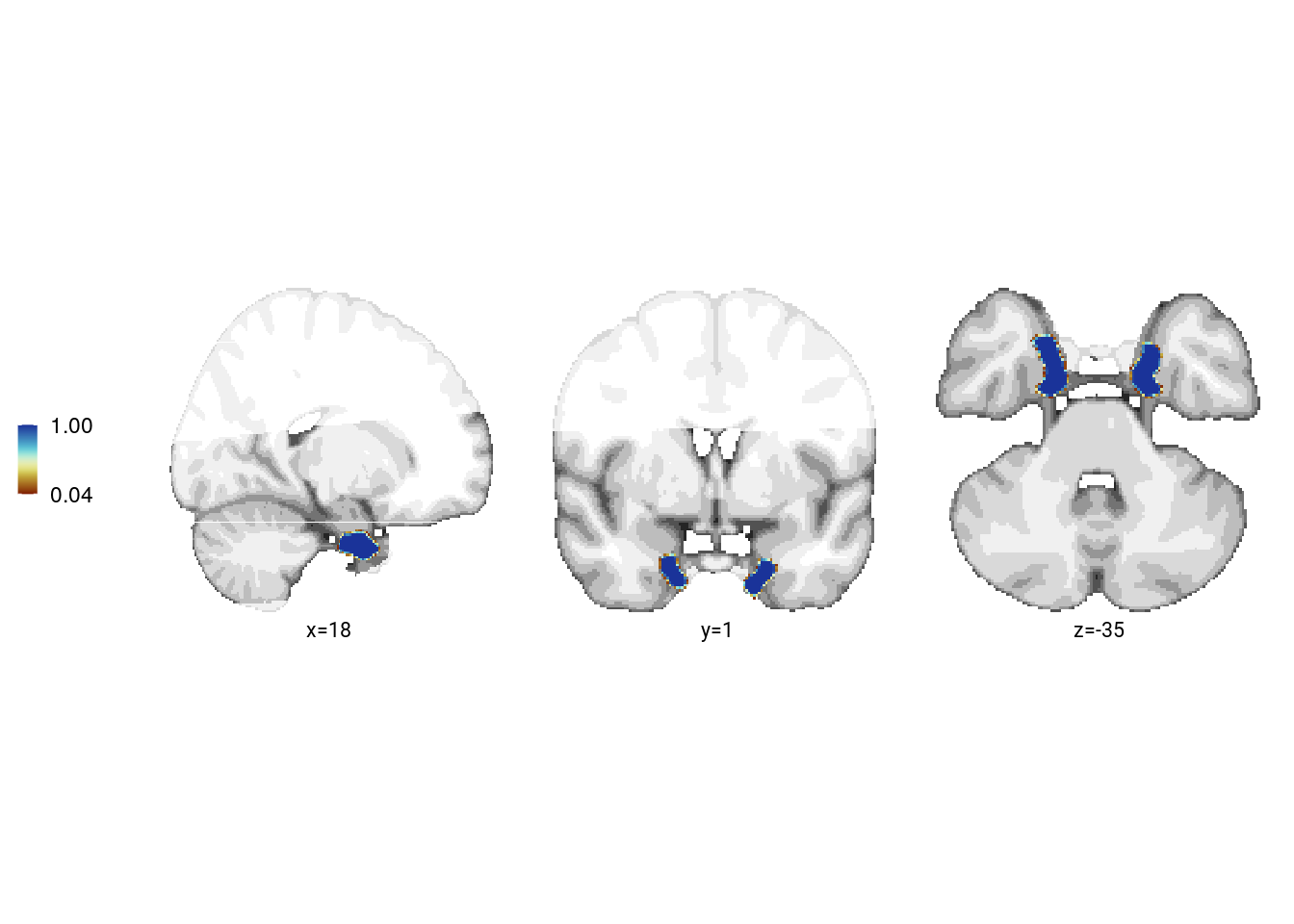## 8.3 First-level RSA In the summary statistics approach, we used the different time metrics as predictors for pattern similarity change. We set up a GLM with the given variable from the day learning task as a predictor and the pairwise representational change values as the criterion for every participant. The t-values of the resulting model coefficients were then compared to a null distribution obtained from shuffling the dependent variable of the linear model (i.e. pattern similarity change) 10000 times. This approach to permutation-testing of regression coefficients controls Type I errors even under situations of collinear regressors (Anderson and Legendre, 1999). Resulting p-values for each coefficient were transformed to a Z-score. The Z-scores were then used for group-level inferential statistics. Group-level statistics were carried out using permutation-based procedures. For t-tests, we compared the observed t-values against a surrogate distribution obtained from 10,000 random sign-flips to non-parametrically test against 0 or to assess within-participant differences between conditions (two-sided tests; α=0.05 unless stated otherwise). We report Cohen’s d with Hedges’ correction and its 95% confidence interval as computed using the effsize-package for R. For paired tests, Cohen’s d was calculated using pooled standard deviations and confidence intervals are based on the non-central t-distribution. Permutation-based repeated measures ANOVAs were carried out using the permuco-package111 and we report generalized η2 as effect sizes computed using the afex-package112. For the summary statistics approach, let’s run the first-level analysis using virtual time as a predictor of pattern similarity change separately for each participant and each ROI. For simplicity we run these analysis together here and will run separate group-level stats below. set.seed(100) # set seed for reproducibility # select the data from alEC and HPC rsa_dat_mtl <- rsa_dat %>% filter(roi == "alEC_lr" | roi == "aHPC_lr") # do RSA using linear model and calculate z-score for model fit based on permutations rsa_fit <- rsa_dat_mtl %>% group_by(sub_id, roi, same_day) %>% # run the linear model do(z = lm_perm_jb(in_dat = ., lm_formula = "ps_change ~ vir_time_diff", nsim = n_perm)) %>% mutate(z = list(setNames(z, c("z_intercept", "z_virtual_time")))) %>% unnest_wider(z) # add group column used for plotting rsa_fit$group <- factor(1)

# add a factor with character labels for within/across days and one to later color control in facets
rsa_fit <- rsa_fit %>%
mutate(same_day_char = plyr::mapvalues(same_day,
from = c(0, 1),
to = c("across days", "within days"),
warn_missing = FALSE),
same_day_char = factor(same_day_char, levels = c("within days", "across days"))) %>%
unite(roi_same_day, c("roi", "same_day_char"), remove = FALSE, sep = " ") %>%
mutate(roi_same_day = str_replace(roi_same_day, "_lr", ":"),
roi_same_day = factor(roi_same_day, levels = c("aHPC: within days", "aHPC: across days",
"alEC: within days", "alEC: across days"))
)

## 8.4 aHPC: virtual time within sequence

We first test, whether virtual time explains pattern similarity change within the aHPC for event pairs from the same sequence.

### Summary statistics

set.seed(101) # set seed for reproducibility

# run a group-level t-test on the RSA fits from the first level in aHPC for within-day
stats <- rsa_fit %>%
filter(roi == "aHPC_lr", same_day == TRUE) %>%
select(z_virtual_time) %>%
paired_t_perm_jb (., n_perm = n_perm)

# Cohen's d with Hedges' correction for one sample using non-central t-distribution for CI
d<-cohen.d(d=(rsa_fit %>% filter(roi == "aHPC_lr", same_day == TRUE))$z_virtual_time, f=NA, paired=TRUE, hedges.correction=TRUE, noncentral=TRUE) stats$d <- d$estimate stats$dCI_low <- d$conf.int[] stats$dCI_high <- d$conf.int[] # print results huxtable(stats) %>% theme_article() Table 8.2: estimatestatisticp.valuep_permparameterconf.lowconf.highmethodalternativeddCI_lowdCI_high 0.6183.070.00480.006270.2051.03One Sample t-testtwo.sided0.5640.1780.995 Summary Statistics: t-test against 0 for virtual time within sequence in aHPC t27=3.07, p=0.006, d=0.56, 95% CI [0.18, 1.00] # select the data to plot plot_dat <- rsa_fit %>% filter(roi == "aHPC_lr", same_day == TRUE) # raincloud plot f4b <- ggplot(plot_dat, aes(x=1, y=z_virtual_time, fill = roi, color = roi)) + gghalves::geom_half_violin(position=position_nudge(0.1), side = "r", color = NA) + geom_point(aes(x = 0.8, y = z_virtual_time), alpha = 0.7, position = position_jitter(width = .1, height = 0), shape=16, size = 1) + geom_boxplot(position = position_nudge(x = 0, y = 0), width = .1, colour = "black", outlier.shape = NA) + stat_summary(fun = mean, geom = "point", size = 1, shape = 16, position = position_nudge(.1), colour = "black") + stat_summary(fun.data = mean_se, geom = "errorbar", position = position_nudge(.1), colour = "black", width = 0, size = 0.5)+ scale_color_manual(values=unname(aHPC_colors["within_main"])) + scale_fill_manual(values=unname(aHPC_colors["within_main"])) + ylab('z RSA model fit') + xlab("virtual time") + guides(fill = FALSE, color = FALSE) + annotate(geom = "text", x = 1, y = 2.6, label = "**", hjust = 0.5, size = 8/.pt, family=font2use) + theme_cowplot() + theme(text = element_text(size=10), axis.text = element_text(size=8), legend.position = "none", axis.ticks.x = element_blank(), axis.text.x = element_blank()) f4b### Linear Mixed Effects Likewise, we want to test whether similarity change reflects virtual time differences between elements within a sequence using a mized model. Thus, we use virtual time differences between scenes as the only fixed effect. Following the recommendation for maximal random effect structures by Barr et al. (JML, 2013), we first include random intercepts and random slopes for each subject. This results in a singular fit (random intercept variance estimated as 0). To avoid anti-conservativity we thus drop the correlation between random intercepts and random slopes as suggested by Barr et al., who demonstrate that “LMEMs with maximal random slopes, but missing either random correlations or within-unit random intercepts, performed nearly as well as “fully” maximal LMEMs, with the exception of the case where p-values were determined by MCMC sampling" (p. 267). This model converges without warnings. Below are the model summaries. set.seed(56) # set seed for reproducibility # extract the comparisons from the same day rsa_dat_same_day <- rsa_dat %>% filter(roi == "aHPC_lr", same_day == TRUE) # define the full model with virtual time difference as # fixed effect and random intercepts for subject # maximal random effects structure with random slopes and intercepts --> singular fit formula <- "ps_change ~ vir_time_diff + (1 + vir_time_diff | sub_id)" lmm_full <- lme4::lmer(formula, data = rsa_dat_same_day, REML = FALSE, control=lmerControl(optCtrl=list(maxfun=20000))) ## boundary (singular) fit: see ?isSingular # thus we reduce the random effects structure. Following Barr et al. (2013), we drop the correlation between random intercepts and random slopes set.seed(345) # set seed for reproducibility formula <- "ps_change ~ vir_time_diff + (1 + vir_time_diff || sub_id)" lmm_full <- lme4::lmer(formula, data = rsa_dat_same_day, REML = FALSE, control=lmerControl(optCtrl=list(maxfun=20000))) summary(lmm_full, corr = FALSE) ## Linear mixed model fit by maximum likelihood ['lmerMod'] ## Formula: ps_change ~ vir_time_diff + ((1 | sub_id) + (0 + vir_time_diff | sub_id)) ## Data: rsa_dat_same_day ## Control: lmerControl(optCtrl = list(maxfun = 20000)) ## ## AIC BIC logLik deviance df.resid ## -7951.4 -7926.3 3980.7 -7961.4 1115 ## ## Scaled residuals: ## Min 1Q Median 3Q Max ## -3.4983 -0.6232 -0.0284 0.6645 3.8269 ## ## Random effects: ## Groups Name Variance Std.Dev. ## sub_id (Intercept) 6.434e-13 8.021e-07 ## sub_id.1 vir_time_diff 6.598e-08 2.569e-04 ## Residual 4.784e-05 6.917e-03 ## Number of obs: 1120, groups: sub_id, 28 ## ## Fixed effects: ## Estimate Std. Error t value ## (Intercept) -0.0003261 0.0002112 -1.544 ## vir_time_diff 0.0007513 0.0002197 3.420 # tidy summary of the fixed effects that calculates 95% CIs lmm_full_bm <- broom.mixed::tidy(lmm_full, effects = "fixed", conf.int=TRUE, conf.method="profile") ## Computing profile confidence intervals ... # tidy summary of the random effects lmm_full_bm_re <- broom.mixed::tidy(lmm_full, effects = "ran_pars") We assess the statistical significance of the fixed effect of virtual time using a likelihood ratio test against a reduced (nested) model that does not have this fixed effect. # one way of testing for significance is by comparing the likelihood against a simpler model. # Here, we drop the effect of virtual time difference and run an ANOVA. See e.g. Bodo Winter tutorial set.seed(348) # set seed for reproducibility formula <- "ps_change ~ 1 + (1 + vir_time_diff || sub_id)" lmm_no_vir_time <- lme4::lmer(formula, data = rsa_dat_same_day, REML = FALSE) ## boundary (singular) fit: see ?isSingular lmm_aov <- anova(lmm_no_vir_time, lmm_full) lmm_aov Table 8.3: nparAICBIClogLikdevianceChisqDfPr(>Chisq) 4-7.94e+03-7.92e+033.98e+03-7.95e+03 5-7.95e+03-7.93e+033.98e+03-7.96e+039.8710.00168 Mixed Model: Fixed effect of virtual time on aHPC pattern similarity change $$\chi^2$$(1)=9.87, p=0.002 Make mixed model summary table that includes overview of fixed and random effects as well as the model comparison to the nested (reduced) model. fe_names <- c("intercept", "virtual time") re_groups <- c(rep("participant",2), "residual") re_names <- c("intercept (SD)", "virtual time", "SD") lmm_hux <- make_lme_huxtable(fix_df=lmm_full_bm, ran_df = lmm_full_bm_re, aov_mdl = lmm_aov, fe_terms =fe_names, re_terms = re_names, re_groups = re_groups, lme_form = gsub(" ", "", paste0(deparse(formula(lmm_full)), collapse = "", sep="")), caption = "Mixed Model: Virtual time explains representational change for same-sequence events in the anterior hippocampus") # convert the huxtable to a flextable for word export stable_lme_aHPC_virtime_same_seq <- convert_huxtable_to_flextable(ht = lmm_hux) # print to screen theme_article(lmm_hux) fixed effects term estimate SE t-value -0.000326 0.000211 -1.54 -0.000740 0.000088 0.000751 0.000220 3.42 0.000307 0.001196 0.000001 0.000257 0.006917 -7943.56 3975.78 -7951.43 3980.72 9.87 1 0.002 To visualize this effect, we plot the estimate for the fixed effect coefficient of virtual time together with its confidence intervals. Further we plot the estimated marginal means for illustration. lmm_full_bm <- lmm_full_bm %>% mutate(term=as.factor(term)) # dot plot of Fixed Effect Coefficients with CIs f4c <- ggplot(data = lmm_full_bm[2,], aes(x = term, color = term)) + geom_hline(yintercept = 0, colour="black", linetype="dotted") + geom_errorbar(aes(ymin = conf.low, ymax = conf.high, width = NA), size = 0.5) + geom_point(aes(y = estimate), shape = 16, size = 1) + scale_fill_manual(values = unname(aHPC_colors["within_main"])) + scale_color_manual(values = unname(aHPC_colors["within_main"]), labels = c("Virtual Time")) + scale_y_continuous(breaks = seq(from=0, to=0.00125, by=0.00025), labels = c("0.000", "", "", "", 0.001, "")) + labs(x = "virtual time", y="fixed effect\ncoefficient", color = "Time Metric") + guides(color = FALSE) + annotate(geom = "text", x = 1, y = 0.00125, label = "**", hjust = 0.5, size = 8/.pt, family=font2use) + theme_cowplot() + theme(plot.title = element_text(hjust = 0.5), axis.text.x=element_blank()) f4c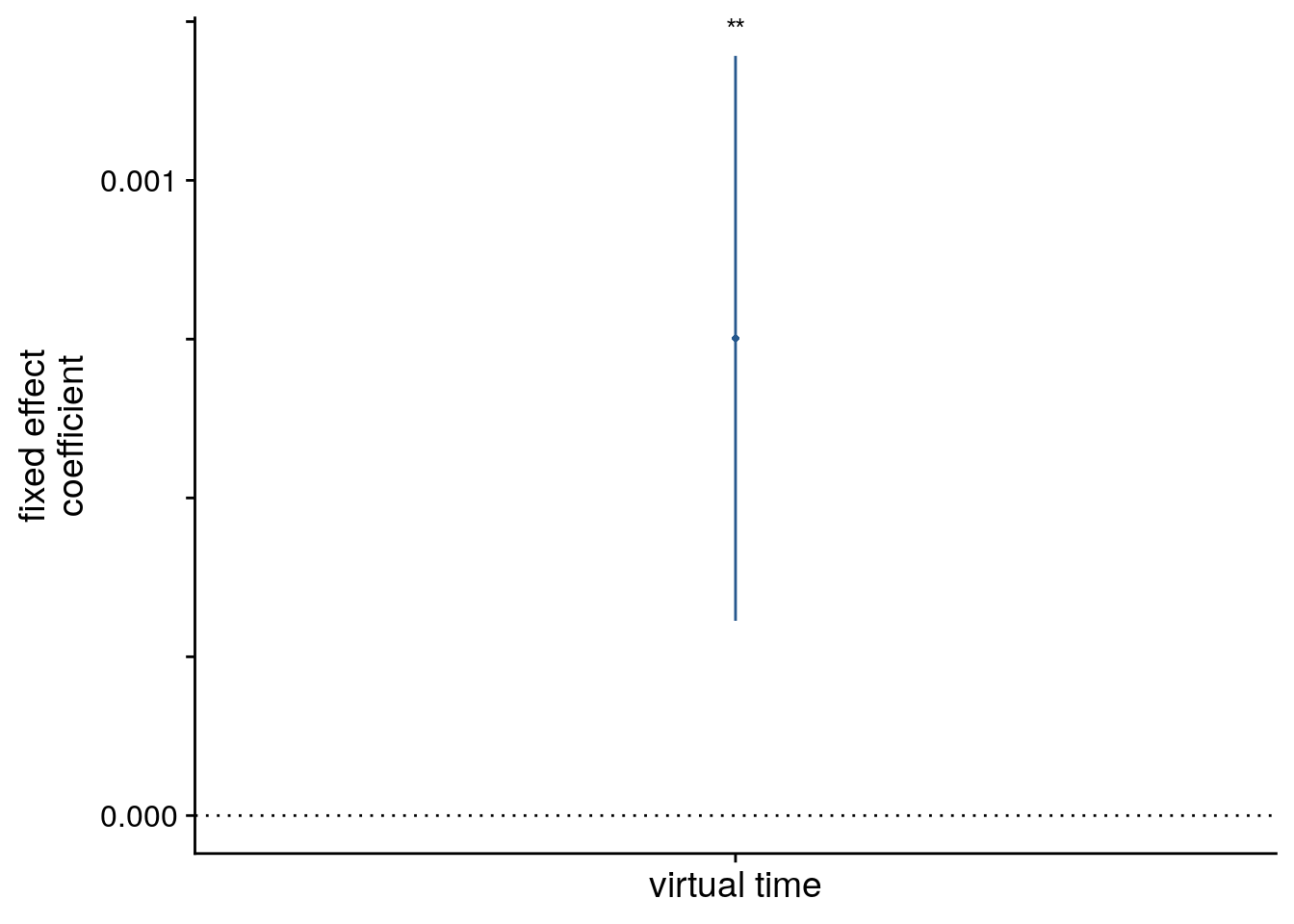# estimate marginal means for each model term by omitting the terms argument lmm_full_emm <- ggeffects::ggpredict(lmm_full, ci.lvl = 0.95) %>% get_complete_df() # plot marginal means f4d <- ggplot(data = lmm_full_emm, aes(color = group)) + geom_line(aes(x, predicted)) + geom_ribbon(aes(x, ymin = conf.low, ymax = conf.high, fill = group), alpha = .2, linetype=0) + scale_color_manual(values = unname(aHPC_colors["within_main"])) + scale_fill_manual(values = unname(aHPC_colors["within_main"])) + ylab('estimated\nmarginal means') + xlab('z virtual time') + guides(color = FALSE, fill =FALSE) + theme_cowplot() f4d#### LME model assumptions lmm_diagplots_jb(lmm_full)### Not driven by first & last event pairs A possible explanation for the positive correlation of pattern similarity and temporal distance could be that, in contrast to our previous work (Deuker et al., 2016), participants had to learn multiple sequences. To do so, participants might group the events by strongly associating the first and last event of a sequence. Now, let’s see if the within-day hippocampus effect goes beyond the effect of the most extreme comparisons, i.e. comparisons between the first and last day of an event. These could for example be used by participants to chunk the events into virtual days. For that we create a binary variable (deviation-coded) that tells us whether a comparison is between the first and last event of a virtual day. #### Summary Statistics In the summary statistics approach, let’s test a multiple regression model that includes the virtual time predictor and a binary predictor for those first/last event pairs. The code below implements the first- and second-level analysis. set.seed(57) # set seed for reproducibility # extract all comparisons from the same day rsa_dat_same_day_aHPC <- rsa_dat %>% filter(roi == "aHPC_lr", same_day == TRUE) # find comparisons of first and last event, which are the comparisons of events 1 and 5 # that have an order difference of 4 rsa_dat_same_day_aHPC$first_last <- abs(rsa_dat_same_day_aHPC$event1 - rsa_dat_same_day_aHPC$event2) == 4

# do RSA using linear model and calculate z-score for model fit from permutations
rsa_fit_aHPC_first_last <- rsa_dat_same_day_aHPC %>% group_by(sub_id) %>%
# run the linear model
do(z = lm_perm_jb(in_dat = .,
lm_formula = "ps_change ~ vir_time_diff + first_last",
nsim = n_perm)) %>%
mutate(z = list(setNames(z, c("z_intercept",  "z_virtual_time", "z_first_last")))) %>%
unnest_longer(z) %>%
filter(z_id != "z_intercept")

# run group-level t-tests on the RSA fits from the first level in aHPC for within-day
stats <- rsa_fit_aHPC_first_last %>%
filter(z_id == "z_virtual_time") %>%
select(z) %>%
paired_t_perm_jb (., n_perm = n_perm)

# Cohen's d with Hedges' correction for one sample using non-central t-distribution for CI
d<-cohen.d(d=(rsa_fit_aHPC_first_last %>% filter(z_id=="z_virtual_time"))$z, f=NA, paired=TRUE, hedges.correction=TRUE, noncentral=TRUE) stats$d <- d$estimate stats$dCI_low <- d$conf.int[] stats$dCI_high <- d$conf.int[] # print results huxtable(stats) %>% theme_article() Table 8.5: estimatestatisticp.valuep_permparameterconf.lowconf.highmethodalternativeddCI_lowdCI_high 0.4242.250.03270.0341270.03750.811One Sample t-testtwo.sided0.4130.03520.824 Summary Statistics: t-test against 0 for virtual time within sequence in aHPC with first-last-event-pair control predictor in the model t27=2.25, p=0.034, d=0.41, 95% CI [0.04, 0.82] # select the data to plot plot_dat <- rsa_fit_aHPC_first_last %>% mutate(z_id = factor(z_id, levels = c("z_virtual_time", "z_first_last"))) # raincloud plot sfiga <- ggplot(plot_dat, aes(x=z_id, y=z, fill = z_id, color = z_id)) + gghalves::geom_half_violin(position=position_nudge(0.1), side = "r", color = NA) + geom_point(aes(x = as.numeric(z_id)-0.2, y = z), position = position_jitter(width = .1, height = 0), shape=16, size = 1, alpha = 0.7) + geom_boxplot(position = position_nudge(x = 0, y = 0), width = .1, colour = "black", outlier.shape = NA) + stat_summary(fun = mean, geom = "point", size = 1, shape = 16, position = position_nudge(.1), colour = "black") + stat_summary(fun.data = mean_se, geom = "errorbar", position = position_nudge(.1), colour = "black", width = 0, size = 0.5)+ scale_color_manual(values=unname(c(aHPC_colors["within_main"], ultimate_gray)), name = "RSA predictor", labels = c("virtual time", "first & last event pair")) + scale_fill_manual(values=unname(c(aHPC_colors["within_main"], ultimate_gray))) + scale_x_discrete(labels=c("virtual time", "first & last")) + ylab('z RSA model fit') + xlab('predictor') + guides(fill=FALSE, color=guide_legend(override.aes=list(fill=NA, alpha = 1, size=2))) + annotate(geom = "text", x = 1, y = 2.2, label = "*", hjust = 0.5, size = 8/.pt, family=font2use) + theme_cowplot() + theme(text = element_text(size=10), axis.text = element_text(size=8), legend.direction = "horizontal", legend.position = "bottom", legend.justification = "center") sfiga#### Linear Mixed Effects Now, let’s do the same using a mixed model. We add the first-last variable as a fixed effect to the model in addition to virtual time to ensure that the effect of virtual time is not exclusively driven by comparisons of the first and last events. The model converges without warnings only with a random effects structure reduced to random slopes for virtual time. set.seed(58) # set seed for reproducibility # extract the comparisons from the same day and add variable indicating whether comparison # is between first and last event of a day rsa_dat_same_day <- rsa_dat %>% filter(roi == "aHPC_lr", same_day == TRUE) %>% mutate(first_last = (as.numeric(abs(event1 - event2) == 4)-0.5)*2) # define the full model with virtual time difference and the extreme pair predictor as # fixed effects and random intercepts for subject # maximal random effects structure with random slopes and intercepts --> singular fit formula <- "ps_change ~ vir_time_diff + first_last + (1 + vir_time_diff + first_last | sub_id)" lmm_full <- lme4::lmer(formula, data = rsa_dat_same_day, REML = FALSE, control=lmerControl(optCtrl=list(maxfun=20000))) ## boundary (singular) fit: see ?isSingular # thus we remove random intercepts for first-last control predictor -> still singular set.seed(349) # set seed for reproducibility formula <- "ps_change ~ vir_time_diff + first_last + (1 + vir_time_diff | sub_id)" lmm_full <- lme4::lmer(formula, data = rsa_dat_same_day, REML = FALSE, control=lmerControl(optCtrl=list(maxfun=90000))) ## boundary (singular) fit: see ?isSingular # thus we also remove the correlation between random slopes for virtual time and random intercepts set.seed(349) # set seed for reproducibility formula <- "ps_change ~ vir_time_diff + first_last + (1 + vir_time_diff || sub_id)" lmm_full <- lme4::lmer(formula, data = rsa_dat_same_day, REML = FALSE, control=lmerControl(optCtrl=list(maxfun=90000))) summary(lmm_full, corr = FALSE) ## Linear mixed model fit by maximum likelihood ['lmerMod'] ## Formula: ps_change ~ vir_time_diff + first_last + ((1 | sub_id) + (0 + vir_time_diff | sub_id)) ## Data: rsa_dat_same_day ## Control: lmerControl(optCtrl = list(maxfun = 90000)) ## ## AIC BIC logLik deviance df.resid ## -7950.2 -7920.0 3981.1 -7962.2 1114 ## ## Scaled residuals: ## Min 1Q Median 3Q Max ## -3.5046 -0.6260 -0.0376 0.6554 3.8528 ## ## Random effects: ## Groups Name Variance Std.Dev. ## sub_id (Intercept) 7.365e-13 8.582e-07 ## sub_id.1 vir_time_diff 6.680e-08 2.585e-04 ## Residual 4.781e-05 6.914e-03 ## Number of obs: 1120, groups: sub_id, 28 ## ## Fixed effects: ## Estimate Std. Error t value ## (Intercept) -1.523e-05 4.207e-04 -0.036 ## vir_time_diff 6.257e-04 2.644e-04 2.367 ## first_last 3.568e-04 4.177e-04 0.854 # tidy summary of the fixed effects that calculates 95% CIs lmm_full_bm <- broom.mixed::tidy(lmm_full, effects = "fixed", conf.int=TRUE, conf.method="profile") ## Computing profile confidence intervals ... # tidy summary of the random effects lmm_full_bm_re <- broom.mixed::tidy(lmm_full, effects = "ran_pars") # compare against a reduced model set.seed(301) # set seed for reproducibility formula <- "ps_change ~ first_last + (1 + vir_time_diff || sub_id)" lmm_no_vir_time <- lme4::lmer(formula, data = rsa_dat_same_day, REML = FALSE) ## boundary (singular) fit: see ?isSingular lmm_aov <- anova(lmm_no_vir_time, lmm_full) Mixed Model: Fixed effect of virtual time on aHPC pattern similarity change with first-last-event-pair control predictor $$\chi^2$$(1)=5.36, p=0.021 Make mixed model summary table that includes overview of fixed and random effects as well as the model comparison to the nested (reduced) model. fe_names <- c("intercept", "virtual time", "first-last pair") re_groups <- c(rep("participant",2), "residual") re_names <- c("intercept (SD)", "virtual time (SD)", "SD") lmm_hux <- make_lme_huxtable(fix_df=lmm_full_bm, ran_df = lmm_full_bm_re, aov_mdl = lmm_aov, fe_terms =fe_names, re_terms = re_names, re_groups = re_groups, lme_form = gsub(" ", "", paste0(deparse(formula(lmm_full)), collapse = "", sep="")), caption = "Mixed Model: Virtual time explains representational change for same-sequence events in the anterior hippocampus when controlling for the effect of first-last event pairs") # convert the huxtable to a flextable for word export stable_lme_aHPC_virtime_same_seq_first_last <- convert_huxtable_to_flextable(ht = lmm_hux) # print to screen theme_article(lmm_hux) fixed effects term estimate SE t-value -0.000015 0.000421 -0.04 -0.000841 0.000810 0.000626 0.000264 2.37 0.000099 0.001152 0.000357 0.000418 0.85 -0.000462 0.001176 0.000001 0.000258 0.006914 -7946.81 3978.40 -7950.16 3981.08 5.36 1 0.021 LME Plots. # let's plot the effect lmm_full_bm <- lmm_full_bm %>% mutate(term=factor(term, levels = c("vir_time_diff", "first_last", "(Intercept)"))) # dot plot of Fixed Effect Coefficients with CIs sfigb <- ggplot(data = lmm_full_bm[c(2,3),], aes(x = term, color = term)) + geom_hline(yintercept = 0, colour="black", linetype="dotted") + geom_errorbar(aes(ymin = conf.low, ymax = conf.high, width = NA), size = 0.5) + geom_point(aes(y = estimate), size = 1, shape = 16) + #scale_fill_manual(values = c(unname(aHPC_colors["within_main"]), "gray")) + scale_color_manual(values = c(unname(aHPC_colors["within_main"]), ultimate_gray), labels = c("Virtual Time", "First-last")) + labs(x = element_blank(), y = "fixed effect\ncoefficient", color = "Time Metric") + guides(color=FALSE, fill = FALSE) + annotate(geom = "text", x = 1, y = 0.0015, label = "*", hjust = 0.5, size = 8/.pt, family=font2use) + theme_cowplot() + #coord_fixed(ratio = 3\\\\) + theme(legend.position = "none", plot.title = element_text(hjust = 0.5), axis.text.x=element_blank()) sfigb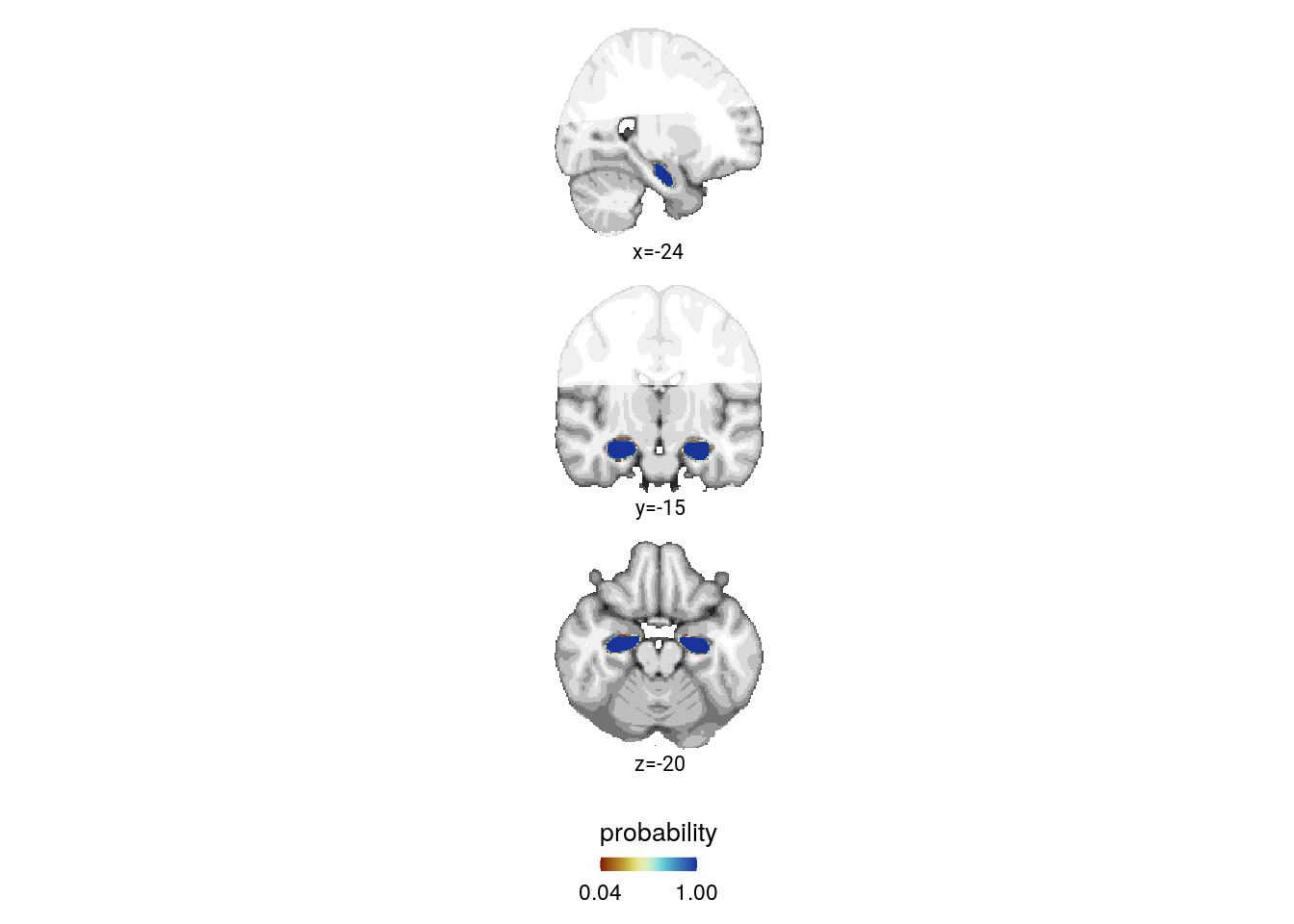# estimate marginal means for each model term by omitting the terms argument lmm_full_emm <- ggeffects::ggpredict(lmm_full, ci.lvl = 0.95) %>% get_complete_df() # plot marginal means sfigc <- ggplot(data = lmm_full_emm, aes(color = group)) + geom_line(aes(x, predicted)) + geom_ribbon(aes(x, ymin = conf.low, ymax = conf.high, fill = group), alpha = .2, linetype=0, show.legend = FALSE) + scale_color_manual(values = c("gray", unname(aHPC_colors["within_main"])), name = element_blank(), labels =c("first & last event", "virtual time")) + scale_fill_manual(values = c("gray", unname(aHPC_colors["within_main"]))) + ylab('estimated\nmarginal means') + xlab('fixed effect') + guides(fill = FALSE, color=FALSE) + theme_cowplot() sfigc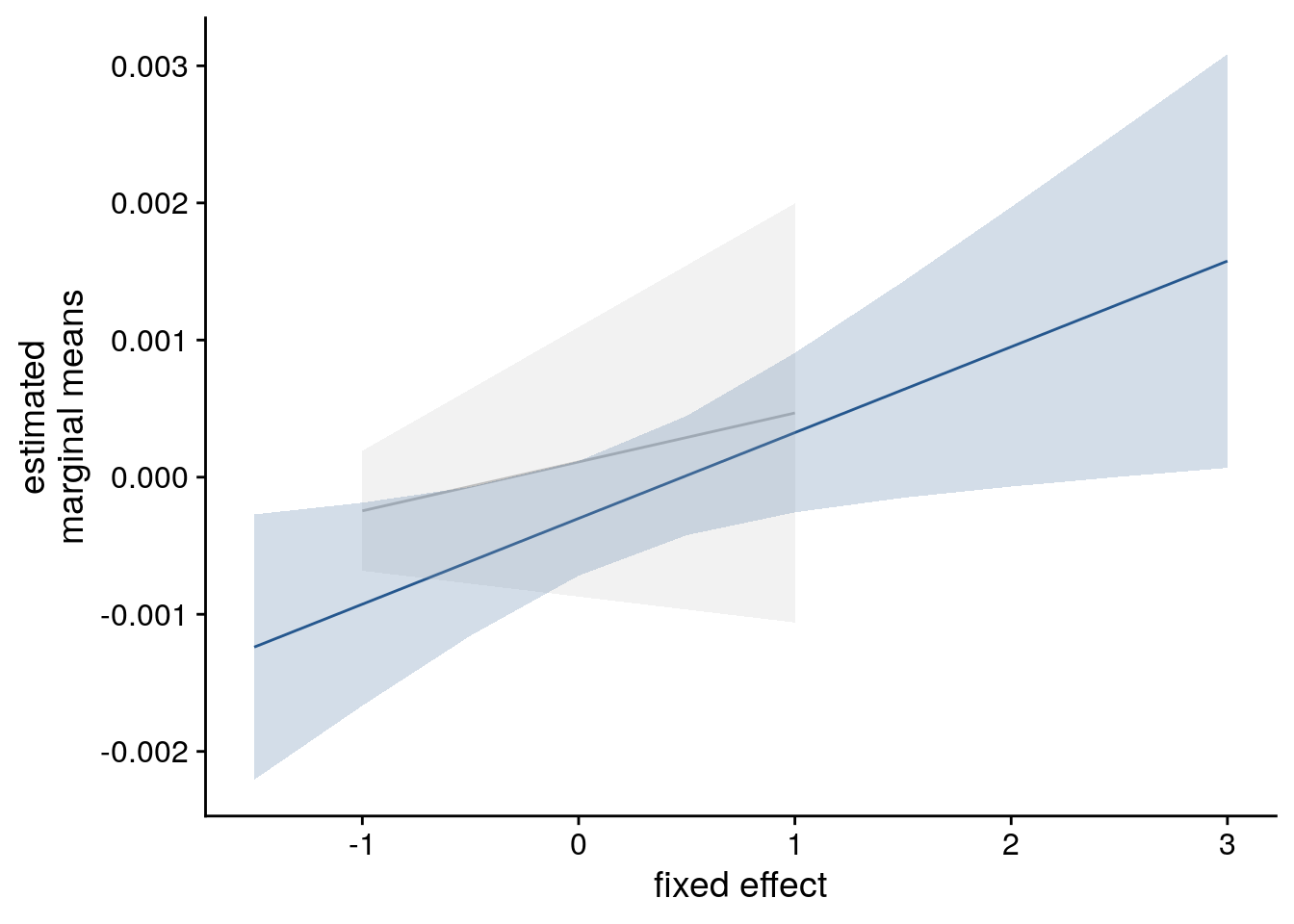##### LME model assumptions lmm_diagplots_jb(lmm_full)## 8.5 aHPC: virtual time within sequence when including order & real time We further tested whether the effect of virtual time on anterior hippocampal pattern similarity change persisted when including the absolute difference between sequence positions (“order”) and the interval in seconds between events (“real time”) as control predictors of no interest in the model. To follow up on the within-day effect in the hippocampus, let’s see if virtual correlates with pattern similarity change above and beyond the effects of order and real time. We will look at this in two ways. ### One model with multiple predictors The first way is to run models in which the three time metrics compete for variance. Temporal distances based on order and real time are control regressors of no interest, we test only the effect of virtual time for significance. #### Summary Statistics In the summary statistics approach, this comes down to a multiple regression on the participant-level. Again, we compute z-values for each regression coefficient from permutations of the dependent variable, which maintains the correlation structure of the regressors. Then we test the z-values for virtual time against 0 on the group level. set.seed(59) # set seed for reproducibility # extract all comparisons from the same day rsa_dat_same_day_aHPC <- rsa_dat %>% filter(roi == "aHPC_lr", same_day == TRUE) # do RSA using linear model and calculate z-score for model fit from permutations rsa_fit_aHPC_mult_reg <- rsa_dat_same_day_aHPC %>% group_by(sub_id) %>% # run the linear model do(z = lm_perm_jb(in_dat = ., lm_formula = "ps_change ~ vir_time_diff + order_diff + real_time_diff", nsim = n_perm)) %>% mutate(z = list(setNames(z, c("z_intercept", "z_virtual_time", "z_order", "z_real_time")))) %>% unnest_longer(z) %>% filter(z_id != "z_intercept") # run group-level t-tests on the RSA fits from the first level in aHPC for within-day stats <- rsa_fit_aHPC_mult_reg %>% filter(z_id =="z_virtual_time") %>% select(z) %>% paired_t_perm_jb (., n_perm = n_perm) # Cohen's d with Hedges' correction for one sample using non-central t-distribution for CI d<-cohen.d(d=(rsa_fit_aHPC_mult_reg %>% filter(z_id == "z_virtual_time"))$z, f=NA, paired=TRUE, hedges.correction=TRUE, noncentral=TRUE)
stats$d <- d$estimate
stats$dCI_low <- d$conf.int[]
stats$dCI_high <- d$conf.int[]

# print results
huxtable(stats) %>% theme_article()
Table 8.7:
estimatestatisticp.valuep_permparameterconf.lowconf.highmethodalternativeddCI_lowdCI_high
0.4372.180.03850.0401270.02480.849One Sample t-testtwo.sided0.3990.02180.808

Summary Statistics: t-test against 0 for virtual time within sequence in aHPC with order and real time in the model
t27=2.18, p=0.040, d=0.40, 95% CI [0.02, 0.81]

# make z_id a factor and reorder it
rsa_fit_aHPC_mult_reg <- rsa_fit_aHPC_mult_reg %>% mutate(
z_id = factor(z_id, levels = c("z_virtual_time", "z_order", "z_real_time")))

# raincloud plot
f4e <- ggplot(rsa_fit_aHPC_mult_reg, aes(x=z_id, y=z, fill = z_id, colour = z_id)) +
gghalves::geom_half_violin(position=position_nudge(0.1),
side = "r", color = NA) +
geom_point(aes(x = as.numeric(z_id)-0.2), alpha = 0.7,
position = position_jitter(width = .1, height = 0),
shape=16, size = 1) +
geom_boxplot(position = position_nudge(x = 0, y = 0),
width = .1, colour = "black", outlier.shape = NA) +
stat_summary(fun = mean, geom = "point", size = 1, shape = 16,
position = position_nudge(.1), colour = "black") +
stat_summary(fun.data = mean_se, geom = "errorbar",
position = position_nudge(.1), colour = "black", width = 0, size = 0.5)+
ylab('z RSA model fit') + xlab('time metric') +
scale_x_discrete(labels = c("virtual time", "order", "real time")) +
scale_color_manual(values=time_colors, name = "time metric",
labels = c("virtual time", "order", "real time")) +
scale_fill_manual(values=time_colors) +
annotate(geom = "text", x = 1, y = 2.2, label = "*", hjust = 0.5, size = 8/.pt, family=font2use) +
guides(fill=FALSE, color=guide_legend(override.aes=list(fill=NA, alpha = 1, size=2))) +
theme_cowplot() +
theme(text = element_text(size=10), axis.text = element_text(size=8),
legend.position = "none")
f4e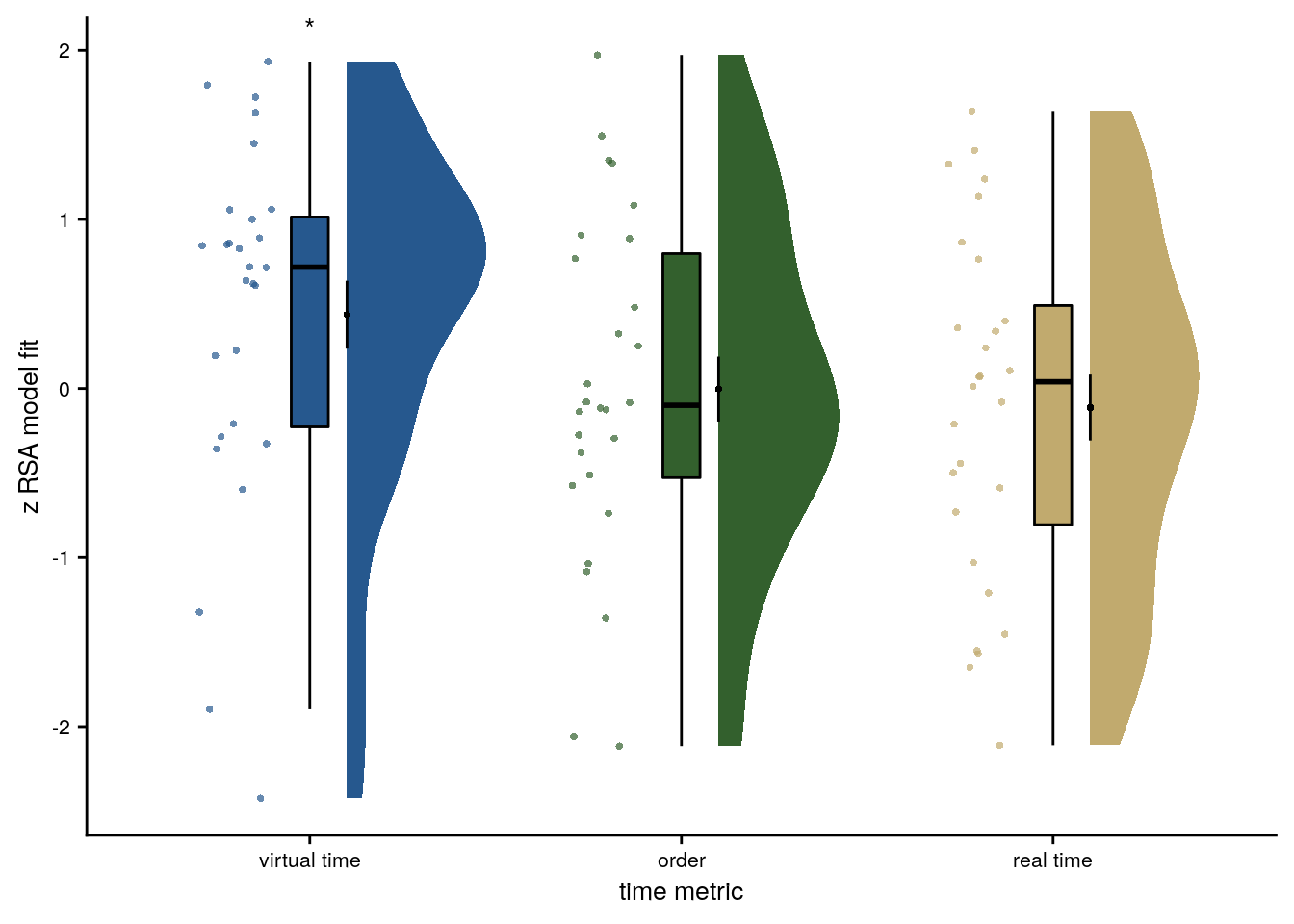#### Linear Mixed Effects

For the mixed model, we use order differences and real time differences as fixed effects in addition to virtual time. As a random effect structure we first include random intercepts and random slopes for each subject for all 3 fixed effects. This model is singular due to an estimated correlation of 1 between random slopes and random intercepts. We remove the random intercepts, but the fit is still singular. Removing the random slopes for the two fixed effects of no interest (order and real time) also does not help. We thus drop the random intercepts (as above) as well to end up with a model that converges without warnings.

Thus, the full model for this analysis has 3 fixed effects and random slopes for virtual time for each subject.

set.seed(87) # set seed for reproducibility

# extract the comparisons from the same day
rsa_dat_same_day <- rsa_dat %>% filter(roi == "aHPC_lr", same_day == TRUE)

# define the full model with virtual time difference, order difference and real time difference as
# fixed effects and random intercepts and random slopes for all effects
# fails to converge and is singular after restart
formula <- "ps_change ~ vir_time_diff + order_diff + real_time_diff + (1+vir_time_diff + order_diff + real_time_diff | sub_id)"
lmm_full <- lme4::lmer(formula, data = rsa_dat_same_day, REML = FALSE)
## boundary (singular) fit: see ?isSingular
#lmm_full <- update(lmm_full, start=getME(lmm_full, "theta"))

# remove the correlation between random slopes and random intercepts
formula <- "ps_change ~ vir_time_diff + order_diff + real_time_diff + (1+vir_time_diff + order_diff + real_time_diff || sub_id)"
lmm_full <- lme4::lmer(formula, data = rsa_dat_same_day, REML = FALSE)
## boundary (singular) fit: see ?isSingular
# thus we reduce the random effect structure by excluding random slopes for the fixed effects of no interest
# as above this results in a singular fit (the random intercept variance estimated to be 0)
formula <- "ps_change ~ vir_time_diff + order_diff + real_time_diff + (1+vir_time_diff | sub_id)"
lmm_full <- lme4::lmer(formula, data = rsa_dat_same_day, REML = FALSE)
## boundary (singular) fit: see ?isSingular
# next step is to remove the correlation between random slopes and random intercepts
formula <- "ps_change ~ vir_time_diff + order_diff + real_time_diff + (1+vir_time_diff || sub_id)"
lmm_full <- lme4::lmer(formula, data = rsa_dat_same_day, REML = FALSE)
## boundary (singular) fit: see ?isSingular
# we thus reduce further and keep only the random slopes for virtual time differences
# now the model converges without warnings
set.seed(332) # set seed for reproducibility
formula <- "ps_change ~ vir_time_diff + order_diff + real_time_diff + (0 + vir_time_diff | sub_id)"
lmm_full <- lme4::lmer(formula, data = rsa_dat_same_day, REML = FALSE)

summary(lmm_full, corr = FALSE)
## Linear mixed model fit by maximum likelihood  ['lmerMod']
## Formula: ps_change ~ vir_time_diff + order_diff + real_time_diff + (0 +      vir_time_diff | sub_id)
##    Data: rsa_dat_same_day
##
##      AIC      BIC   logLik deviance df.resid
##  -7950.8  -7920.6   3981.4  -7962.8     1114
##
## Scaled residuals:
##     Min      1Q  Median      3Q     Max
## -3.4923 -0.6444 -0.0269  0.6629  3.8017
##
## Random effects:
##  Groups   Name          Variance  Std.Dev.
##  sub_id   vir_time_diff 6.744e-08 0.0002597
##  Residual               4.778e-05 0.0069126
## Number of obs: 1120, groups:  sub_id, 28
##
## Fixed effects:
##                  Estimate Std. Error t value
## (Intercept)    -2.812e-04  2.191e-04  -1.284
## vir_time_diff   1.321e-03  5.415e-04   2.439
## order_diff      1.235e-05  9.077e-04   0.014
## real_time_diff -6.760e-04  1.019e-03  -0.663
# tidy summary of the fixed effects that calculates 95% CIs
lmm_full_bm <- broom.mixed::tidy(lmm_full, effects = "fixed", conf.int=TRUE, conf.method="profile")
## Computing profile confidence intervals ...
# tidy summary of the random effects
lmm_full_bm_re <- broom.mixed::tidy(lmm_full, effects = "ran_pars")

# one way of testing for significance is by comparing the likelihood against a simpler model.
# Here, we drop the effect of virtual time difference and run an ANOVA. See e.g. Bodo Winter tutorial
set.seed(321) # set seed for reproducibility
formula <- "ps_change ~ order_diff + real_time_diff + (0 + vir_time_diff | sub_id)"
lmm_no_vir_time <- lme4::lmer(formula, data = rsa_dat_same_day, REML = FALSE)
lmm_aov <- anova(lmm_no_vir_time, lmm_full)
lmm_aov
Table 8.8:
nparAICBIClogLikdevianceChisqDfPr(>Chisq)
5-7.95e+03-7.92e+033.98e+03-7.96e+03
6-7.95e+03-7.92e+033.98e+03-7.96e+035.9210.015

Mixed Model: Fixed effect of virtual time on aHPC pattern similarity change with order and real time in model $$\chi^2$$(1)=5.92, p=0.015

Make mixed model summary table that includes overview of fixed and random effects as well as the model comparison to the nested (reduced) model.

fe_names <- c("intercept", "virtual time", "order", "real time")
re_groups <- c(rep("participant",1), "residual")
re_names <- c("virtual time (SD)", "SD")

lmm_hux <- make_lme_huxtable(fix_df=lmm_full_bm,
ran_df = lmm_full_bm_re,
aov_mdl = lmm_aov,
fe_terms =fe_names,
re_terms = re_names,
re_groups = re_groups,
lme_form = gsub(" ", "", paste0(deparse(formula(lmm_full)),
collapse = "", sep="")),
caption = "Mixed Model: Virtual time explains representational change for same-sequence events in the anterior hippocampus when including order and real time in the model")

# convert the huxtable to a flextable for word export
stable_lme_aHPC_virtime_same_seq_time_metrics <- convert_huxtable_to_flextable(ht = lmm_hux)

# print to screen
theme_article(lmm_hux)
fixed effects term estimate SE t-value -0.000281 0.000219 -1.28 -0.000711 0.000149 0.001321 0.000541 2.44 0.000258 0.002383 0.000012 0.000908 0.01 -0.001768 0.001793 -0.000676 0.001019 -0.66 -0.002675 0.001323 0.000260 0.006913 -7946.84 3978.42 -7950.76 3981.38 5.92 1 0.015

Mixed model plots.

# set RNG
set.seed(23)

lmm_full_bm <- lmm_full_bm %>%
mutate(term=as.factor(term) %>%
factor(levels = c("vir_time_diff", "order_diff", "real_time_diff")))

# dot plot of Fixed Effect Coefficients with CIs
f4f <- ggplot(data = lmm_full_bm[2:4,], aes(x = term, color = term)) +
geom_hline(yintercept = 0, colour="black", linetype="dotted") +
geom_errorbar(aes(ymin = conf.low, ymax = conf.high, width = NA), size = 0.5) +
geom_point(aes(y = estimate), shape = 16, size = 1) +
scale_fill_manual(values = time_colors) +
scale_color_manual(values = time_colors, labels = c("Virtual Time", "Order", "Real Time")) +
labs(x = "time metric", y = "fixed effect\nestimate", color = "Time Metric") +
guides(color = FALSE, fill = FALSE) +
annotate(geom = "text", x = 1, y = 0.0027, label = "*", hjust = 0.5, size = 8/.pt, family=font2use) +
theme_cowplot() +
theme(plot.title = element_text(hjust = 0.5), axis.text.x=element_blank())
f4f# estimate marginal means for each model term by omitting the terms argument
lmm_full_emm <- ggeffects::ggpredict(lmm_full, ci.lvl = 0.95) %>% get_complete_df
# convert the group variable to a factor to control the order of facets below
lmm_full_emm$group <- factor(lmm_full_emm$group, levels = c("vir_time_diff", "order_diff", "real_time_diff"))

# plot marginal means
f4g <- ggplot(data = lmm_full_emm, aes(color = group)) +
geom_line(aes(x, predicted)) +
geom_ribbon(aes(x, ymin = conf.low, ymax = conf.high, fill = group), alpha = .2, linetype=0) +
scale_color_manual(values = time_colors, name = element_blank(),
labels = c("virtual time", "order", "real time")) +
scale_fill_manual(values = time_colors) +
ylab('estimated\nmarginal means') +
xlab('z time metric') +
guides(fill = FALSE, color=FALSE) +
theme_cowplot() +
theme(plot.title = element_text(hjust = 0.5), strip.background = element_blank(),
strip.text.x = element_blank())
f4g##### LME model assumptions
lmm_diagplots_jb(lmm_full)We are now ready to create figure 4 of the manuscript:

layoutBG = "
BCEEEF
BDEEEG"

layoutABG ="
ABBBBBB
ABBBBBB"

f4 <- f4a+
{f4b+f4c+f4d+f4e+f4f+f4g +
plot_layout(design = layoutBG, guides = "collect") &
theme(text = element_text(size=10, family=font2use),
axis.text = element_text(size=8),
legend.text=element_text(size=8),
legend.title=element_text(size=8),
legend.spacing.x = unit(1, 'mm'),
legend.key.size = unit(3,"mm"),
legend.position = 'bottom')} +
plot_layout(design = layoutABG, guides = "keep") &
plot_annotation(theme = theme(plot.margin = margin(t=0, r=7, b=0, l=-19, unit="pt")),
tag_levels = 'A')&
theme(#plot.tag = element_text(size = 10, face="bold"),
#plot.tag.position = "topleft",
#plot.margin=grid::unit(c(0,7,0,-20), "pt"), # need to add a bit of right margin because x-label extentds beyond plot area
text = element_text(size=10, family=font2use))
f4[] <- f4[] +
theme(legend.position = c(0.5,-0.1975),
legend.text=element_text(size=8),
legend.title=element_text(size=8),
plot.tag = element_text(margin = margin(l = 13)))

# save and print
fn <- here("figures", "f4")
ggsave(paste0(fn, ".pdf"), plot=f4, units = "cm",
width = 17.4, height = 10, dpi = "retina", device = cairo_pdf)
ggsave(paste0(fn, ".png"), plot=f4, units = "cm",
width = 17.4, height = 10, dpi = "retina", device = "png")
f4Figure 4. Sequence representations in anterior hippocampus reflect constructed event times. A. The anterior hippocampus region of interest is displayed on the MNI template with voxels outside the field of view shown in lighter shades of grey. Color code denotes probability of a voxel to be included in the mask based on participant-specific ROIs (see Methods). B. The Z-values based on permutation tests of participant-specific linear models assessing the effect of virtual time on pattern similarity change for event pairs from the same sequence were significantly positive. C. Dot plot shows the parameter estimate and 95% confidence interval for the fixed effect of virtual time on hippocampal pattern similarity change for same-sequence events from a linear mixed effects model. D. Estimated marginal means illustrate the positive relationship between virtual temporal distances and pattern similarity change. E. Z-values show the relationship of the different time metrics to representational change based on participant-specific multiple regression analyses. Virtual time predicts pattern similarity change with event order and real time in the model as control predictors of no interest. F,G. Parameter estimates with 95% confidence intervals (F) and estimated marginal means (G) show the fixed effects of the three time metrics from the corresponding mixed model. B,E. Circles are individual participant data; boxplots show median and upper/lower quartile along with whiskers extending to most extreme data point within 1.5 interquartile ranges above/below the upper/lower quartile; black circle with error bars corresponds to mean±S.E.M.; distributions show probability density function of data points. ** p<0.01; * p<0.05

### Virtual time explains residuals of order and real time

We implemented participant-specific regression analyses with order and real time distances as predictors of hippocampal pattern similarity change. The plot shows a significant effect of virtual temporal distances when tested on the residuals these linear models. Thus, variance that cannot be explained by the other time metrics can be accounted for by virtual temporal distances. This analysis was conducted only using the summary statistics approach because the residuals of a mixed model are more difficult to interpret than those of participant-specific regression analyses using ordinary least squares.

The second way of running this analysis is via a two-step procedure. We first predict pattern similarity change from the ordinal and real-time relationships and store the residuals, i.e. variance in pattern similarity change that cannot be explained by the effects of order and real time. Then, we test in a second model whether virtual time can explain these residuals.

#### Summary Statistics

The first step here is a multiple linear regression model with order and real time distances as predictors. We do not use permutations here because we are only interested in the residuals of this model. In the second step, we run a linear model with permutations to obtain z-values for group-level stats for the effect of virtual time on the residuals.

set.seed(102) # set seed for reproducibility

# extract all comparisons from the same day
rsa_dat_same_day_aHPC <- rsa_dat %>%
filter(roi == "aHPC_lr", same_day == TRUE)

# run multiple regression model with order and real time as predictors and store residuals
resids <- rsa_dat_same_day_aHPC %>% group_by(sub_id) %>%
# run the linear model
do(resids = residuals(lm(ps_change ~ order_diff + real_time_diff, data=.))) %>%
select(resids) %>%
unnest(resids)

# add to the original data frame
rsa_dat_same_day_aHPC$resids <- resids$resids

# do RSA using linear model and calculate z-score for model fit
rsa_fit_aHPC_mult_reg_resids <- rsa_dat_same_day_aHPC %>%
group_by(sub_id) %>%
# run the linear model
do(z = lm_perm_jb(in_dat = .,
lm_formula = "resids ~ vir_time_diff",
nsim = n_perm)) %>%
mutate(z = list(setNames(z, c("z_intercept",  "z_virtual_time")))) %>%
unnest_wider(z)

# run a group-level t-test on the RSA fits from the first level in aHPC for within-day
stats <- rsa_fit_aHPC_mult_reg_resids %>%
select(z_virtual_time) %>%
paired_t_perm_jb (., n_perm = n_perm)

# Cohen's d with Hedges' correction for one sample using non-central t-distribution for CI
d<-cohen.d(d=rsa_fit_aHPC_mult_reg_resids$z_virtual_time, f=NA, paired=TRUE, hedges.correction=TRUE, noncentral=TRUE) stats$d <- d$estimate stats$dCI_low <- d$conf.int[] stats$dCI_high <- d$conf.int[] # print results huxtable(stats) %>% theme_article() Table 8.10: estimatestatisticp.valuep_permparameterconf.lowconf.highmethodalternativeddCI_lowdCI_high 0.1772.230.03450.0343270.0140.341One Sample t-testtwo.sided0.4090.0310.819 Summary Statistics for virtual time on residuals of order and real time: t27=2.23, p=0.034, d=0.41, 95% CI [0.03, 0.82] We further observed that the residuals of linear models, in which hippocampal representational change was predicted from order and real time, were related to virtual temporal distances (Supplemental Figure 3D; t27=2.23, p=0.034, d=0.41, 95% CI [0.03, 0.82] ), demonstrating that virtual time accounts for variance that the other time metrics fail to explain. # select the data to plot plot_dat <- rsa_fit_aHPC_mult_reg_resids plot_dat$group <- factor(1)

# raincloud
sfigd <- ggplot(plot_dat, aes(x=1, y=z_virtual_time, color = group, fill=group)) +
gghalves::geom_half_violin(position=position_nudge(0.1),
side = "r", color = NA) +
geom_point(aes(x = 0.8, y = z_virtual_time), alpha = 0.7,
position = position_jitter(width = .1, height = 0),
shape=16, size = 1) +
geom_boxplot(position = position_nudge(x = 0, y = 0),
width = .1, colour = "black", outlier.shape = NA) +
stat_summary(fun = mean, geom = "point", size = 1, shape = 16,
position = position_nudge(.1), colour = "black") +
stat_summary(fun.data = mean_se, geom = "errorbar",
position = position_nudge(.1), colour = "black", width = 0, size = 0.5)+
scale_color_manual(values=unname(aHPC_colors["within_main"])) +
scale_fill_manual(values=unname(aHPC_colors["within_main"])) +
ylab('z RSA model fit') + xlab(element_blank()) +
annotate(geom = "text", x = 1, y = 0.9, label = "*", hjust = 0.5, size = 8/.pt, family=font2use) +
theme_cowplot() +
theme(text = element_text(size=10), axis.text = element_text(size=8),
legend.position = "none",
axis.ticks.x = element_blank(), axis.text.x = element_blank())
sfigd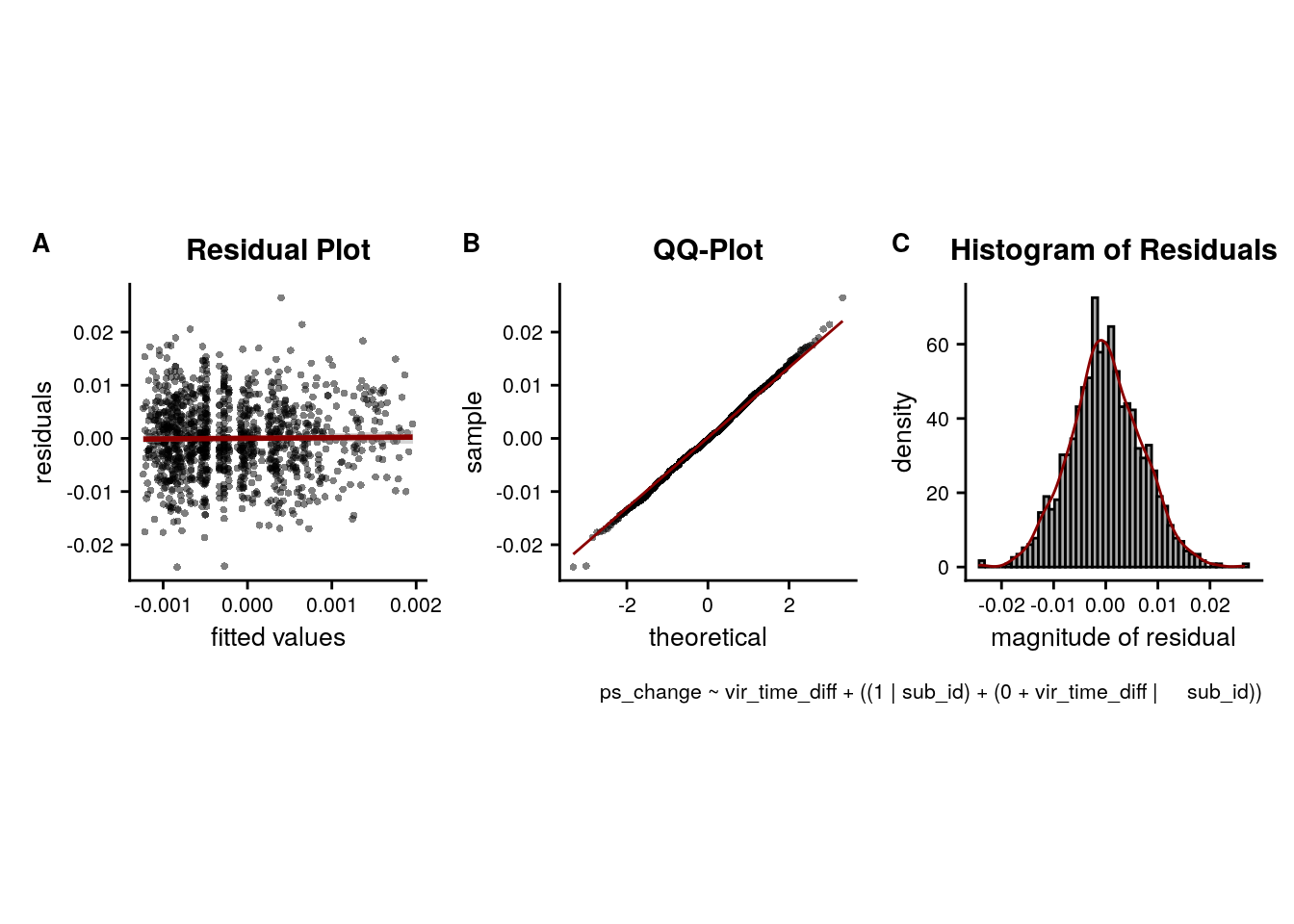#### Supplemental Figure

Let’s compose a figure from the above plots to summarize these results of the control analyses for the same-sequence effects.

layout = "
AAAABDD
AAAACDD"
sfig <- sfiga + sfigb + sfigc + sfigd +
plot_layout(design = layout, guides = 'collect') &
theme(text = element_text(size=10, family = font2use),
axis.text = element_text(size=8),
legend.position = "bottom") &
plot_annotation(theme = theme(plot.margin = margin(t=-3, r=-6, b=-7, l=-6, unit="pt")),
tag_levels = 'A')
sfig[] <- sfig[] + theme(legend.position = "none")
# save and print to screen
fn <- here("figures", "sf03")
ggsave(paste0(fn, ".pdf"), plot=sfig, units = "cm",
width = 17.4, height = 10, dpi = "retina", device = cairo_pdf)
ggsave(paste0(fn, ".png"), plot=sfig, units = "cm",
width = 17.4, height = 10, dpi = "retina", device = "png")
sfig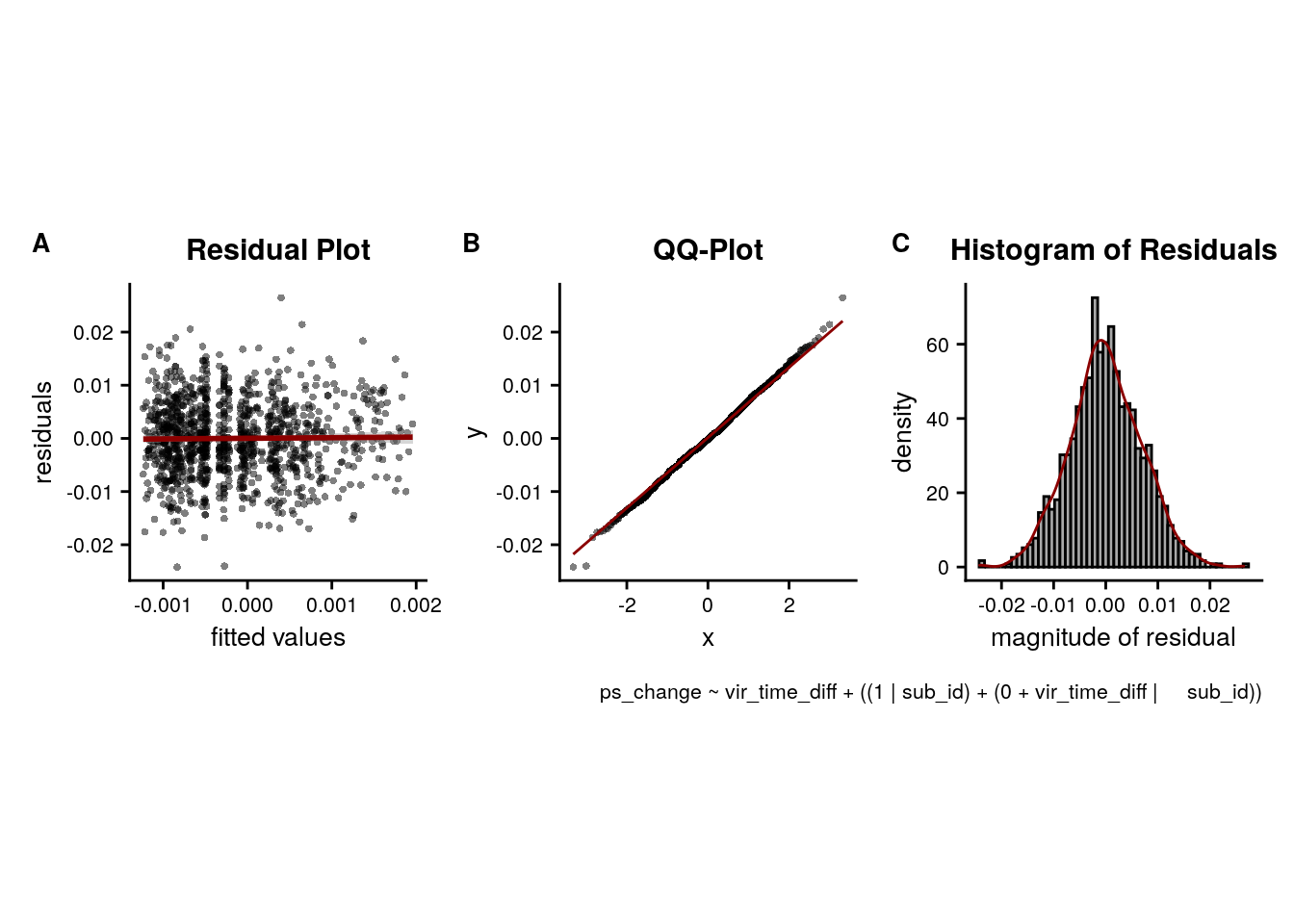Supplemental Figure 3. The relationship of virtual time and hippocampal pattern similarity change is not driven by the first and last event of a sequence. A. Z-values from the summary statistics approach show a significant positive effect of virtual time on pattern similarity change in the anterior hippcampus when competing for variance with a control predictor of no interest modelling pairs of events made up from the first and last event of the same sequence. B, C. Fixed effect estimate with 95% confidence intervals (B) and estimated marginal means (C) visualize the results of corresponding mixed model. D. We implemented participant-specific regression analyses with order and real time distances as predictors of hippocampal pattern similarity change. The plot shows a significant effect of virtual temporal distances when tested on the residuals of these linear models. Thus, variance that cannot be explained by the other time metrics can be accounted for by virtual temporal distances. This analysis was conducted only using the summary statistics approach because the residuals of a mixed model are more difficult to interpret than those of participant-specific regression analyses using ordinary least squares. A,D. Circles show individual participant Z-values from the summary statistics approach; boxplot shows median and upper/lower quartile along with whiskers extending to most extreme data point within 1.5 interquartile ranges above/below the upper/lower quartile; black circle with error bars corresponds to mean±S.E.M.; distribution shows probability density function of data points. * p<0.05

## 8.6 aHPC: virtual time across sequences

Having established that the anterior hippocampus forms sequence memories shaped by event relations in virtual time, we want to ask whether the hippocampus also generalizes across sequences. So next, we focus on pattern similarity changes for events that stem from different virtual days.

We separately tested the effect of virtual time for event pairs from the same or different sequences and thus use a Bonferroni-corrected α-level of 0.025 for these tests.

### Summary statistics

set.seed(103) # set seed for reproducibility

# run a group-level t-test on the RSA fits from the first level in aHPC for across-day
stats <- rsa_fit %>%
filter(roi == "aHPC_lr", same_day == FALSE) %>%
select(z_virtual_time) %>%
paired_t_perm_jb (., n_perm = n_perm)

# Cohen's d with Hedges' correction for one sample using non-central t-distribution for CI
d<-cohen.d(d=(rsa_fit %>% filter(roi == "aHPC_lr", same_day == FALSE))$z_virtual_time, f=NA, paired=TRUE, hedges.correction=TRUE, noncentral=TRUE) stats$d <- d$estimate stats$dCI_low <- d$conf.int[] stats$dCI_high <- d$conf.int[] # print results huxtable(stats) %>% theme_article() Table 8.11: estimatestatisticp.valuep_permparameterconf.lowconf.highmethodalternativeddCI_lowdCI_high -0.5-2.650.01330.012827-0.887-0.113One Sample t-testtwo.sided-0.486-0.906-0.105 Summary Statistics: t-test against 0 for virtual time across sequences in aHPC t27=-2.65, p=0.013, d=-0.49, 95% CI [-0.91, -0.10] # select the data to plot plot_dat <- rsa_fit %>% filter(roi == "aHPC_lr", same_day == FALSE) ggplot(plot_dat, aes(x=1, y=z_virtual_time, fill = roi, color = roi)) + gghalves::geom_half_violin(position=position_nudge(0.1), side = "r", color = NA) + geom_point(aes(x = 0.8, y = z_virtual_time), alpha = 0.7, position = position_jitter(width = .1, height = 0), shape=16, size = 1) + geom_boxplot(position = position_nudge(x = 0, y = 0), width = .1, colour = "black", outlier.shape = NA) + stat_summary(fun = mean, geom = "point", size = 1, shape = 16, position = position_nudge(.1), colour = "black") + stat_summary(fun.data = mean_se, geom = "errorbar", position = position_nudge(.1), colour = "black", width = 0, size = 0.5)+ scale_color_manual(values=unname(aHPC_colors["across_main"])) + scale_fill_manual(values=unname(aHPC_colors["across_main"])) + ylab('z RSA model fit') + xlab(element_blank()) + guides(fill=FALSE, color=FALSE) + annotate(geom = "text", x = 1, y = 1.8, label = "**", hjust = 0.5, size = 8/.pt, family=font2use) + theme_cowplot() + theme(text = element_text(size=10), axis.text = element_text(size=8), legend.position = "none", axis.ticks.x = element_blank(), axis.text.x = element_blank())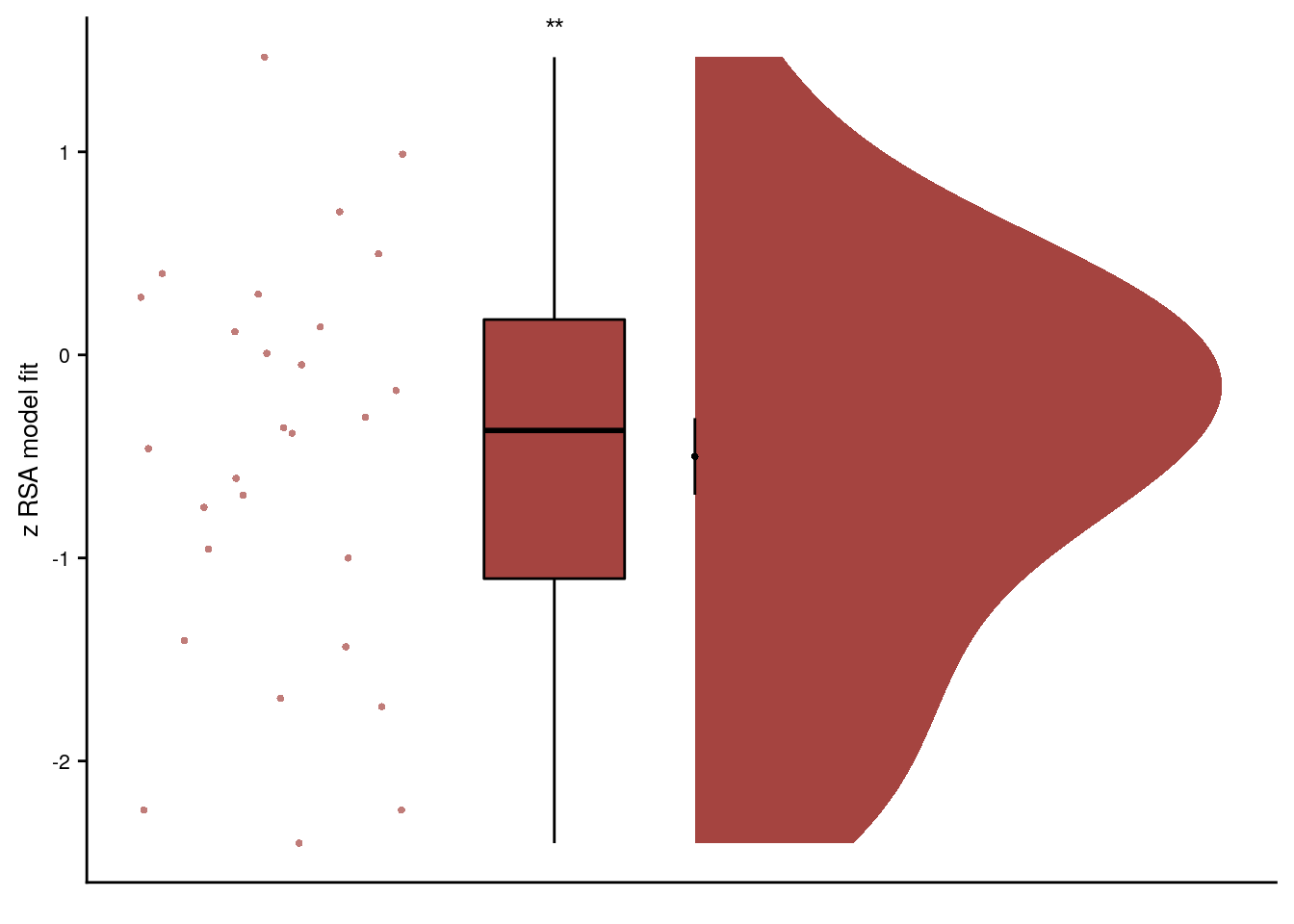### Linear Mixed Effects Here, we fit a model with virtual time as the predictor for pattern similarity change for the across-day comparisons in the anterior hippocampus. We start with a full random effects structure including random slopes and random intercepts for each subject. We reduce the random effects structure, but unfortunately even a simple model with only random slopes for the effect of virtual time results in a singular fit warning; even when trying different optimizers. We keep the random slopes to account for the within-subject dependencies in the data. # extract the comparisons from the same day rsa_dat_diff_day <- rsa_dat %>% filter(roi == "aHPC_lr", same_day == FALSE) # define the full model with virtual time difference as # fixed effect and by-subject random slopes and random intercepts --> singular fit formula <- "ps_change ~ vir_time_diff + (1 + vir_time_diff | sub_id)" lmm_full <- lme4::lmer(formula, data = rsa_dat_diff_day, REML = FALSE) ## boundary (singular) fit: see ?isSingular # remove correlation --> still singular formula <- "ps_change ~ vir_time_diff + (1 + vir_time_diff || sub_id)" lmm_full <- lme4::lmer(formula, data = rsa_dat_diff_day, REML = FALSE) ## boundary (singular) fit: see ?isSingular # only random slopes set.seed(389) # set seed for reproducibility formula <- "ps_change ~ vir_time_diff + (0 + vir_time_diff | sub_id)" lmm_full <- lme4::lmer(formula, data = rsa_dat_diff_day, REML = FALSE, #control = lmerControl(optimizer ='optimx', optCtrl=list(method='nlminb')) #control = lmerControl(optimizer ="bobyqa", optCtrl=list(maxfun=40000)) control = lmerControl(optimizer ='nloptwrap', optCtrl=list(method='NLOPT_LN_NELDERMEAD', maxfun=100000)) ) ## boundary (singular) fit: see ?isSingular summary(lmm_full, corr = FALSE) ## Linear mixed model fit by maximum likelihood ['lmerMod'] ## Formula: ps_change ~ vir_time_diff + (0 + vir_time_diff | sub_id) ## Data: rsa_dat_diff_day ## Control: lmerControl(optimizer = "nloptwrap", optCtrl = list(method = "NLOPT_LN_NELDERMEAD", maxfun = 1e+05)) ## ## AIC BIC logLik deviance df.resid ## -29625.4 -29600.0 14816.7 -29633.4 4196 ## ## Scaled residuals: ## Min 1Q Median 3Q Max ## -4.3020 -0.6877 0.0324 0.6564 3.5548 ## ## Random effects: ## Groups Name Variance Std.Dev. ## sub_id vir_time_diff 0.00e+00 0.000000 ## Residual 5.05e-05 0.007107 ## Number of obs: 4200, groups: sub_id, 28 ## ## Fixed effects: ## Estimate Std. Error t value ## (Intercept) -6.075e-05 1.098e-04 -0.553 ## vir_time_diff -2.745e-04 1.096e-04 -2.505 ## convergence code: 0 ## boundary (singular) fit: see ?isSingular # tidy summary of the fixed effects that calculates 95% CIs lmm_full_bm <- broom.mixed::tidy(lmm_full, effects = "fixed", conf.int=TRUE, conf.method="profile", optimizer = 'nloptwrap') ## Computing profile confidence intervals ... # tidy summary of the random effects lmm_full_bm_re <- broom.mixed::tidy(lmm_full, effects = "ran_pars") # one way of testing for significance is by comparing the likelihood against a simpler model. # Here, we drop the effect of virtual time difference and run an ANOVA. See e.g. Bodo Winter tutorial set.seed(328) # set seed for reproducibility formula <- "ps_change ~ 1 + (0 + vir_time_diff | sub_id)" lmm_no_vir_time <- lme4::lmer(formula, data = rsa_dat_diff_day, REML = FALSE) lmm_aov <-anova(lmm_no_vir_time, lmm_full) Mixed Model: Fixed effect of virtual time across sequences on aHPC pattern similarity change $$\chi^2$$(1)=6.01, p=0.014 Make mixed model summary table that includes overview of fixed and random effects as well as the model comparison to the nested (reduced) model. fe_names <- c("intercept", "virtual time") re_groups <- c(rep("participant",1), "residual") re_names <- c("virtual time (SD)", "SD") lmm_hux <- make_lme_huxtable(fix_df=lmm_full_bm, ran_df = lmm_full_bm_re, aov_mdl = lmm_aov, fe_terms =fe_names, re_terms = re_names, re_groups = re_groups, lme_form = gsub(" ", "", paste0(deparse(formula(lmm_full)), collapse = "", sep="")), caption = "Mixed Model: Virtual time explains representational change for different-sequence events in the anterior hippocampus") # convert the huxtable to a flextable for word export stable_lme_aHPC_virtime_diff_seq <- convert_huxtable_to_flextable(ht = lmm_hux) # print to screen theme_article(lmm_hux) fixed effects term estimate SE t-value -0.000061 0.000110 -0.55 -0.000276 0.000155 -0.000275 0.000110 -2.51 -0.000491 -0.000058 0.000000 0.007107 -29621.39 14813.69 -29625.40 14816.70 6.01 1 0.014 ### PLOTS lmm_full_bm <- lmm_full_bm %>% mutate(term=as.factor(term)) # dot plot of Fixed Effect Coefficients with CIs sfiga <-ggplot(data = lmm_full_bm[2,], aes(x = term, color = term)) + geom_hline(yintercept = 0, colour="black", linetype="dotted") + geom_errorbar(aes(ymin = conf.low, ymax = conf.high, width = NA), size = 0.5) + geom_point(aes(y = estimate), shape = 16, size = 1) + scale_fill_manual(values = unname(aHPC_colors["across_main"])) + scale_color_manual(values = unname(aHPC_colors["across_main"]), labels = c("Virtual Time")) + labs(x = element_blank(), y = "fixed effect\nestimate", color = "") + guides(fill=FALSE, color=guide_legend(override.aes=list(fill=NA, alpha = 1, size=2, linetype=0))) + annotate(geom = "text", x = 1, y = -0.00003, label = "*", hjust = 0.5, size = 8/.pt, family=font2use) + theme_cowplot() + theme(legend.position = "none", axis.text.x=element_blank()) sfiga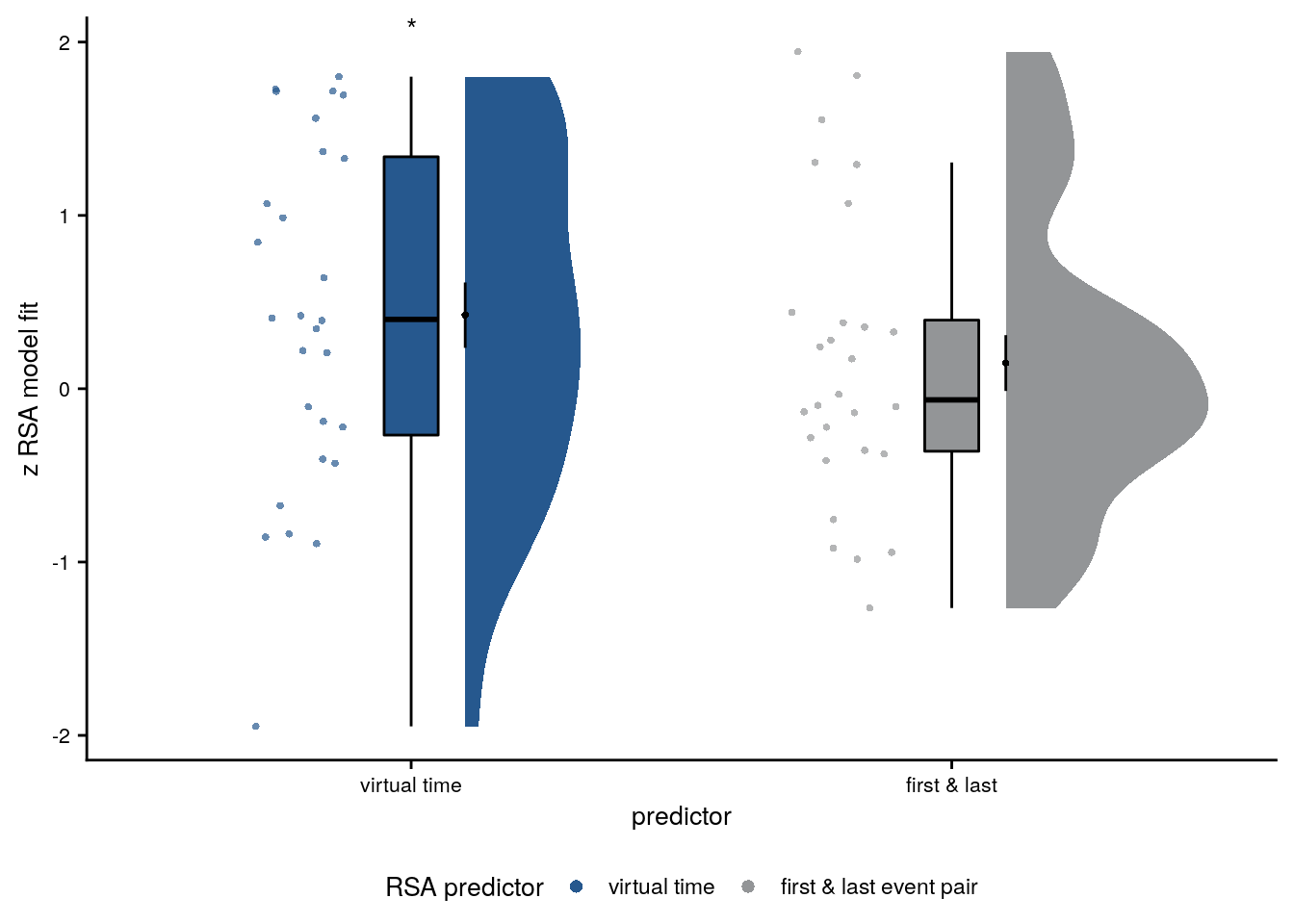# estimate marginal means for each model term by omitting the terms argument lmm_full_emm <- ggeffects::ggpredict(lmm_full, ci.lvl = 0.95) %>% ggeffects::get_complete_df() # plot marginal means sfigb <- ggplot(data = lmm_full_emm[lmm_full_emm$group == "vir_time_diff",], aes(color = group)) +
geom_line(aes(x, predicted)) +
geom_ribbon(aes(x, ymin = conf.low, ymax = conf.high, fill = group), alpha = .2, linetype=0) +
scale_color_manual(values = unname(aHPC_colors["across_main"]),
name = element_blank(), labels = "virtual time") +
scale_fill_manual(values = unname(aHPC_colors["across_main"])) +
ylab('estimated\nmarginal means') +
xlab('z time metric') +
#ggtitle("Estimated marginal means") +
guides(fill=FALSE, color=FALSE) +
theme_cowplot() +
theme(legend.position = "none")
sfigb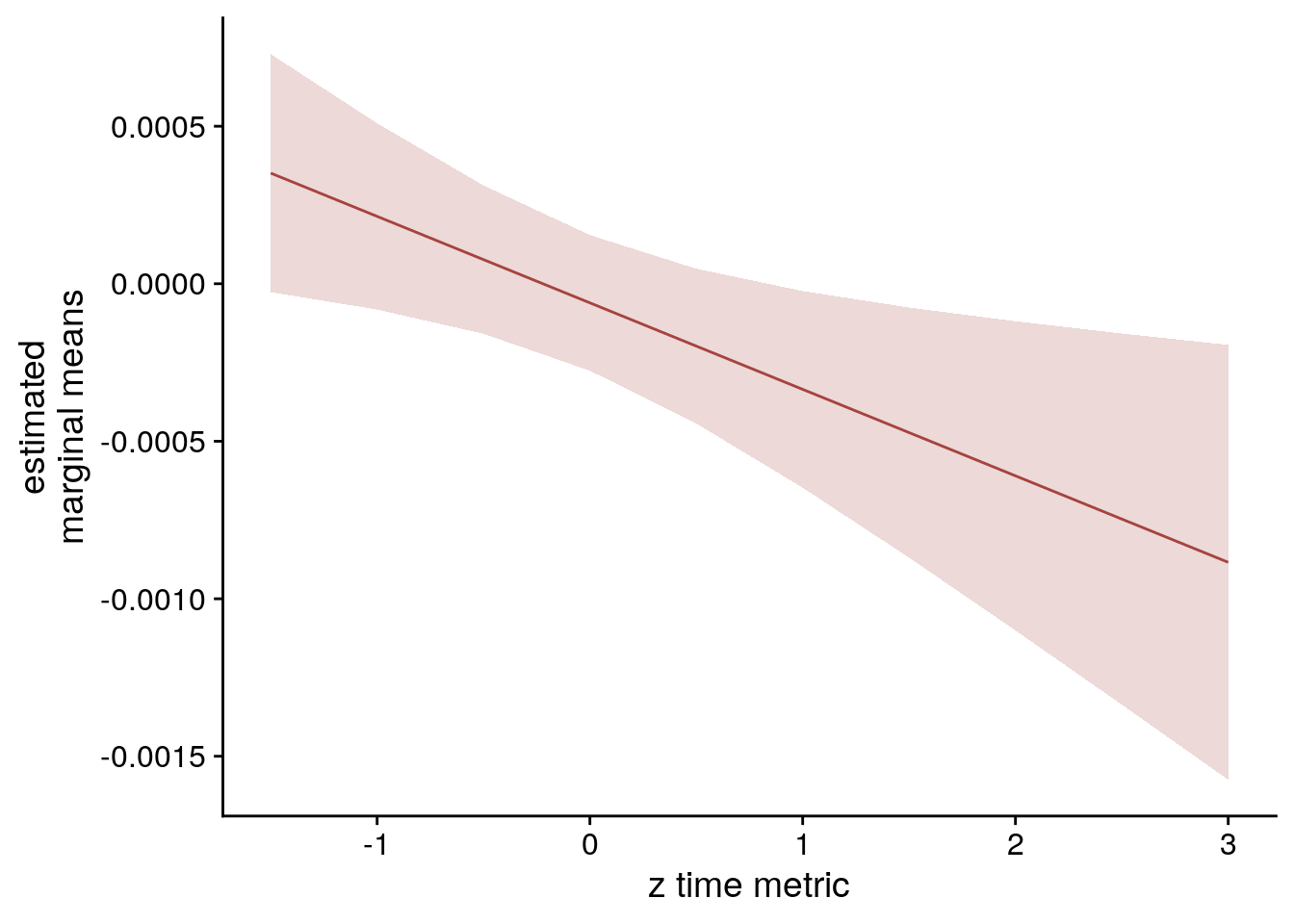#### LME model assumptions

lmm_diagplots_jb(lmm_full)## 8.7 aHPC: virtual time within vs. across sequences

The previous results show positive correlations of pattern similarity change with temporal distances between event pairs from the same sequence and negative correlations with temporal distances when events are from different sequences. Next, we want to quantify whether these two modes of representation are significantly different from each other.

### Second-level analysis

For the summary statistics approach, we run a permutation-based paired t-test to check for a difference in the effect of virtual time depending on whether we look at within vs. across sequences.

set.seed(105) # set seed for reproducibility

stats <- rsa_fit %>%
filter(roi=="aHPC_lr") %>%
# calculate difference between within- an across-day effect for each participant
group_by(sub_id) %>%
summarise(
same_day_diff = z_virtual_time[same_day==TRUE]-z_virtual_time[same_day==FALSE],
.groups = "drop") %>%
# test difference against 0
select(same_day_diff) %>%
paired_t_perm_jb (., n_perm = n_perm)

# Cohen's d with Hedges' correction for paired samples using non-central t-distribution for CI
d<-cohen.d(d=(rsa_fit %>% filter(roi == "aHPC_lr", same_day == TRUE))$z_virtual_time, f=(rsa_fit %>% filter(roi == "aHPC_lr", same_day == FALSE))$z_virtual_time,
paired=TRUE, pooled =TRUE, hedges.correction=TRUE, noncentral=TRUE)
stats$d <- d$estimate
stats$dCI_low <- d$conf.int[]
stats$dCI_high <- d$conf.int[]

# print results
huxtable(stats) %>% theme_article()
Table 8.13:
estimatestatisticp.valuep_permparameterconf.lowconf.highmethodalternativeddCI_lowdCI_high
1.123.710.0009410.001270.51.74One Sample t-testtwo.sided1.050.2871.13

Summary Statistics: paired t-test comparing effects of virtual time within and across sequences in aHPC
t27=3.71, p=0.001, d=1.05, 95% CI [0.29, 1.13]

To visualize the difference between the effects of virtual time for events from the same or different day, we create a pair of rainclouds facing each other. We use a custom jitter so that the length of lines connecting individual subject data points remains constant, thus keeping their slopes comparable.

# generate custom repeating jitter (multiply with -1/1 to shift towards each other)
plot_dat <- rsa_fit %>%
filter(roi=="aHPC_lr") %>%
mutate(
same_day_num = plyr::mapvalues(same_day, from = c("FALSE", "TRUE"),to = c(1,0)),
same_day_char = plyr::mapvalues(same_day, from = c("FALSE", "TRUE"),
to = c("different", "same")),
same_day_char = fct_relevel(same_day_char, c("same", "different")),
x_jit = as.numeric(same_day_char) + rep(jitter(rep(0,n_subs), amount=0.05), each=2) * rep(c(-1,1),n_subs))

f5a<- ggplot(data=plot_dat, aes(x=same_day_char, y=z_virtual_time, fill = same_day_char, color = same_day_char)) +
geom_boxplot(aes(group=same_day_char), position = position_nudge(x = 0, y = 0),
width = .1, colour = "black", outlier.shape = NA) +
scale_fill_manual(values = unname(c(aHPC_colors["within_main"], aHPC_colors["across_main"]))) +
scale_color_manual(values = unname(c(aHPC_colors["within_main"], aHPC_colors["across_main"])),
name = "sequence", labels=c("same", "different")) +
gghalves::geom_half_violin(data = plot_dat %>% filter(same_day == TRUE),
aes(x=same_day_char, y=z_virtual_time),
position=position_nudge(-0.1),
side = "l", color = NA) +
gghalves::geom_half_violin(data = plot_dat %>% filter(same_day == FALSE),
aes(x=same_day_char, y=z_virtual_time),
position=position_nudge(0.1),
side = "r", color = NA) +
stat_summary(fun = mean, geom = "point", size = 1, shape = 16,
position = position_nudge(c(-0.1, 0.1)), colour = "black") +
stat_summary(fun.data = mean_se, geom = "errorbar",
position = position_nudge(c(-0.1, 0.1)), colour = "black", width = 0, size = 0.5) +
geom_line(aes(x = x_jit, group=sub_id,), color = ultimate_gray,
position = position_nudge(c(0.15, -0.15))) +
geom_point(aes(x=x_jit, fill = same_day_char), position = position_nudge(c(-0.15, 0.15)),
shape=16, size = 1) +
ylab('z RSA model fit') + xlab('sequence') +
annotate(geom = "line",x=c(1.3, 1.7), y=2.5, size=0.5) +
annotate(geom = "text", x = c(1, 1.5, 2), y = c(2.6, 2.6, 1.7),
label = c("**", "***", "*"), hjust = 0.5, size = 8/.pt, family=font2use) +
theme_cowplot() +
theme(text = element_text(size=10), axis.text = element_text(size=8),
legend.position = "none")
f5a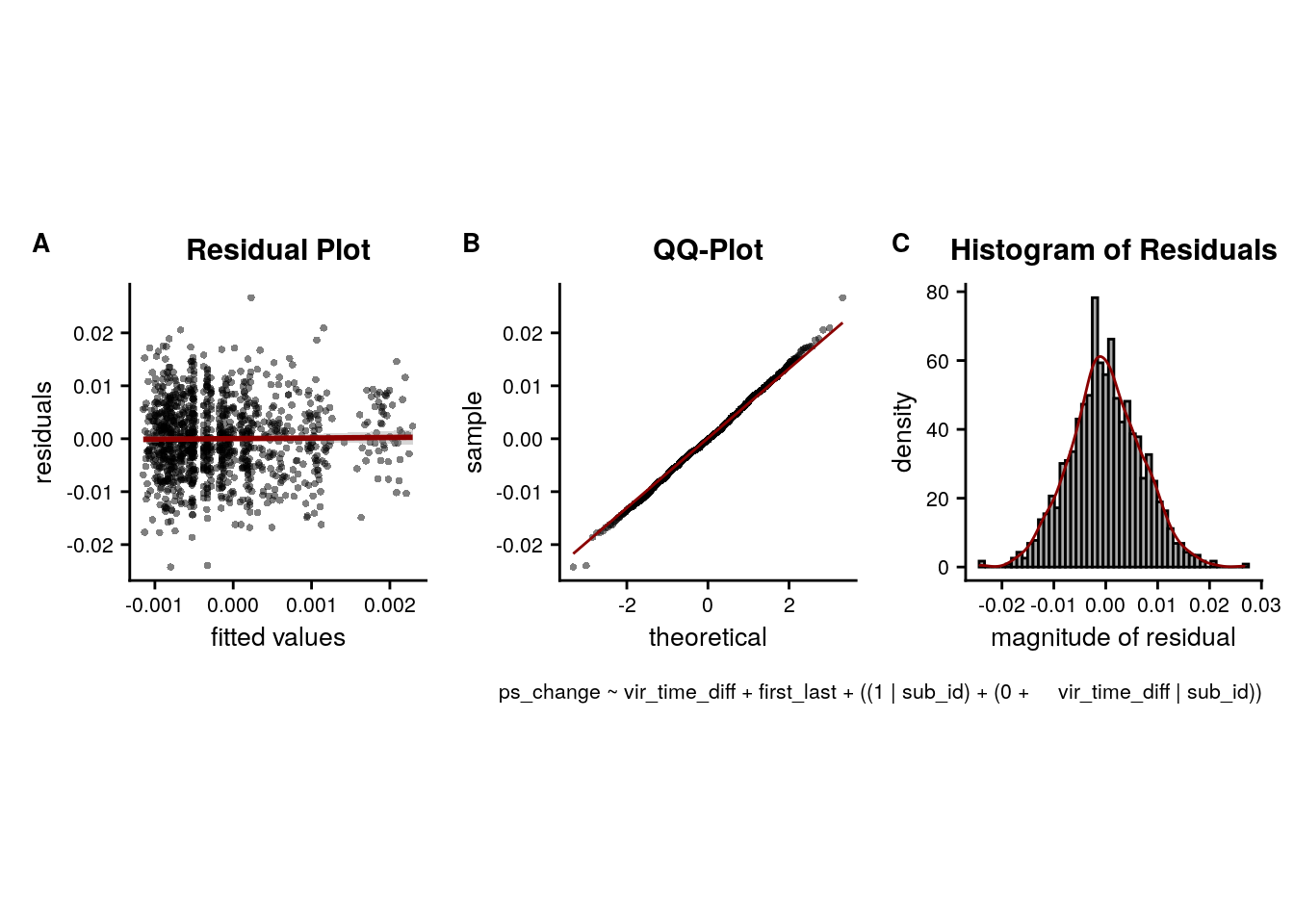### Linear Mixed Effects

Next, we test whether there is an interaction effect, i.e. we test whether the effect of virtual time on hippocampal pattern similarity change depends on whether we look at event pairs from the same vs. from different days.

We again have to reduce our model from the maximal random effects structure. We end up with a model that keeps only the random slopes for the interaction of virtual time and day. This is in keeping with the paper on random effect structures when testing interactions that emphasizes the importance of random slopes for the interaction of interest Barr, 2013, Frontiers Psychology.

# get the data
rsa_dat_aHPC <- rsa_dat %>% filter(roi == "aHPC_lr")

# test the interaction for aHPC
# maximal random effect structure --> singular fit
formula <- "ps_change ~ vir_time_diff * same_day_dv + (1 + vir_time_diff * same_day_dv | sub_id)"
lmm_full = lme4::lmer(formula, data=rsa_dat_aHPC, REML=FALSE)
## boundary (singular) fit: see ?isSingular
# no correlations with random intercept --> singular fit
formula <- "ps_change ~ vir_time_diff * same_day_dv + (1 + vir_time_diff * same_day_dv || sub_id)"
lmm_full = lme4::lmer(formula, data=rsa_dat_aHPC, REML=FALSE)
## boundary (singular) fit: see ?isSingular
# random intercepts and random slopes only for the critical interaction --> singular fit
formula <- "ps_change ~ vir_time_diff * same_day_dv + (1 + vir_time_diff : same_day_dv || sub_id)"
lmm_full = lme4::lmer(formula, data=rsa_dat_aHPC, REML=FALSE)
## boundary (singular) fit: see ?isSingular
# random slopes for critical interactions
set.seed(334) # set seed for reproducibility
formula <- "ps_change ~ vir_time_diff * same_day_dv + (0 + vir_time_diff : same_day_dv | sub_id)"
lmm_full = lme4::lmer(formula, data=rsa_dat_aHPC, REML=FALSE)
summary(lmm_full, corr = FALSE)
## Linear mixed model fit by maximum likelihood  ['lmerMod']
## Formula: ps_change ~ vir_time_diff * same_day_dv + (0 + vir_time_diff:same_day_dv |      sub_id)
##    Data: rsa_dat_aHPC
##
##      AIC      BIC   logLik deviance df.resid
## -37581.7 -37542.3  18796.9 -37593.7     5314
##
## Scaled residuals:
##     Min      1Q  Median      3Q     Max
## -4.3282 -0.6823  0.0196  0.6564  3.7447
##
## Random effects:
##  Groups   Name                      Variance  Std.Dev.
##  sub_id   vir_time_diff:same_day_dv 3.083e-08 0.0001756
##  Residual                           4.993e-05 0.0070659
## Number of obs: 5320, groups:  sub_id, 28
##
## Fixed effects:
##                             Estimate Std. Error t value
## (Intercept)               -0.0001934  0.0001209  -1.600
## vir_time_diff              0.0002384  0.0001223   1.950
## same_day_dv               -0.0001327  0.0001209  -1.098
## vir_time_diff:same_day_dv  0.0005129  0.0001267   4.049
# tidy summary of the fixed effects that calculates 95% CIs
lmm_full_bm <- broom.mixed::tidy(lmm_full, effects = "fixed", conf.int=TRUE, conf.method="profile")
## Computing profile confidence intervals ...
# tidy summary of the random effects
lmm_full_bm_re <- broom.mixed::tidy(lmm_full, effects = "ran_pars")

# test against reduced model
set.seed(309) # set seed for reproducibility
lmm_reduced <- update(lmm_full, ~ . - vir_time_diff:same_day_dv)
lmm_aov <- anova(lmm_reduced, lmm_full)

Mixed Model: Fixed effect of interaction of virtual time with sequence identity on aHPC pattern similarity change $$\chi^2$$(1)=14.37, p=0.000

Make mixed model summary table that includes overview of fixed and random effects as well as the model comparison to the nested (reduced) model.

fe_names <- c("intercept", "virtual time", "day", "interaction virtual time and day")
re_groups <- c(rep("participant",1), "residual")
re_names <- c("interaction virtual time and day (SD)", "SD")

lmm_hux <- make_lme_huxtable(fix_df=lmm_full_bm,
ran_df = lmm_full_bm_re,
aov_mdl = lmm_aov,
fe_terms =fe_names,
re_terms = re_names,
re_groups = re_groups,
lme_form = gsub(" ", "", paste0(deparse(formula(lmm_full)),
collapse = "", sep="")),
caption = "Mixed Model: The effect of virtual time differs between same-sequence and different-sequence events in the anterior hippocampus")

# convert the huxtable to a flextable for word export
stable_lme_aHPC_virtime_same_vs_diff_seq <- convert_huxtable_to_flextable(ht = lmm_hux)

# print to screen
theme_article(lmm_hux)
fixed effects term estimate SE t-value -0.000193 0.000121 -1.60 -0.000430 0.000044 0.000238 0.000122 1.95 -0.000001 0.000478 -0.000133 0.000121 -1.10 -0.000370 0.000104 0.000513 0.000127 4.05 0.000261 0.000765 0.000176 0.007066 -37569.38 18789.69 -37581.75 18796.87 14.37 1 1.50e-04
lmm_full_bm <- lmm_full_bm %>%
mutate(term=as.factor(term))

# dot plot of Fixed Effect Coefficients with CIs
f5b <- ggplot(data = lmm_full_bm[2:4,], aes(x = term, color = term)) +
geom_hline(yintercept = 0, colour="black", linetype="dotted") +
geom_errorbar(aes(ymin = conf.low, ymax = conf.high, width = NA), size = 0.5) +
geom_point(aes(y = estimate), shape = 16, size = 1) +
scale_fill_manual(values = c(rep("black",6), "#DD8D29")) +
scale_color_manual(values = c(ultimate_gray, time_within_across_color, day_time_int_color), name = "mixed model predictor",
labels = c("sequence", "virtual time", "interaction")) +
labs(x = element_blank(), y = "fixed effect\nestimate") +
theme_cowplot() +
guides(fill=FALSE, color=guide_legend(override.aes=list(fill=NA, alpha = 1, size=2, linetype=0), title.position = "top")) +
annotate(geom = "text", x = 3, y = 0.00081, label = "***", hjust = 0.5, size = 8/.pt, family=font2use) +
theme(plot.title = element_text(hjust = 0.5), axis.text.x=element_blank(), legend.position = "bottom",
legend.margin=margin(t=-0.5, b=-0.2, l = 1, unit='cm'))
f5b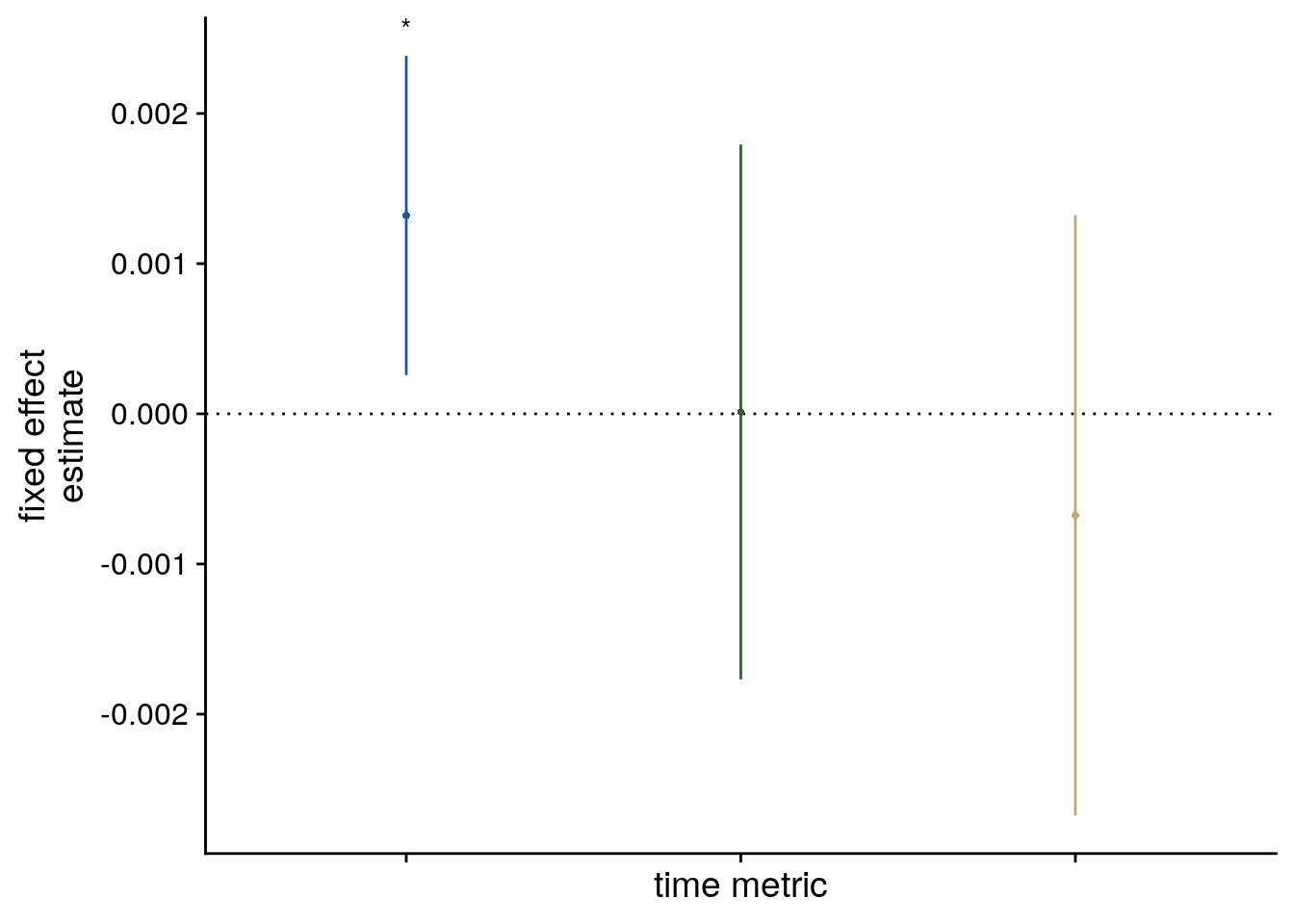# estimate marginal means (& add factor for easier plotting)
hpc_emms <- ggeffects::ggpredict(lmm_full, terms = c("vir_time_diff", "same_day_dv")) %>%
as_tibble() %>%
mutate(day = factor(if_else(group == 1, true = "same day", false = "different day"),
levels = c("same day", "different day")))

# plot marginal means
f5c <- ggplot(hpc_emms, aes(x = x, y = predicted, colour = day, fill = day)) +
geom_ribbon(aes(ymin = conf.low, ymax = conf.high), alpha = .2, linetype=0) +
geom_line(size = 0.5) +
#geom_line(aes(linetype = day), size = 0.5) +
#scale_linetype_manual(values=c("solid", "2121")) +
scale_fill_manual(values = unname(c(aHPC_colors[c("within_main", "across_main")])), name = element_blank()) +
scale_color_manual(values = unname(c(aHPC_colors[c("within_main", "across_main")]))) +
ylab('estimated\nmarginal means') +
xlab('z virtual time') +
guides(fill=FALSE, color=FALSE, linetype=FALSE) +
theme_cowplot() +
theme(legend.title = element_blank())
f5c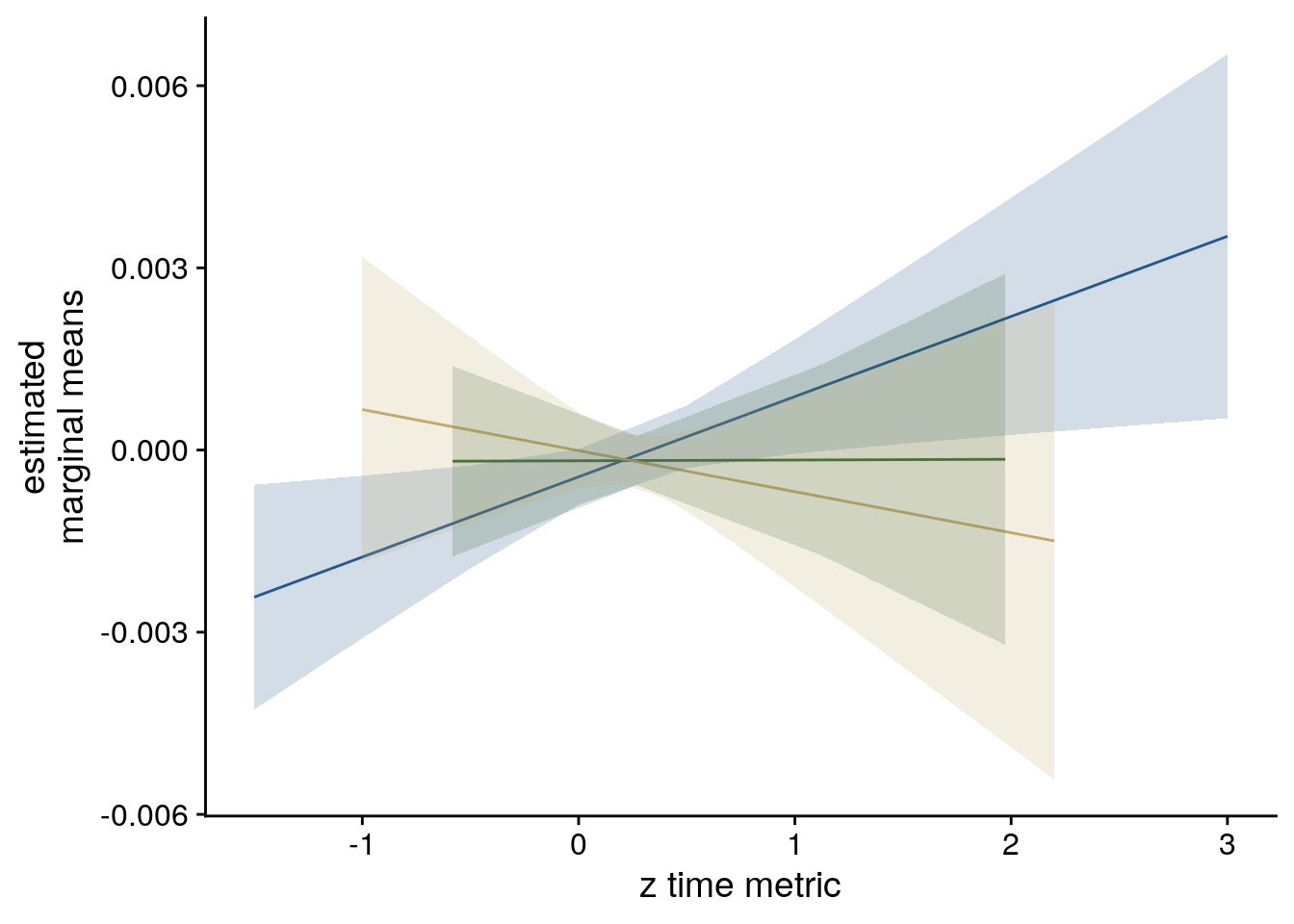#### LME model assumptions

lmm_diagplots_jb(lmm_full)### Illustration of Pattern Similarity Change

To illustrate the effect in a simpler way, we average pattern similarity changes based on a median split of events, separately for events from the same and from different sequences.

# get data from aHPC
rsa_dat_hpc <- rsa_dat %>%
filter(roi == "aHPC_lr")

# for each subject, ROI and same/diff day comparisons dichotomize virtual time difference
# based on a median split, then average high/low temporal distances
ps_change_means <-rsa_dat_hpc %>% group_by(sub_id, same_day) %>%
mutate(temp_dist = sjmisc::dicho(vir_time_diff, as.num = TRUE)+1) %>%
group_by(sub_id, same_day, temp_dist) %>%
summarise(ps_change = mean(ps_change), .groups = 'drop')

# make the high/low temporal distance variable a factor
ps_change_means <- ps_change_means %>%
mutate(
temp_dist = as.numeric(temp_dist),
temp_dist_f = factor(temp_dist),
temp_dist_f = plyr::mapvalues(temp_dist, from = c(1,2), to = c("low", "high")),
temp_dist_f = factor(temp_dist_f, levels= c("low", "high")),
same_day = as.factor(same_day),
same_day = plyr::mapvalues(same_day, from = c("FALSE", "TRUE"), to = c("different day", "same day")),
same_day = factor(same_day, levels = c("same day", "different day")))

# Test pattern similarity for same-sequence events separated by high vs. low temporal distances
set.seed(208) # set seed for reproducibility
stats_same_day_high_low <- paired_t_perm_jb(ps_change_means[ps_change_means$same_day=="same day" & ps_change_means$temp_dist_f == "high",]$ps_change - ps_change_means[ps_change_means$same_day=="same day" & ps_change_means$temp_dist_f == "low",]$ps_change)

# Cohen's d with Hedges' correction for paired samples using non-central t-distribution for CI
d<-cohen.d(d=ps_change_means[ps_change_means$same_day=="same day" & ps_change_means$temp_dist_f == "high",]$ps_change, f=ps_change_means[ps_change_means$same_day=="same day" & ps_change_means$temp_dist_f == "low",]$ps_change,
paired=TRUE, pooled=TRUE, hedges.correction=TRUE, noncentral=TRUE)
stats_same_day_high_low$d <- d$estimate
stats_same_day_high_low$dCI_low <- d$conf.int[]
stats_same_day_high_low$dCI_high <- d$conf.int[]
huxtable(stats_same_day_high_low) %>% theme_article()
Table 8.15:
estimatestatisticp.valuep_permparameterconf.lowconf.highmethodalternativeddCI_lowdCI_high
0.0012.480.01970.0201270.0001730.00183One Sample t-testtwo.sided0.6370.07520.871
# Test pattern similarity for different-sequence events separated by high vs. low temporal distances
set.seed(209) # set seed for reproducibility
stats_diff_day_high_low <- paired_t_perm_jb(ps_change_means[ps_change_means$same_day=="different day" & ps_change_means$temp_dist_f == "high",]$ps_change - ps_change_means[ps_change_means$same_day=="different day" & ps_change_means$temp_dist_f == "low",]$ps_change)

# Cohen's d with Hedges' correction for paired samples using non-central t-distribution for CI
d<-cohen.d(d=ps_change_means[ps_change_means$same_day=="different day" & ps_change_means$temp_dist_f == "high",]$ps_change, f=ps_change_means[ps_change_means$same_day=="different day" & ps_change_means$temp_dist_f == "low",]$ps_change,
paired=TRUE, pooled=TRUE, hedges.correction=TRUE, noncentral=TRUE)
stats_diff_day_high_low$d <- d$estimate
stats_diff_day_high_low$dCI_low <- d$conf.int[]
stats_diff_day_high_low$dCI_high <- d$conf.int[]
huxtable(stats_diff_day_high_low) %>% theme_article()
Table 8.15:
estimatestatisticp.valuep_permparameterconf.lowconf.highmethodalternativeddCI_lowdCI_high
-0.000711-3.260.003040.002527-0.00116-0.000263One Sample t-testtwo.sided-0.89-1.03-0.209

To visualize the effect, lets make a plot of the raw pattern similarity change for high vs. low temporal distances.

# extra data frame for annotations when facetting
anno <- data.frame(x1 = 1.3, x2 = 1.7,
y1 = c(0.00285, 0.0022), y2 = c(0.00285, 0.0022),
xstar = 1.5, ystar = c(0.003, 0.0023),
lab = c("*", "**"),
same_day = factor(c("same day", "different day"),levels = c("same day", "different day")))

# add a column with subject-specific jitter
# generate custom repeating jitter (multiply with -1/1 to shift towards each other)
ps_change_means <- ps_change_means %>%
mutate(
x_jit = as.numeric(temp_dist) + rep(jitter(rep(0,n_subs), amount = 0.05), each=4) * rep(c(-1,1),n_subs))

f5d<- ggplot(data=ps_change_means, aes(x=temp_dist, y=ps_change, fill = same_day, color = same_day)) +
gghalves::geom_half_violin(data = ps_change_means %>% filter(temp_dist_f == "low"),
aes(x=temp_dist, y=ps_change),
position=position_nudge(-0.1),
side = "l", color = NA) +
gghalves::geom_half_violin(data = ps_change_means %>% filter(temp_dist_f == "high"),
aes(x=temp_dist, y=ps_change),
position=position_nudge(0.1),
side = "r", color = NA) +
geom_boxplot(aes(group = temp_dist),width = .1, colour = "black", outlier.shape = NA) +
geom_line(aes(x = x_jit, group=sub_id,), color = ultimate_gray,
position = position_nudge(c(0.15, -0.15))) +
geom_point(aes(x=x_jit), position = position_nudge(c(0.15, -0.15)),
shape=16, size = 1) +
stat_summary(fun = mean, geom = "point", size = 1, shape = 16,
position = position_nudge(c(-0.1, 0.1)), colour = "black") +
stat_summary(fun.data = mean_se, geom = "errorbar",
position = position_nudge(c(-0.1, 0.1)), colour = "black", width = 0, size = 0.5) +
facet_wrap(~same_day, scales = "free") +
scale_fill_manual(values = unname(c(aHPC_colors["within_main"], aHPC_colors["across_main"]))) +
scale_color_manual(values = unname(c(aHPC_colors["within_main"], aHPC_colors["across_main"])), name = "sequence", labels=c("same", "different")) +
scale_x_continuous(breaks = c(1,2), labels=c("low", "high")) +
geom_segment(data = anno, aes(x = x1, xend = x2, y = y2, yend = y2), colour = "black", size = 0.5)+
geom_text(data = anno, aes(x = xstar,  y = ystar, label = lab), colour = "black") +
ylab('pattern similarity change') + xlab('temporal distance') +
guides(fill=FALSE, color=guide_legend(override.aes=list(fill=NA, alpha = 1, size=2), title.position = "top")) +
theme_cowplot() +
theme(text = element_text(size=10), axis.text = element_text(size=8),
legend.position = "right", strip.background = element_blank(), strip.text = element_blank())
f5dSummary Statistics: paired t-test for aHPC pattern similarity changes for high vs. low temporal distances for same sequence events t27=2.48, p=0.020, d=0.64, 95% CI [0.08, 0.87]

Summary Statistics: paired t-test for aHPC pattern similarity changes for high vs. low temporal distances for different sequence events t27=-3.26, p=0.002, d=-0.89, 95% CI [-1.03, -0.21]

### MDS

To explore how the event sequences could be arranged in a low-dimensional representational space, we want to run multi-dimensional scaling. To implement these analyses, we use the smacof-package, see also the helpful vignette here. MDS requires a distance matrix as an input. We will use a distance matrix based on the population-level predictions from the linear mixed model fitted to the anterior hippocampus pattern similarity change data as an input. This model captures the interaction between virtual temporal distances and sequence membership of event pairs on pattern similarity in the aHPC.

We first initialize a data frame that has one row for each possible pairwise comparison of two events and their (z-scored) absolute virtual temporal distances. We use this data frame as “new” data to predict pattern similarity changes from the linear mixed model. The resulting predicted pairwise similarity changes are converted to a matrix, converted to distances and scaled to the range from 0 to 1.

We aimed to explore how hippocampal event representations could be embedded in a low-dimensional space to give rise to the positive and negative correlations of pattern similarity change and temporal distances for same-sequence and different-sequence events, respectively. For each pair of events, we generated an expected representational similarity using the mixed model fitted to hippocampal pattern similarity that captures the interaction between virtual temporal distances and sequence membership (c.f. Figure 5 and Supplemental Table 6). We used the fixed effects parameters to generate model predictions. We chose this approach over the raw pattern similarity values to obtain less noisy estimates of the pairwise distances.

# check if virtual time exists
#(added for OSF code, which will not have this variable in the workspace yet because it did not run the design illustration script included in the full analysis)
if (!exists("virtual_time")){virtual_time <- beh_data %>% filter(sub_id=="31") %>% pull("virtual_time")} # virtual time is the same for all subjects

# create a tibble to use for MDS
# this tibble should have a row for each possible pairwise comparison of two events
mds_df <- expand_grid(virtual_time1=virtual_time, virtual_time2=virtual_time) %>%
day1 = rep(1:n_days, each=n_days*n_events_day^2),
day2 = rep(1:n_days, each=n_events_day, times=n_events_day*n_days),
event1 = rep(1:n_events_day, each=n_events_day*n_days, times=n_days),
event2 = rep(1:n_events_day, times=n_days^2*n_events_day),
idx1 = (day1-1)*5+event1, # for later conversion to matrix
ixd2 = (day2-1)*5+event2, # for later conversion to matrix
same_day = day1==day2,
same_day_dv = plyr::mapvalues(same_day, from = c(FALSE, TRUE), to = c(-1, 1)),
.before = virtual_time1) %>%
filter(!(day1==day2 & event1 == event2)) %>% # remove diagonal, important to do before z-scoring!
mutate(vir_time_diff = scale(abs(virtual_time1-virtual_time2))) # z-scored absolute virtual time difference

# for each event pair, get a predicted pattern similarity change value from the mixed model fitted to the aHPC data (ignoring the random effects)
mds_df$ps_change_hat <- predict(lmm_full, newdata=mds_df, re.form=NA) # turn into matrix (can probably be done in a prettier way without the loop) ps_change_hat_mat <- matrix(0, nrow=n_events_day*n_days, ncol=n_events_day*n_days) for (i in 1:nrow(mds_df)){ ps_change_hat_mat[mds_df$idx1[i], mds_df$ixd2[i]] <- mds_df$ps_change_hat[i]
}

# make plot of the resulting predicted pattern similarity change matrix
ps_change_hat_df <- reshape2::melt(ps_change_hat_mat)

f0a <- ggplot(ps_change_hat_df, aes(x=Var1, y=Var2, fill=value)) +
geom_tile() +
scale_y_reverse() +
scale_fill_scico(palette = "buda") +
ggtitle("predicted similarity change") +
xlab("events by day") +
ylab("events by day") +
labs(fill = "ps change") +
theme_cowplot() +
coord_fixed() #theme(aspect.ratio = 1)

# convert to distance matrix
ps_dist_hat_mat <- ps_change_hat_mat %>%
smacof::sim2diss(method = "corr")

# rescale from 0 to 1
ps_dist_hat_mat <- rescale(ps_dist_hat_mat, c(0,1))
diag(ps_dist_hat_mat)<-NA

# make plot of the resulting distance matrix
ps_dist_hat_df <- reshape2::melt(ps_dist_hat_mat)

f0b <- ggplot(ps_dist_hat_df, aes(x=Var1, y=Var2, fill=value)) +
geom_tile() +
scale_y_reverse() +
scale_fill_scico(palette = "buda", limits=c(0,1)) +
theme_cowplot() +
coord_fixed()+ #theme(aspect.ratio = 1) +
ggtitle("corresponding distance matrix") +
xlab("events by day") +
ylab("events by day") +
labs(fill = "distance")

f0a +f0b &
plot_annotation(tag_levels = 'A',
title = "MDS based on mixed model fitted to aHPC pattern similarity change") &
theme(text = element_text(size=10, family = font2use),
axis.text = element_text(size=8),
legend.text=element_text(size=8),
legend.title=element_text(size=8),
legend.position = 'right',
plot.tag = element_text(size = 10))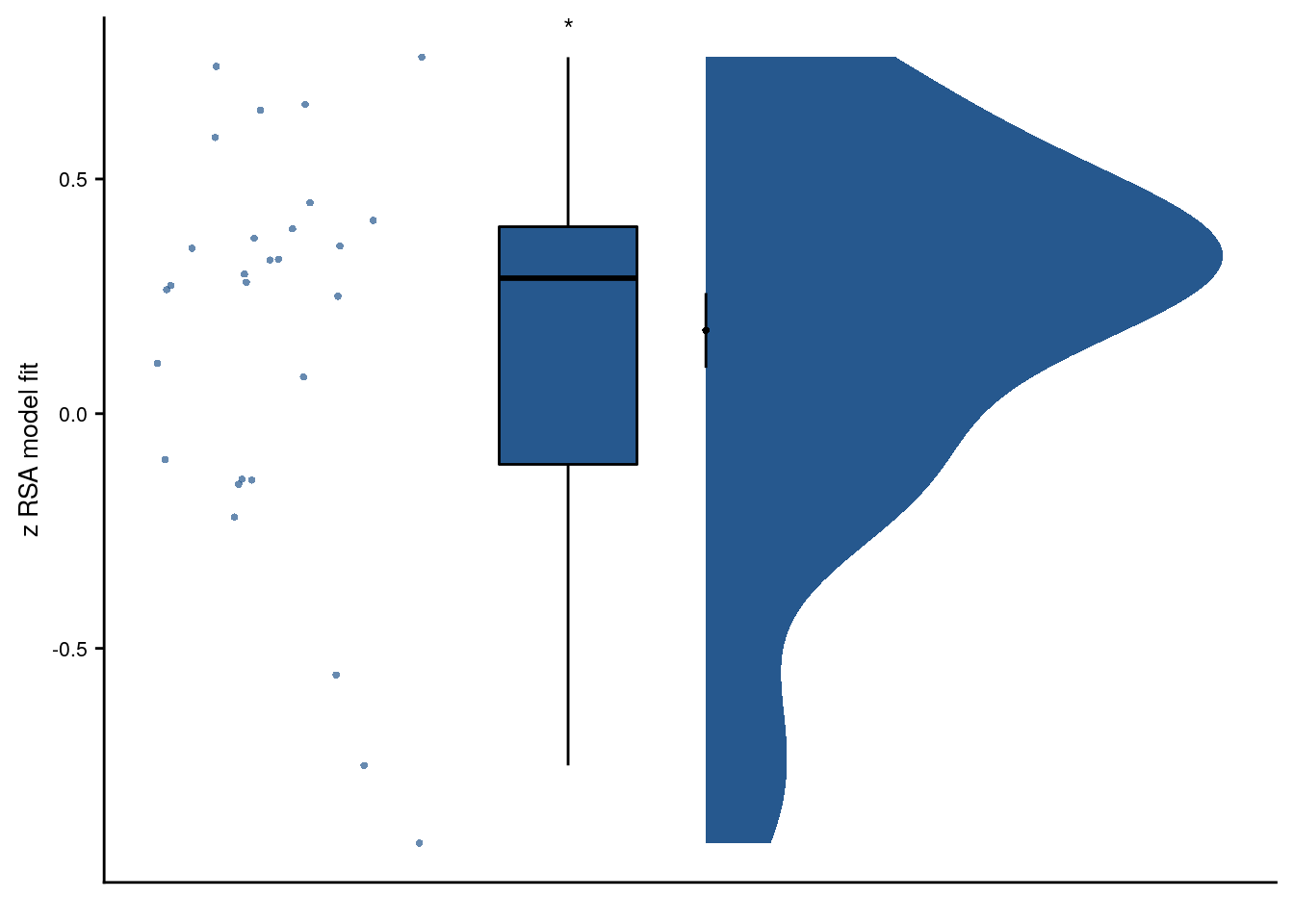# make plot of lower triangle only of the resulting distance matrix
lt <- upper.tri(ps_dist_hat_mat, diag = TRUE)
lt_df <- reshape2::melt(lt)
ps_dist_hat_df <- reshape2::melt(ps_dist_hat_mat)
ps_dist_hat_df<-ps_dist_hat_df[lt_df$value,] sfigc <- ggplot(ps_dist_hat_df, aes(x=Var1, y=Var2, fill=value)) + geom_tile() + scale_y_reverse() + scale_fill_scico(palette = "buda", limits=c(0,1), breaks=c(0,1)) + theme_cowplot() + coord_fixed() + theme(legend.position = c(1,1), legend.justification = c(1,1)) + xlab("events by sequence") + ylab("events by sequence") + guides(fill=guide_colorbar(title="distance", title.hjust = 0, barwidth = 0.5, barheight = 2)) sfigcWe are now ready to run MDS. We subject the distance matrix to MDS using an ordinal model (i.e. non-metric MDS) using two dimensions. Because MDS is sensitive to the starting configuration, we run MDS many times using random starting configurations. From the resulting configurations, we pick the one resulting in the lowest stress. This procedure is described in the vignette of the smacof-package, which we use for these analyses. Using the smacof-package115, the model-predicted similarities were converted to distances and the resulting distance matrix (Supplemental Figure 4C) was subjected to non-metric multidimensional scaling using two dimensions. Because MDS is sensitive to starting values, we ran multidimensional scaling 1000 times with random initial configurations and visualized the resulting configuration with the lowest stress value. # because MDS is sensitive to the start configuration, let's run it with many different random configurations (take the best in the end) n_rand_starts <- 1000 # maximum number of iterations max_it <- 5000 # run MDS for 2 dimensions n_rand_starts times with random start configuration and store best fit set.seed(106) # set seed for reproducibility stressvec <- rep(NA, n_rand_starts) fitbest <- mds(ps_dist_hat_mat, ndim = 2, itmax = max_it, init = "random", type = "ordinal") stressvec <- fitbest$stress

for(i in 2:n_rand_starts) {
fitran <- mds(ps_dist_hat_mat, ndim = 2, itmax = max_it, init = "random", type = "ordinal")
stressvec[i] <- fitran$stress if (fitran$stress < fitbest$stress){ fitbest <- fitran} } # get data frame of resulting configuration conf <- as.data.frame(fitbest$conf)
conf$day <- factor(rep(1:n_days, each = n_events_day)) conf$event <- factor(rep(1:n_events_day,n_days))
conf$virtual_time <- virtual_time # visualize as 2D scatter with lines of the configuration f5e <- ggplot(conf, aes(x=D1,y=D2, group=day, color=virtual_time, fill=virtual_time, shape=event)) + geom_path(aes(linetype=day),size=0.5, color="grey") + scale_linetype_manual(values=c("solid", "dashed", "dotted", "3111")) + geom_point(size=2) + scale_shape_manual(values = c(21:25)) + scico::scale_fill_scico(begin = 0.1, end = 0.7, palette = "devon", breaks=c(8,22)) + scico::scale_color_scico(begin = 0.1, end = 0.7, palette = "devon") + guides(linetype=guide_legend(title = "sequence", title.position="top", title.hjust = 0.5, order=1), shape=guide_legend(override.aes = list(shape = c(21:25), color = NA, fill = "grey"), title.position="top", title.hjust = 0.5, order=2), fill=guide_colorbar(title="virtual time", title.position="top", title.hjust = 1, barwidth = 0.5, barheight = 2), color=FALSE ) + xlab("dimension 1") + ylab("dimension 2") + theme_cowplot() + coord_fixed() + theme(#aspect.ratio = 1, legend.spacing.x = unit(1, 'mm'), legend.key.size = unit(2.5,"mm"), #legend.margin = margin(0,0,0,0.5, unit="cm"), legend.position = "right", legend.justification = "left") We are ready to create figure 5: # F5 layout = " AADDDD AADDDD AADDDD AADDDD BCEEEE BCEEEE BCEEEE" f5 <- f5a+f5b+f5c+f5d +f5e + plot_layout(design = layout, guides="keep") & theme(text = element_text(size=10, family = font2use), axis.text = element_text(size=8), legend.text=element_text(size=8), legend.title=element_text(size=8), #legend.position = 'bottom', #legend.box="vertical", legend.margin=margin(), #legend.spacing.x = unit(1, 'mm'), #legend.key.size = unit(3,"mm"), #legend.margin = margin(0,0,0,1, unit="cm"), plot.tag = element_text(size = 10)) & plot_annotation(tag_levels = 'A') # save and print (height was 18 when incl. searchlights) fn <- here("figures", "f5") ggsave(paste0(fn, ".pdf"), plot=f5, units = "cm", width = 17.4, height = 14, dpi = "retina", device = cairo_pdf) ggsave(paste0(fn, ".png"), plot=f5, units = "cm", width = 17.4, height = 14, dpi = "retina", device = "png") f5Figure 5. The anterior hippocampus generalizes temporal relations across sequences. A. Z-values show results of participant-specific linear models quantifying the effect of virtual time for event pairs from the same sequence (blue, as in Figure 4B) and from different sequences (red). Temporal distance is negatively related to hippocampal representational change for event pairs from different sequences. See Supplemental Figure 4 for mixed model analysis of across-sequence comparisons. The effect of virtual time differs for comparisons within the same sequence or between two different sequences. B. In the corresponding mixed effect model, a significant interaction between virtual time and sequence membership was observed. Dot plot shows fixed effect estimates with 95% confidence intervals. C. Estimated marginal means illustrate the diverging effects of virtual time as a function of sequence membership of event pairs. D. To visualize the interaction effect in A-C, raw pattern similarity change was averaged for events separated by low and high temporal distances, respectively. E. Multidimensional scaling results show low-dimensional embedding of the event sequences. Shapes indicate event order, color shows virtual times of events. The different lines connect the events belonging to the four sequences for illustration. *** p≤0.001; ** p<0.01; * p<0.05 ### UMAP To check if the MDS results depend on the specific algorithm, let’s also try the UMAP (Uniform Manifold Approximation and Projection for Dimension Reduction) algorithm on the mixed model distance matrix. We further used the UMAP-package116 to explore a low-dimensional embedding of the same distance matrix based on a different algorithm (Supplemental Figure 4D, n nearest neighbors=6; minimum distance=0.1). # set up umap config based on defaults, but using distance matrix as input and define the two parameters custom_config = umap.defaults custom_config$input = "dist"
custom_config$n_neighbors <- 6 # k nearest neighbors: high-values push towards global structure at cost of detail custom_config$min_dist <- 0.1 # minimum distance: high values lead to looser packing, but better topology

# run umap using the LME distance matrix as input. use the custom config
set.seed(306) # set seed for reproducibility
umap_dist = umap(ps_dist_hat_mat, config = custom_config)

# turn this solution into a tibble and include info about days and events
umap_df <- tibble(day = factor(rep(c(1:n_days), each=n_events_day)),
event = factor(rep(1:n_events_day,n_days)),
virtual_time <- virtual_time,
umapX = umap_dist$layout[,1], umapY = umap_dist$layout[,2])

# visualize as 2D scatter with lines of the configuration
sfigd <- ggplot(umap_df, aes(x=umapX,y=umapY, group=day, color=virtual_time, fill=virtual_time, shape=event)) +
geom_path(aes(linetype=day),size=0.5, color="grey") +
scale_linetype_manual(values=c("solid", "dashed", "dotted", "3111")) +
geom_point(size=2) +
scale_shape_manual(values = c(21:25)) +
scico::scale_fill_scico(begin = 0.1, end = 0.7, palette = "devon", breaks=c(8,22)) +
scico::scale_color_scico(begin = 0.1, end = 0.7, palette = "devon") +
guides(linetype=guide_legend(title = "sequence", title.position="top", title.hjust = 0.5, order=1),
shape=guide_legend(override.aes = list(shape = c(21:25), color = NA, fill = "grey"), title.position="top", title.hjust = 0.5, order=2),
fill=guide_colorbar(title="virtual time", title.position="top", title.hjust = 1, barwidth = 0.5, barheight = 2),
color=FALSE
) +
xlab("dimension 1") +
ylab("dimension 2") +
theme_cowplot() +
coord_fixed() +
theme(legend.spacing.x = unit(1, 'mm'),
legend.key.size = unit(2.5,"mm"),
legend.position = "right",
legend.justification = "left")

Let’s create supplemental figure 4

sfig <- sfiga + sfigb + sfigc + sfigd +
plot_layout(guides = "keep") +
plot_annotation(tag_levels = 'A') &
theme(text = element_text(size=10, family = font2use),
axis.text = element_text(size=8),
#legend.position = 'right',
plot.tag = element_text(size = 10))

fn <- here("figures", "sf04")
ggsave(paste0(fn, ".pdf"), plot=sfig, units = "cm",
width = 17.4, height = 11, dpi = "retina", device = cairo_pdf)
ggsave(paste0(fn, ".png"), plot=sfig, units = "cm",
width = 17.4, height = 11, dpi = "retina", device = "png")
sfig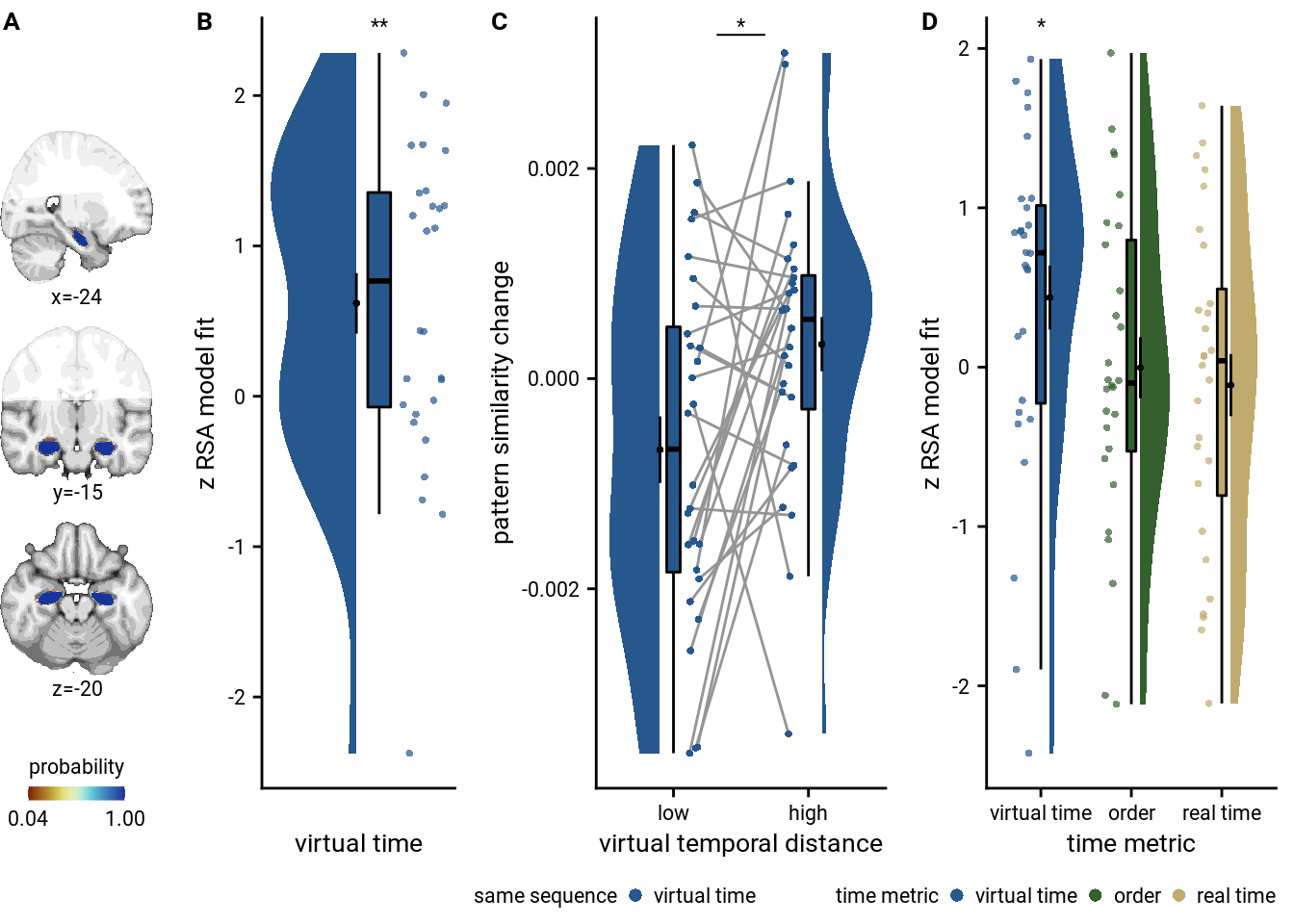Supplemental Figure 4. Virtual time predicts hippocampal pattern similarity change for events from different sequences. A, B. Fixed effect estimate with 95% confidence interval (A) and estimated marginal means (B) for the effect of virtual time on pattern similarity for events from different sequences are shown to illustrate the across-sequences generalization effect as observed in the linear mixed model analysis. This mixed model complements the summary statistics results shown in Figure 5A. * p<0.05. C. Scaled distance matrix showing distances between event pairs. This matrix was derived from the mixed model capturing the interaction effect of virtual temporal distances and sequence membership on hippocampal pattern similarity change and was used as input for multidimensional scaling and the UMAP algorithm (see Methods). D. Low-dimensional embedding of the twenty events as observed using UMAP. Shapes show the different events and color denotes virtual times of these events. The different lines connect the events belonging to the four sequences for illustration.

## 8.8 alEC

So far we focused on the anterior hippocampus. But what about our second region of interest, the anterior-lateral entorhinal cortex (alEC)?

### alEC: virtual time within/across sequences separately

First, we run the t-tests against zero for the alEC, separately for events from the same or two different sequences.

set.seed(108) # set seed for reproducibility

# run a group-level t-test on the RSA fits from the first level in alEC for within-day
stats <- rsa_fit %>%
filter(roi == "alEC_lr", same_day == TRUE) %>%
select(z_virtual_time) %>%
paired_t_perm_jb (., n_perm = n_perm)

# print results
huxtable(stats) %>% theme_article()
Table 8.16:
estimatestatisticp.valuep_permparameterconf.lowconf.highmethodalternative
-0.233-1.260.2170.21527-0.6110.145One Sample t-testtwo.sided
# re-run without outliers (see outliers in subsequent plot)
alEC_within <- rsa_fit %>%
filter(roi == "alEC_lr", same_day == TRUE)

# find outliers
is_outlier <- function(x) {
return(x < quantile(x, 0.25) - 1.5 * IQR(x) | x > quantile(x, 0.75) + 1.5 * IQR(x))
}
out_ind <- is_outlier(alEC_within$z_virtual_time) # run second-level analysis on data points that are not outliers set.seed(123) # set seed for reproducibility stats <- alEC_within[!out_ind,] %>% select(z_virtual_time) %>% paired_t_perm_jb (., n_perm = n_perm) # Cohen's d with Hedges' correction for one sample using non-central t-distribution for CI d<-cohen.d(d=alEC_within[!out_ind,]$z_virtual_time,
f=NA, paired=TRUE, hedges.correction=TRUE, noncentral=TRUE)
stats$d <- d$estimate
stats$dCI_low <- d$conf.int[]
stats$dCI_high <- d$conf.int[]

huxtable(stats) %>% theme_article()
Table 8.16:
estimatestatisticp.valuep_permparameterconf.lowconf.highmethodalternativeddCI_lowdCI_high
-0.482-3.540.001660.00224-0.763-0.201One Sample t-testtwo.sided-0.685-1.17-0.268

Summary Statistics: t-test against 0 for virtual time within sequence in alEC (outliers excluded)
t24=-3.54, p=0.002, d=-0.69, 95% CI [-1.17, -0.27]

# run a group-level t-test on the RSA fits from the first level in alEC for across-day
set.seed(145) # set seed for reproducibility
stats <- rsa_fit %>%
filter(roi == "alEC_lr", same_day == FALSE) %>%
select(z_virtual_time) %>%
paired_t_perm_jb (., n_perm = n_perm)

# Cohen's d with Hedges' correction for one sample using non-central t-distribution for CI
f=(rsa_fit %>% filter(roi == "alEC_lr", same_day == FALSE))$z_virtual_time, paired=TRUE, pooled=TRUE, hedges.correction=TRUE, noncentral=TRUE) stats$d <- d$estimate stats$dCI_low <- d$conf.int[] stats$dCI_high <- d$conf.int[] # print results huxtable(stats) %>% theme_article() Table 8.18: estimatestatisticp.valuep_permparameterconf.lowconf.highmethodalternativeddCI_lowdCI_high 0.01990.0730.9420.94227-0.5390.579One Sample t-testtwo.sided0.0213-0.3630.391 Summary Statistics: paired t-test comparing effect of virtual time within and across sequences in alEC t27=0.07, p=0.942, d=0.02, 95% CI [-0.36, 0.39] To finish this, we make a plot of the same and different sequence effect to go into the supplement. # generate custom repeating jitter (multiply with -1/1 to shift towards each other) plot_dat <- rsa_fit %>% filter(roi=="alEC_lr") %>% mutate( same_day_num = plyr::mapvalues(same_day, from = c("FALSE", "TRUE"),to = c(1,0)), same_day_char = plyr::mapvalues(same_day, from = c("FALSE", "TRUE"), to = c("different", "same")), same_day_char = fct_relevel(same_day_char, c("same", "different")), x_jit = as.numeric(same_day_char) + rep(jitter(rep(0,n_subs), amount=0.05), each=2) * rep(c(-1,1),n_subs)) sfig<- ggplot(data=plot_dat, aes(x=same_day_char, y=z_virtual_time, fill = same_day_char, color = same_day_char)) + geom_boxplot(aes(group=same_day_char), position = position_nudge(x = 0, y = 0), width = .1, colour = "black", outlier.shape = NA) + scale_fill_manual(values = unname(c(aHPC_colors["within_main"], aHPC_colors["across_main"]))) + scale_color_manual(values = unname(c(aHPC_colors["within_main"], aHPC_colors["across_main"])), name = "sequence", labels=c("same", "different")) + gghalves::geom_half_violin(data = plot_dat %>% filter(same_day == TRUE), aes(x=same_day_char, y=z_virtual_time), position=position_nudge(-0.1), side = "l", color = NA) + gghalves::geom_half_violin(data = plot_dat %>% filter(same_day == FALSE), aes(x=same_day_char, y=z_virtual_time), position=position_nudge(0.1), side = "r", color = NA) + stat_summary(fun = mean, geom = "point", size = 1, shape = 16, position = position_nudge(c(-0.1, 0.1)), colour = "black") + stat_summary(fun.data = mean_se, geom = "errorbar", position = position_nudge(c(-0.1, 0.1)), colour = "black", width = 0, size = 0.5) + geom_line(aes(x = x_jit, group=sub_id,), color = ultimate_gray, position = position_nudge(c(0.15, -0.15))) + geom_point(aes(x=x_jit, fill = same_day_char), position = position_nudge(c(-0.15, 0.15)), shape=16, size = 1) + ylab('z RSA model fit') + xlab('sequence') + guides(fill=FALSE, color=guide_legend(override.aes=list(fill=NA, alpha = 1, size=2))) + annotate(geom = "line",x=c(1.3, 1.7), y=2.2, size=0.5) + annotate(geom = "text", x = c(1, 1.5), y = 2.4, label = c("**","n.s."), hjust = 0.5, size = 8/.pt, family=font2use) + theme_cowplot() + theme(text = element_text(size=10), axis.text = element_text(size=8), legend.position = "none") # save and print to screen fn <- here("figures", "sf05") ggsave(paste0(fn, ".pdf"), plot=sfig, units = "cm", width = 6, height = 10, dpi = "retina", device = cairo_pdf) ggsave(paste0(fn, ".png"), plot=sfig, units = "cm", width = 6, height = 10, dpi = "retina", device = "png") sfig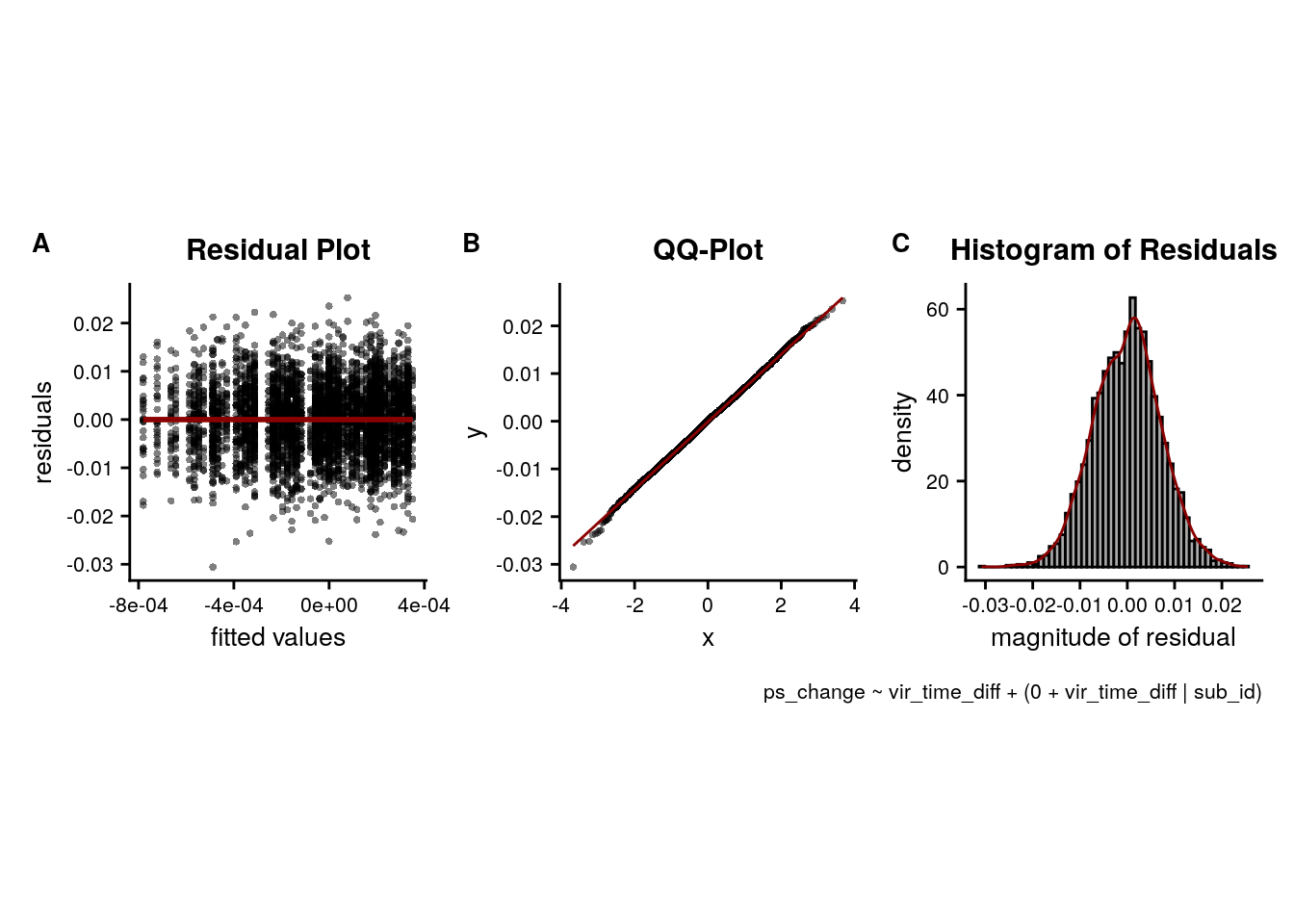Supplemental Figure 5. Relationship of pattern similarity change and temporal distances between events from the same and different sequences in the anterior-lateral entorhinal cortex. There was no statistically significant difference between correlations of virtual temporal distances and representational change in the anterior-lateral entorhinal cortex depending on whether event pairs were from the same or different sequences. Entorhinal representational change was negatively related to temporal distances between events from the same sequence (summary statistics: t24=-3.54, p=0.002; α=0.025, corrected for separate tests of events of the same and different sequences; three outliers excluded based on the boxplot criterion). The relationship between entorhinal pattern similarity change for events from different sequences was not statistically different from zero (summary statistics: t27=-1.60, p=0.122; α=0.025, corrected for separate tests of events of the same and different sequences). Circles show participant-specific Z-values from summary statistics approach; boxplot shows median and upper/lower quartile along with whiskers extending to most extreme data point within 1.5 interquartile ranges above/below the upper/lower quartile; black circle with error bars corresponds to mean±S.E.M.; distribution shows probability density function of data points. ** p<0.01 after outlier exclusion #### Mixed Effects Test the interaction between sequence membership and virtual time on alEC representational change in mixed model analysis. # get the data rsa_dat_alEC <- rsa_dat %>% filter(roi == "alEC_lr") # test the interaction for alEC # maximal random effect structure --> singular fit formula <- "ps_change ~ vir_time_diff * same_day_dv + (1 + vir_time_diff * same_day_dv | sub_id)" lmm_full = lme4::lmer(formula, data=rsa_dat_alEC, REML=FALSE) ## boundary (singular) fit: see ?isSingular # no correlations with random intercept --> singular fit formula <- "ps_change ~ vir_time_diff * same_day_dv + (1 + vir_time_diff * same_day_dv || sub_id)" lmm_full = lme4::lmer(formula, data=rsa_dat_alEC, REML=FALSE) ## boundary (singular) fit: see ?isSingular # random intercepts and random slopes only for the critical interaction --> singular fit formula <- "ps_change ~ vir_time_diff * same_day_dv + (1 + vir_time_diff : same_day_dv || sub_id)" lmm_full = lme4::lmer(formula, data=rsa_dat_alEC, REML=FALSE) ## boundary (singular) fit: see ?isSingular # random slopes for critical interactions set.seed(378) # set seed for reproducibility formula <- "ps_change ~ vir_time_diff * same_day_dv + (0 + vir_time_diff : same_day_dv | sub_id)" lmm_full = lme4::lmer(formula, data=rsa_dat_alEC, REML=FALSE) ## boundary (singular) fit: see ?isSingular summary(lmm_full, corr = FALSE) ## Linear mixed model fit by maximum likelihood ['lmerMod'] ## Formula: ps_change ~ vir_time_diff * same_day_dv + (0 + vir_time_diff:same_day_dv | sub_id) ## Data: rsa_dat_alEC ## ## AIC BIC logLik deviance df.resid ## -29766.7 -29727.3 14889.4 -29778.7 5314 ## ## Scaled residuals: ## Min 1Q Median 3Q Max ## -4.5825 -0.6461 0.0014 0.6380 4.1761 ## ## Random effects: ## Groups Name Variance Std.Dev. ## sub_id vir_time_diff:same_day_dv 0.000000 0.00000 ## Residual 0.000217 0.01473 ## Number of obs: 5320, groups: sub_id, 28 ## ## Fixed effects: ## Estimate Std. Error t value ## (Intercept) 0.0002612 0.0002521 1.036 ## vir_time_diff -0.0005716 0.0002549 -2.242 ## same_day_dv 0.0001285 0.0002521 0.510 ## vir_time_diff:same_day_dv -0.0002320 0.0002549 -0.910 ## convergence code: 0 ## boundary (singular) fit: see ?isSingular # tidy summary of the fixed effects that calculates 95% CIs lmm_full_bm <- broom.mixed::tidy(lmm_full, effects = "fixed", conf.int=TRUE, conf.method="profile") ## Computing profile confidence intervals ... # tidy summary of the random effects lmm_full_bm_re <- broom.mixed::tidy(lmm_full, effects = "ran_pars") # test against reduced model set.seed(370) # set seed for reproducibility lmm_reduced <- update(lmm_full, ~ . - vir_time_diff:same_day_dv) ## boundary (singular) fit: see ?isSingular lmm_aov <- anova(lmm_reduced, lmm_full) Mixed Model: Fixed effect of interaction of virtual time with sequence identity on alEC pattern similarity change $$\chi^2$$(1)=0.83, p=0.363 ### alEC: virtual time for all event pairs Because there is no significant difference when we look at event pairs from the same vs. different days in the above analysis, we collapse across all comparisons to test for an effect of virtual time on entorhinal pattern similarity change. #### Summary Statistics set.seed(110) # set seed for reproducibility # do RSA using linear model and calculate z-score for model fit from 1000 permutations rsa_fit_ec <- rsa_dat %>% filter(roi == "alEC_lr") %>% group_by(sub_id) %>% # run the linear model do(z = lm_perm_jb(in_dat = ., lm_formula = "ps_change ~ vir_time_diff", nsim = n_perm)) %>% mutate(z = list(setNames(z, c("z_intercept", "z_virtual_time")))) %>% unnest_wider(z) # run a group-level t-test on the RSA fits from the first level in alEC for both within-day and across days stats <- rsa_fit_ec %>% select(z_virtual_time) %>% paired_t_perm_jb (., n_perm = n_perm) # Cohen's d with Hedges' correction for one sample using non-central t-distribution for CI d<-cohen.d(d=rsa_fit_ec$z_virtual_time,
f=NA, paired=TRUE, hedges.correction=TRUE, noncentral=TRUE)
stats$d <- d$estimate
stats$dCI_low <- d$conf.int[]
stats$dCI_high <- d$conf.int[]

# print results
huxtable(stats) %>% theme_article()
Table 8.19:
estimatestatisticp.valuep_permparameterconf.lowconf.highmethodalternativeddCI_lowdCI_high
-0.335-2.310.0290.029427-0.634-0.0371One Sample t-testtwo.sided-0.423-0.835-0.0451

Summary Statistics: t-test against 0 for virtual time for all pairs in alEC
t27=-2.31, p=0.029, d=-0.42, 95% CI [-0.84, -0.05]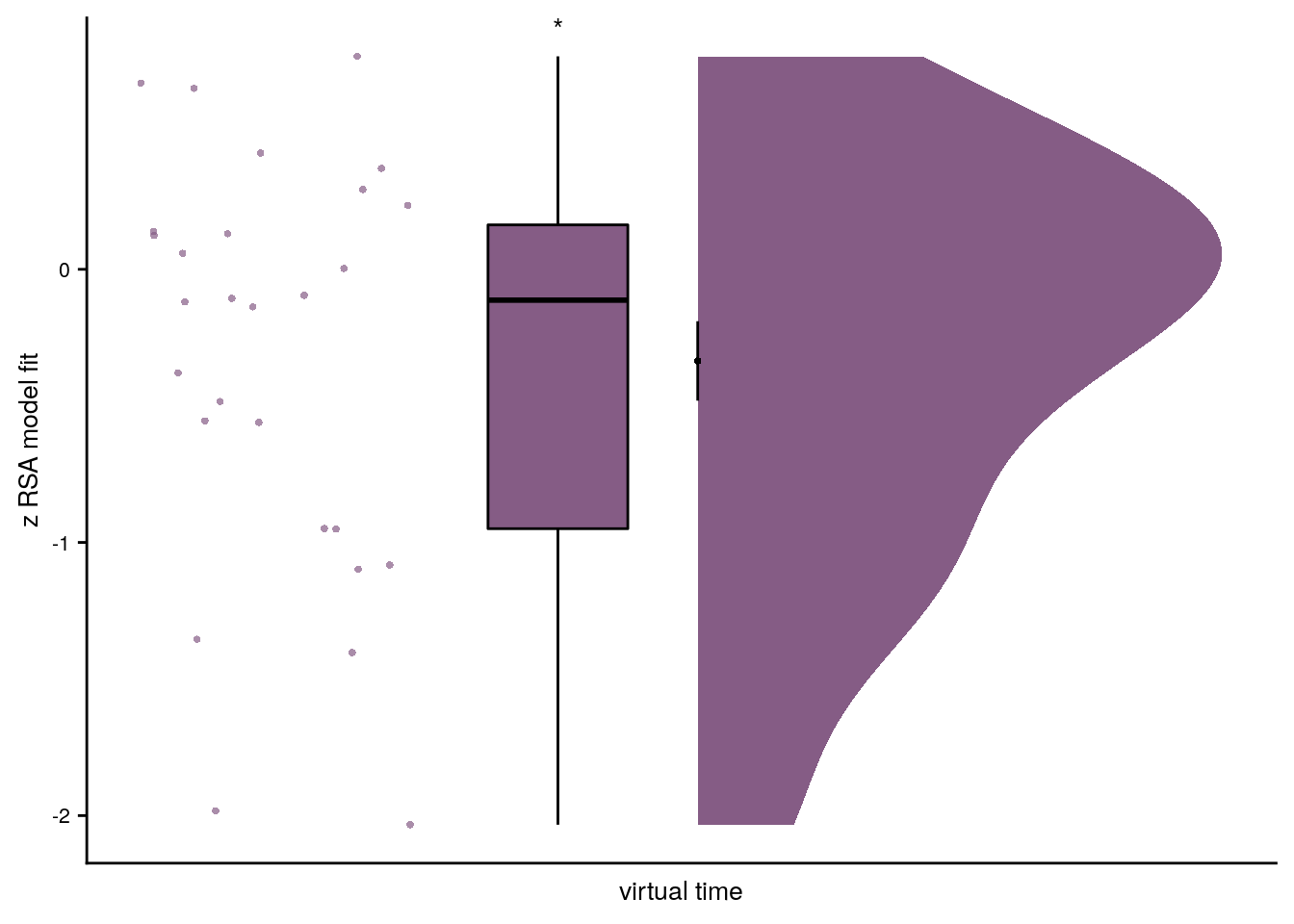#### Linear Mixed Effects

When fitting a linear mixed effects model to test the effect of virtual time on pattern similarity change in the alEC, we thus use all pairwise comparisons. Due to singular fits we reduce the random effects structure to only include random slopes for virtual time. The model is still singular, but we keep these random slopes in the model to avoid anti-conservativity and to account for the within-subject dependencies.

# extract the comparisons from the same day
rsa_dat_ec <- rsa_dat %>% filter(roi == "alEC_lr")

# define the full model with virtual time difference as
# fixed effect and random intercepts for subject --> singular fit
formula <- "ps_change ~ vir_time_diff + (1 + vir_time_diff | sub_id)"
lmm_full <- lme4::lmer(formula, data = rsa_dat_ec, REML = FALSE)
## boundary (singular) fit: see ?isSingular
# remove the correlation between random slopes and intercepts --> still singular
formula <- "ps_change ~ vir_time_diff + (1 + vir_time_diff || sub_id)"
lmm_full <- lme4::lmer(formula, data = rsa_dat_ec, REML = FALSE)
## boundary (singular) fit: see ?isSingular
# keep only random slopes --> still singular
set.seed(301) # set seed for reproducibility
formula <- "ps_change ~ vir_time_diff + (0 + vir_time_diff | sub_id)"
lmm_full <- lme4::lmer(formula, data = rsa_dat_ec, REML = FALSE)
## boundary (singular) fit: see ?isSingular
#lmm_full = lme4::lmer(formula, data=rsa_dat_mtl, REML=FALSE,
#                control = lmerControl(optimizer ="bobyqa", optCtrl=list(maxfun=100000)))
#lmm_full = lmer(formula, data=rsa_dat_mtl, REML=FALSE,
#                 control = lmerControl(optimizer ='nloptwrap',
#lmm_full = lme4::lmer(formula, data=rsa_dat_mtl, REML=FALSE,
#                control = lmerControl(optimizer ='optimx', optCtrl=list(method='nlminb')))

summary(lmm_full, corr=FALSE)
## Linear mixed model fit by maximum likelihood  ['lmerMod']
## Formula: ps_change ~ vir_time_diff + (0 + vir_time_diff | sub_id)
##    Data: rsa_dat_ec
##
##      AIC      BIC   logLik deviance df.resid
## -29769.8 -29743.5  14888.9 -29777.8     5316
##
## Scaled residuals:
##     Min      1Q  Median      3Q     Max
## -4.5888 -0.6468  0.0016  0.6373  4.1684
##
## Random effects:
##  Groups   Name          Variance  Std.Dev.
##  sub_id   vir_time_diff 0.0000000 0.00000
##  Residual               0.0002171 0.01473
## Number of obs: 5320, groups:  sub_id, 28
##
## Fixed effects:
##                 Estimate Std. Error t value
## (Intercept)    0.0001670  0.0002020   0.827
## vir_time_diff -0.0004237  0.0002023  -2.095
## convergence code: 0
## boundary (singular) fit: see ?isSingular
# tidy summary of the fixed effects that calculates 95% CIs
lmm_full_bm <- broom.mixed::tidy(lmm_full, effects = "fixed", conf.int=TRUE, conf.method="profile")
## Computing profile confidence intervals ...
# tidy summary of the random effects
lmm_full_bm_re <- broom.mixed::tidy(lmm_full, effects = "ran_pars")

# test against reduced model
set.seed(317) # set seed for reproducibility
formula <- "ps_change ~ 1 + (0 + vir_time_diff | sub_id)"
lmm_no_vir_time <- lme4::lmer(formula, data = rsa_dat_ec, REML = FALSE)
## boundary (singular) fit: see ?isSingular
lmm_aov <- anova(lmm_no_vir_time, lmm_full)

Mixed Model: Fixed effect of virtual time on alEC pattern similarity change (all pairs) $$\chi^2$$(1)=4.39, p=0.036

Make mixed model summary table that includes overview of fixed and random effects as well as the model comparison to the nested (reduced) model.

fe_names <- c("intercept", "virtual time")
re_groups <- c(rep("participant",1), "residual")
re_names <- c("virtual time (SD)", "SD")

lmm_hux <- make_lme_huxtable(fix_df=lmm_full_bm,
ran_df = lmm_full_bm_re,
aov_mdl = lmm_aov,
fe_terms =fe_names,
re_terms = re_names,
re_groups = re_groups,
lme_form = gsub(" ", "", paste0(deparse(formula(lmm_full)),
collapse = "", sep="")),
caption = "Mixed Model: Virtual time explains representational change in the anterior-lateral entorhinal cortex (all events)")

# convert the huxtable to a flextable for word export
stable_lme_alEC_virtime_all_events <- convert_huxtable_to_flextable(ht = lmm_hux)

# print to screen
theme_article(lmm_hux)
fixed effects term estimate SE t-value 0.000167 0.000202 0.83 -0.000229 0.000563 -0.000424 0.000202 -2.09 -0.000820 -0.000027 0.000000 0.014734 -29767.39 14886.69 -29769.77 14888.89 4.39 1 0.036

Visualize the effects.

lmm_full_bm <- lmm_full_bm %>%
mutate(term=as.factor(term))

# dot plot of Fixed Effect Coefficients with CIs
f6c <- ggplot(data = lmm_full_bm[2,], aes(x = term, color = term)) +
geom_hline(yintercept = 0, colour="black", linetype="dotted") +
geom_errorbar(aes(ymin = conf.low, ymax = conf.high, width = NA), size = 0.5) +
geom_point(aes(y = estimate), shape = 16, size = 1) +
scale_fill_manual(values = unname(alEC_colors["main"])) +
scale_color_manual(values = unname(alEC_colors["main"]), labels = c("Virtual Time")) +
labs(x = "virtual time", y="fixed effect\ncoefficient", color = "Time Metric") +
guides(color = FALSE) +
annotate(geom = "text", x = 1, y = 0.00005, label = "*", hjust = 0.5, size = 8/.pt, family=font2use) +
theme_cowplot() +
theme(plot.title = element_text(hjust = 0.5), axis.text.x=element_blank())
f6c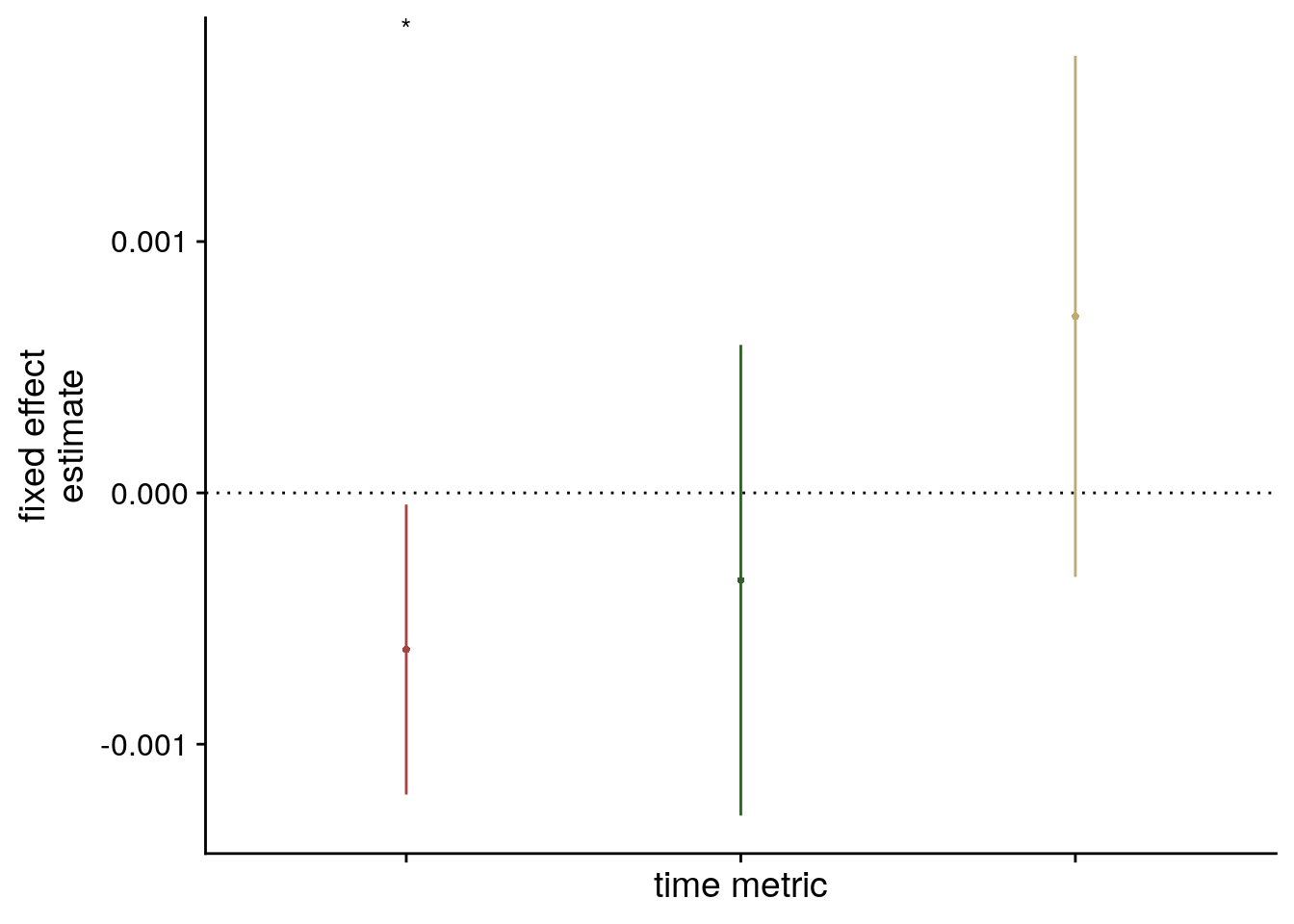# estimate marginal means for each model term by omitting the terms argument
lmm_full_emm <- ggeffects::ggpredict(lmm_full, ci.lvl = 0.95) %>% get_complete_df()

# plot marginal means
f6d <- ggplot(data = lmm_full_emm, aes(color = group)) +
geom_line(aes(x, predicted)) +
geom_ribbon(aes(x, ymin = conf.low, ymax = conf.high, fill = group), alpha = .2, linetype=0) +
scale_color_manual(values = unname(alEC_colors["main"])) +
scale_fill_manual(values = unname(alEC_colors["main"])) +
ylab('estimated\nmarginal means') +
xlab('z virtual time') +
guides(color = FALSE, fill =FALSE) +
theme_cowplot()
f6d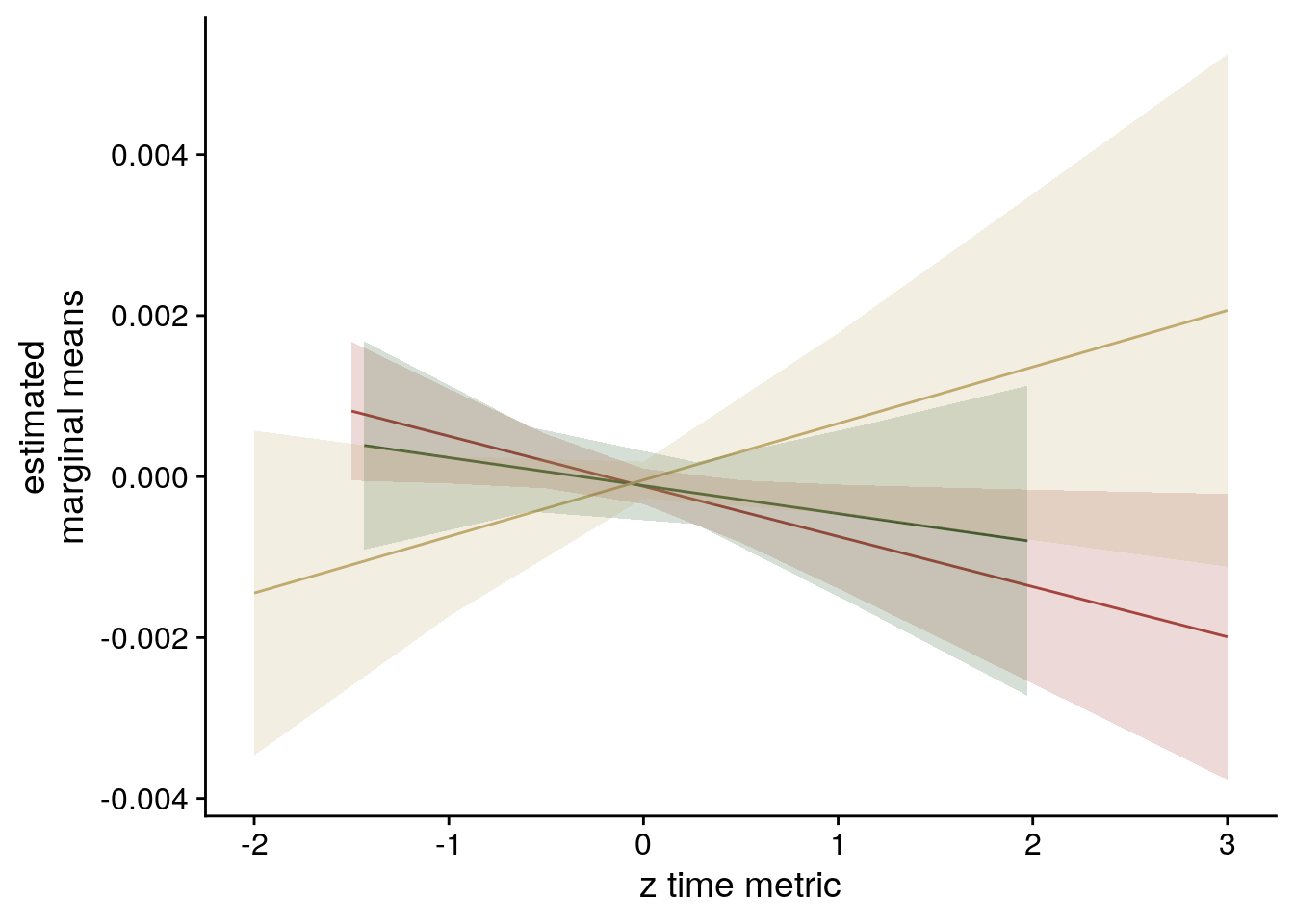#### Pattern Similarity Change Visualization

To further visualize the effect, lets make a plot of the raw pattern similarity change for high vs. low temporal distances, averaged across all pairs.

# get data from alEC
rsa_dat_ec <- rsa_dat %>%
filter(roi == "alEC_lr")

# for each subject dichotomize virtual time difference
# based on a median split, then average high/low temporal distances
ps_change_means <-rsa_dat_ec %>% group_by(sub_id) %>%
mutate(temp_dist = sjmisc::dicho(vir_time_diff, as.num = TRUE)+1) %>%
group_by(sub_id, temp_dist) %>%
summarise(ps_change = mean(ps_change), .groups = 'drop')

# make the high/low temporal distance variable a factor
ps_change_means <- ps_change_means %>%
mutate(
temp_dist = as.numeric(temp_dist),
temp_dist_f = factor(temp_dist),
temp_dist_f = plyr::mapvalues(temp_dist, from = c(1,2), to = c("low", "high")),
temp_dist_f = factor(temp_dist_f, levels= c("low", "high")),
roi = "alEC")

# add a column with subject-specific jitter
# generate custom repeating jitter (multiply with -1/1 to shift towards each other)
ps_change_means <- ps_change_means %>%
mutate(
x_jit = as.numeric(temp_dist) + rep(jitter(rep(0,n_subs), amount = 0.05), each=2) * rep(c(-1,1),n_subs))

f6e<- ggplot(data=ps_change_means, aes(x=temp_dist, y=ps_change, fill = roi, color = roi)) +
gghalves::geom_half_violin(data = ps_change_means %>% filter(temp_dist_f == "low"),
aes(x=temp_dist, y=ps_change),
position=position_nudge(-0.1),
side = "l", color = NA) +
gghalves::geom_half_violin(data = ps_change_means %>% filter(temp_dist_f == "high"),
aes(x=temp_dist, y=ps_change),
position=position_nudge(0.1),
side = "r", color = NA) +
geom_boxplot(aes(group = temp_dist),width = .1, colour = "black", outlier.shape = NA) +
geom_line(aes(x = x_jit, group=sub_id,), color = ultimate_gray,
position = position_nudge(c(0.15, -0.15))) +
geom_point(aes(x=x_jit), position = position_nudge(c(0.15, -0.15)),
shape=16, size = 1) +
stat_summary(fun = mean, geom = "point", size = 1, shape = 16,
position = position_nudge(c(-0.1, 0.1)), colour = "black") +
stat_summary(fun.data = mean_se, geom = "errorbar",
position = position_nudge(c(-0.1, 0.1)), colour = "black", width = 0, size = 0.5) +
scale_fill_manual(values = unname(alEC_colors["main"])) +
scale_color_manual(values = unname(alEC_colors["main"]))+
scale_x_continuous(breaks = c(1,2), labels=c("low", "high")) +
ylab('pattern similarity change') + xlab('temporal distance') +
guides(fill = FALSE, color = FALSE) +
theme_cowplot() +
theme(text = element_text(size=10), axis.text = element_text(size=8),
legend.position = "none", strip.background = element_blank(), strip.text = element_blank())
f6eNow we are ready to make figure 6 of the manuscript.

layoutBE = "
BBCEEE
BBDEEE"

layoutABE ="
ABBBBBB
ABBBBBB"

f6 <- f6a+
{f6b+f6c+f6d+f6e+
plot_layout(design = layoutBE, guides = "collect") &
theme(text = element_text(size=10, family = font2use),
axis.text = element_text(size=8),
legend.text=element_text(size=8),
legend.title=element_text(size=8),
legend.spacing.x = unit(1, 'mm'),
legend.key.size = unit(3,"mm"),
legend.position = 'bottom')} +
plot_layout(design = layoutABE, guides = "keep") &
plot_annotation(theme = theme(plot.margin = margin(t=0, r=-5, b=0, l=-19, unit="pt")),
tag_levels = 'A') &
theme(plot.tag = element_text(size = 10, face="bold"),
#plot.tag.position = "topleft",
#plot.margin=grid::unit(c(5,8,5,0), "pt"),
text = element_text(size=10, family=font2use))
f6[] <- f6[] +
theme(legend.position = c(0.5,-0.13),
legend.text=element_text(size=8),
legend.title=element_text(size=8),
plot.tag = element_text(margin = margin(l = 13)))

# save and print
fn <- here("figures", "f6")
ggsave(paste0(fn, ".pdf"), plot=f6, units = "cm",
width = 17.4, height = 10, dpi = "retina", device = cairo_pdf)
ggsave(paste0(fn, ".png"), plot=f6, units = "cm",
width = 17.4, height = 10, dpi = "retina", device = "png")
f6Figure 6. The anterior-lateral entorhinal cortex uses a shared representational format for relations of events from the same and different sequences. A. The anterior-lateral entorhinal cortex region of interest is displayed on the MNI template with voxels outside the field of view shown in lighter shades of gray. Color code denotes probability of a voxel to be included based on participant-specific masks (see Methods). B. Z-values for participant-specific RSA model fits show a negative relationship between pattern similarity change and virtual temporal distances when collapsing across all event pairs. C,D. Parameter estimate with 95% confidence intervals (C) and estimated marginal means (D) show the fixed effect of virtual time from the corresponding linear mixed effect model. E. To illustrate the effect in B-D, raw pattern similarity change in the anterior-lateral entorhinal cortex was averaged for events separated by low and high temporal distances. * p<0.05

## 8.9 aHPC vs. alEC

The findings above suggest differences between how sequence memories in aHPC and alEC are shaped by virtual time depending on whether events from the same or from different sequences are compared. Let’s qualify these differences by running models with both ROIs.

### Summary Statistics

Permutation-based repeated measures ANOVAs were carried out using the permuco package111 and we report generalized η2 as effect sizes computed using the afex-package112.

In the summary statistics approach, this comes down to a 2x2 repeated measures ANOVA with the factors ROI (aHPC vs. alEC) and sequence (same vs. different). We use a permutation-based repeated measures ANOVA to show there is a significant interaction between the two factors.

# sanity check & effect size: parametric ANOVA using
# run a 2x2 repeated measures ANOVA from afex package
aov_fit <- afex::aov_ez(id = "sub_id",
dv = "z_virtual_time",
data = rsa_fit,
within = c("roi", "same_day"), print.formula = TRUE, es="ges")
## Formula send to aov_car: z_virtual_time ~ 1 + Error(sub_id/(roi * same_day))
# print stats
aov_fit
## Anova Table (Type 3 tests)
##
## Response: z_virtual_time
##         Effect    df  MSE       F  ges p.value
## 1          roi 1, 27 0.82  3.10 + .024    .089
## 2     same_day 1, 27 1.22  7.41 * .082    .011
## 3 roi:same_day 1, 27 1.09 7.76 ** .076    .010
## ---
## Signif. codes:  0 '***' 0.001 '**' 0.01 '*' 0.05 '+' 0.1 ' ' 1
set.seed(111) # set seed for reproducibility
# permutation repeated measures from permuco package (use factorial same_day variable)
aov_fit_perm <- permuco::aovperm(formula = z_virtual_time ~ roi * same_day_char + Error(sub_id/(roi*same_day_char)),
data = rsa_fit, method = "Rd_kheradPajouh_renaud", np =n_perm)
summary(aov_fit_perm)
Table 8.21:
SSndfnSSddfdMSEnMSEdFparametric P(>F)permutation P(>F)
2.55122.2272.550.8223.1 0.0895 0.0859
9.06133  279.061.22 7.410.0112 0.0115
8.43129.3278.431.09 7.760.009640.0102

Summary Statistics: permutation-based repeated measures ANOVA (Rd_kheradPajouh_renaud method) main effect of ROI: F1,27=3.1, p=0.086, $$\eta^2$$=0.02
main effect of sequence: F1,27=7.41, p=0.012, $$\eta^2$$=0.08
interaction ROI and sequence: F1,27=7.76, p=0.010, $$\eta^2$$=0.08

### Linear Mixed Effects

To test whether the hippocampus and entorhinal cortex differentially represent virtual time between events within a virtual day and also virtual days, we also run a linear mixed model on pattern similarity change in both regions that includes event pairs that are from the same day, but also from different days. We include the fixed effects ROI (aHPC and alEC), day (same or different) and virtual time difference as well as their interaction in the model.

# get data from aHPC and alEC for within and across day comparisons
rsa_dat_mtl <- rsa_dat %>% filter(roi == "aHPC_lr" | roi == "alEC_lr")

# recode the ROI factor to -1 and 1
rsa_dat_mtl <- rsa_dat_mtl %>%
mutate(
roi_dv = plyr::mapvalues(roi, from = c("alEC_lr", "aHPC_lr"), to = c(-1, 1)))

# run LME for effect of time with factors same/different day vs. ROI
# random intercepts and all random slopes and interactions --> singular fit
formula <- "ps_change ~ vir_time_diff * same_day_dv * roi_dv + (1 + vir_time_diff * same_day_dv * roi_dv | sub_id)"
lmm_full = lme4::lmer(formula, data=rsa_dat_mtl, REML=FALSE,
control = lmerControl(optimizer ="bobyqa", optCtrl=list(maxfun=40000)))
## boundary (singular) fit: see ?isSingular
# remove correlation between random slopes and random intercepts --> singular fit
formula <- "ps_change ~ vir_time_diff * same_day_dv * roi_dv + (1 + vir_time_diff * same_day_dv * roi_dv || sub_id)"
lmm_full = lme4::lmer(formula, data=rsa_dat_mtl, REML=FALSE,
control = lmerControl(optimizer ="bobyqa", optCtrl=list(maxfun=40000)))
## boundary (singular) fit: see ?isSingular
# keep only highest-order interaction as random slopes
formula <- "ps_change ~ vir_time_diff * same_day_dv * roi_dv + (1 + vir_time_diff : same_day_dv : roi_dv | sub_id)"
lmm_full = lme4::lmer(formula, data=rsa_dat_mtl, REML=FALSE,
control = lmerControl(optimizer ="bobyqa", optCtrl=list(maxfun=40000)))
## boundary (singular) fit: see ?isSingular
#lmm_full = lme4::lmer(formula, data=rsa_dat_mtl, REML=FALSE,
#                control = lmerControl(optimizer ='optimx', optCtrl=list(method='nlminb', maxit=100000)))
set.seed(398) # set seed for reproducibility
lmm_full = lme4::lmer(formula, data=rsa_dat_mtl, REML=FALSE,
control = lmerControl(optimizer ='nloptwrap',
optCtrl=list(method='NLOPT_LN_NELDERMEAD', maxfun=100000)))
## boundary (singular) fit: see ?isSingular
summary(lmm_full, corr = FALSE)
## Linear mixed model fit by maximum likelihood  ['lmerMod']
## Formula: ps_change ~ vir_time_diff * same_day_dv * roi_dv + (1 + vir_time_diff:same_day_dv:roi_dv |      sub_id)
##    Data: rsa_dat_mtl
## Control: lmerControl(optimizer = "nloptwrap", optCtrl = list(method = "NLOPT_LN_NELDERMEAD",      maxfun = 1e+05))
##
##      AIC      BIC   logLik deviance df.resid
## -64704.2 -64595.1  32367.1 -64734.2    10625
##
## Scaled residuals:
##     Min      1Q  Median      3Q     Max
## -5.9391 -0.5647  0.0096  0.5629  5.3781
##
## Random effects:
##  Groups   Name                               Variance  Std.Dev.  Corr
##  sub_id   (Intercept)                        2.460e-07 0.0004960
##           vir_time_diff:same_day_dv:roi_dv1  2.897e-08 0.0001702 -1.00
##           vir_time_diff:same_day_dv:roi_dv-1 1.772e-07 0.0004209 -0.15  0.15
##  Residual                                    1.332e-04 0.0115397
## Number of obs: 10640, groups:  sub_id, 28
##
## Fixed effects:
##                                      Estimate Std. Error t value
## (Intercept)                        -0.0001934  0.0002186  -0.885
## vir_time_diff                       0.0002384  0.0001997   1.194
## same_day_dv                        -0.0001327  0.0001974  -0.672
## roi_dv-1                            0.0004546  0.0002792   1.628
## vir_time_diff:same_day_dv           0.0005129  0.0002022   2.536
## vir_time_diff:roi_dv-1             -0.0008100  0.0002824  -2.868
## same_day_dv:roi_dv-1                0.0002612  0.0002792   0.936
## vir_time_diff:same_day_dv:roi_dv-1 -0.0007449  0.0002938  -2.535
## convergence code: 0
## boundary (singular) fit: see ?isSingular
# tidy summary of the fixed effects that calculates 95% CIs
lmm_full_bm <- broom.mixed::tidy(lmm_full, effects = "fixed",
conf.int=TRUE, optimizer = 'bobyqa')

# tidy summary of the random effects
lmm_full_bm_re <- broom.mixed::tidy(lmm_full, effects = "ran_pars")

# compare to reduced model without interaction term
set.seed(351) # set seed for reproducibility
lmm_reduced <-update(lmm_full, ~ . - vir_time_diff:same_day_dv:roi_dv)
## boundary (singular) fit: see ?isSingular
lmm_aov <- anova(lmm_reduced, lmm_full)
lmm_aov
Table 8.22:
nparAICBIClogLikdevianceChisqDfPr(>Chisq)
14-6.47e+04-6.46e+043.24e+04-6.47e+04
15-6.47e+04-6.46e+043.24e+04-6.47e+046.3110.012

Mixed Model: Fixed effect of interaction virtual time with sequence identity and ROI on pattern similarity change $$\chi^2$$(1)=6.31, p=0.012

Make mixed model summary table that includes overview of fixed and random effects as well as the model comparison to the nested (reduced) model.

fe_names <- c("intercept", "virtual time", "day", "ROI", "virtual time * day",
"virtual time * ROI", "day * ROI", "virtual time * day * ROI")
re_groups <- c(rep("participant",6), "residual")
re_names <- c("intercept (SD)", "corr. intercept, virtual time:day:ROI1",
"corr. intercept, virtual time:day:ROI-1", "virtual time:day:ROI1 (SD)",
"corr. virtual time:day:ROI1, virtual time:day:ROI-1",
"virtual time:day:ROI-1 (SD)", "SD")

lmm_hux <- make_lme_huxtable(fix_df=lmm_full_bm,
ran_df = lmm_full_bm_re,
aov_mdl = lmm_aov,
fe_terms =fe_names,
re_terms = re_names,
re_groups = re_groups,
lme_form = gsub(" ", "", paste0(deparse(formula(lmm_full)),
collapse = "", sep="")),
caption = "Mixed Model: The effect of virtual time differentially depends on sequence membership in the anterior hippocampus and the anterior-lateral entorhinal cortex")

# convert the huxtable to a flextable for word export
stable_lme_virtime_aHPC_vs_alEC <- convert_huxtable_to_flextable(ht = lmm_hux)

# print to screen
theme_article(lmm_hux)
fixed effects term estimate SE t-value -0.000193 0.000219 -0.89 -0.000622 0.000235 0.000238 0.000200 1.19 -0.000153 0.000630 -0.000133 0.000197 -0.67 -0.000520 0.000254 0.000455 0.000279 1.63 -0.000093 0.001002 0.000513 0.000202 2.54 0.000117 0.000909 -0.000810 0.000282 -2.87 -0.001363 -0.000257 0.000261 0.000279 0.94 -0.000286 0.000808 -0.000745 0.000294 -2.54 -0.001321 -0.000169 0.000496 -1.000000 -0.151340 0.000170 0.151340 0.000421 0.011540 -64699.87 32363.94 -64704.19 32367.09 6.31 1 0.012

## 8.10 Searchlight Results

We further probed how temporal distances between events shaped representational change using searchlight analyses. Using the procedures described above, we calculated pattern similarity change values for search spheres with a radius of 3 voxels around the center voxel. Search spheres were centered on all brain voxels within our field of view. Within a given search sphere, only gray matter voxels were analyzed. Search spheres not containing more than 25 gray matter voxels were discarded. For each search sphere, we implemented linear models to quantify the relationship between representational change and the learned temporal structure. Specifically, we assessed the relationship of pattern similarity change and absolute virtual temporal distances, separately for event pairs from the same sequences and from pairs from different sequences. In a third model, we included all event pairs and tested for an interaction effect of a sequence (same or different) predictor and virtual temporal distances. The t-values of the respective regressors of interest were stored at the center voxel of a given search sphere. The resulting t-maps were registered to MNI space for group level statistics and spatially smoothed (FWHM 3mm). Group level statistics were carried out using random sign flipping implemented with FSL Randomise and threshold-free cluster enhancement. We corrected for multiple comparisons using a small volume correction mask including our a priori regions of interest, the anterior hippocampus and the anterior-lateral entorhinal cortex.

To complement our ROI-analyses we also ran searchlights analyses. Here, we used search spheres with a 3 voxel radius around the center voxel. Each brain voxel served as a sphere center, but only gray matter voxels were analyzed as features. We analyzed the relationship of virtual temporal distances to pattern similarity changes for events from the same day only, from different days only, and for the interaction of virtual time with the same/different day factor.

First, we load the MNI template brain (1mm resolution) and gray out the voxels outside the field of view.

radius=3

# 1 mm MNI template as background image
mni_fn <- file.path(dirs$data4analysis, "mni1mm_masks", "MNI152_T1_1mm_brain.nii.gz") mni_nii <- readNIfTI2(mni_fn) # load FOV mask and binarize it fov_fn <- file.path(dirs$data4analysis, "mni1mm_masks", "fov_mask_mni.nii.gz")
fov_nii[fov_nii >0] <- 1
fov_nii[fov_nii !=1 ] <- 0

# make a mask for the brain area outside our FOV
out_fov <- (fov_nii == 0) & (mni_nii>0)
mni_nii[out_fov] <- scales::rescale(mni_nii[out_fov], from=range(mni_nii[out_fov]), to=c(6000, 8207))
mni_nii[mni_nii == 0] <- NA

### Virtual Time Within Sequence

Report significant clusters after small volume correction.

# read the cluster file for corrected results
fn <- file.path(dirs$data4analysis, "searchlight_results", "same_day_vir_time", "cluster_same_day_vir_time_svc_corrp_atlas.txt") corrp_df <- readr::read_tsv(fn, col_types = c("ccdddddddddd")) # add column with p-values (not 1-p as given by FSL) and manual labels corrp_df <- corrp_df %>% mutate( p = 1-max_1minusp, atlas_label = c("left hippocampus", "right hippocampus", "left hippocampus")) %>% select(-c(max_1minusp, "Harvard_Oxford_Cortical", "Harvard_Oxford_Subcortical", cluster_index)) %>% relocate(atlas_label) # quick print #corrp_df %>% huxtable::as_huxtable() %>% theme_article() # print to screen cat("significant clusters in same day searchlight:", sprintf("\n%s, peak voxel at x=%d, y=%d, z=%d; t=%.2f, corrected p=%.3f", corrp_df$atlas_label, corrp_df$x, corrp_df$y, corrp_df$z, corrp_df$t_extr, corrp_df$p)) ## significant clusters in same day searchlight: ## left hippocampus, peak voxel at x=-24, y=-13, z=-20; t=4.53, corrected p=0.006 ## right hippocampus, peak voxel at x=31, y=-16, z=-20; t=3.56, corrected p=0.035 ## left hippocampus, peak voxel at x=-27, y=-20, z=-15; t=3.47, corrected p=0.029 Report additional clusters from exploratory analysis with threshold p<0.001 uncorrected and a minimum of 30 voxels per cluster. # read the cluster file for uncorrected results fn <- file.path(dirs$data4analysis, "searchlight_results",
"same_day_vir_time", "cluster_same_day_vir_time_fov_uncorrp_atlas.txt")

# add column with p-values (not 1-p as given by FSL) and manual labels
uncorrp_df <- uncorrp_df %>%
mutate(
p = 1-max_1minusp,
atlas_label = c(
"frontal pole",
"frontal pole",
"left hippocampus",
"left entorhinal cortex",
"inferior frontal gyrus",
"lingual gyrus",
"frontal medial cortex",
"left hippocampus")) %>%
select(-c(max_1minusp, "Harvard_Oxford_Cortical", "Harvard_Oxford_Subcortical", cluster_index)) %>%
filter(!str_detect(atlas_label, "hippocampus")) %>% # remove hippocampal clusters (already reported with SVC)
relocate(atlas_label)

# add p-values because small values are reported as 0 by FSL cluster
uncorrp_fn <- file.path(dirs$data4analysis, "searchlight_results","same_day_vir_time", "same_day_vir_time_randomise_fov_fwhm3_tfce_p_tstat1.nii.gz") uncorrp_nii <- readNIfTI2(uncorrp_fn) for (i in 1:nrow(uncorrp_df)){ coords <- mni2vox(c(uncorrp_df[i,]$x,uncorrp_df[i,]$y,uncorrp_df[i,]$z))
uncorrp_df[i,]$p <- 1-uncorrp_nii[coords, coords, coords] } # quick print #uncorrp_df %>% huxtable::as_huxtable() %>% theme_article() Create supplemental table. corrp_ht <- corrp_df %>% as_huxtable() %>% huxtable::set_contents(., row=1, value =c("Atlas Label", "Voxel Extent", "x", "y", "z", "COG x", "COG y", "COG z", "t", "p")) %>% huxtable::insert_row(., rep("", ncol(.)), after=0) %>% huxtable::merge_across(.,1,1:ncol(.)) %>% huxtable::set_contents(., row=1, value ="Searchlight results in a priori regions of interest, p-values corrected using small volume correction") %>% huxtable::set_header_rows(.,row=1, value=TRUE) %>% theme_article() corrp_ht Table 8.24: Searchlight results in a priori regions of interest, p-values corrected using small volume correction Atlas LabelVoxel ExtentxyzCOG xCOG yCOG ztp left hippocampus193-24-13-20-23.3-13.1-19.84.530.006 right hippocampus9631-16-2030.1-16.7-19.83.560.035 left hippocampus76-27-20-15-27.9-19.5-16.63.470.029 uncorrp_ht <- uncorrp_df %>% as_huxtable() %>% huxtable::set_contents(., row=1, value =c("Atlas Label", "Voxel Extent", "x", "y", "z", "COG x", "COG y", "COG z", "t", "p")) %>% huxtable::insert_row(., rep("", ncol(.)), after=0) %>% huxtable::merge_across(.,1,1:ncol(.)) %>% huxtable::set_contents(., row=1, value ="Exploratory searchlight results, p-values uncorrected") %>% huxtable::set_header_rows(.,row=1, value=TRUE) %>% theme_article() uncorrp_ht Table 8.24: Exploratory searchlight results, p-values uncorrected Atlas LabelVoxel ExtentxyzCOG xCOG yCOG ztp frontal pole39950441648.3 41.619.2 3.960.0002 frontal pole1735341-751.1 42.9-4.454.560.0002 left entorhinal cortex119-18-16-32-21.2 -14.6-31.2 3.450.0004 inferior frontal gyrus914027244.2 28 3.594.290.0002 lingual gyrus86-17-58-15-15.7 -56.9-9.643.820.0002 frontal medial cortex49735-236.2936.7-24.1 4.280.0004 # merge and convert to flexable that can be written to Word stable_srchlght_same_seq <- rbind(corrp_ht, uncorrp_ht) %>% huxtable::add_footnote("x, y, z refer to MNI coordinates of minimum p-value in cluster, t denotes the most extreme t-value, COG: center of gravity", border = 0.5) %>% huxtable::as_flextable() %>% flextable::fontsize(size=10, part="all") %>% flextable::font(fontname = font2use) %>% flextable::style(i=c(1,nrow(corrp_ht)+1), pr_c = officer::fp_cell(border.bottom = fp_border(), border.top = fp_border())) %>% flextable::bg(i=c(1,nrow(corrp_ht)+1), bg="lightgrey", part="all") %>% flextable::padding(., padding = 3, part="all") %>% set_table_properties(., width=1, layout = "autofit") %>% flextable::set_caption(., caption = "Searchlight Analysis: Virtual time explains representational change for same-sequence events", style="Normal") Here, we plot the results of the searchlight looking at the effect of virtual time on pattern similarity change for events from the same sequence. We show the t-values thresholded at p < 0.01 uncorrected. To also show which voxels survive corrections for multiple comparisons (within aHPC and alEC), we also load the outline of the clusters surviving at corrected p<0.05. In the resulting plot, voxels inside the black area are significant when correcting for multiple comparisons. # choose coordinates (these are chosen manually now) and get a data frame for labeling the plots coords <- mni2vox(c(-24,-15,-19)) label_df <- data.frame(col_ind = sprintf("%s=%d",c("x","y", "z"),vox2mni(coords)), label = sprintf("%s=%d",c("x","y", "z"),vox2mni(coords))) # get ggplot object for template as background ggTemplate<-getggTemplate(col_template=rev(RColorBrewer::brewer.pal(8, 'Greys')),brains=mni_nii, all_brain_and_time_inds_one=TRUE, mar=c(1,2,3), mar_ind=coords, row_ind = c(1,1,1), col_ind=sprintf("%s=%d",c("x","y","z"),vox2mni(coords)), center_coords=TRUE) # get uncorrected p-values in FOV pfov_fn <- file.path(dirs$data4analysis, "searchlight_results","same_day_vir_time",
"same_day_vir_time_randomise_fov_fwhm3_tfce_p_tstat1.nii.gz")

# t-values to plot (FOV)
tvals_fn <- file.path(dirs$data4analysis, "searchlight_results","same_day_vir_time", "same_day_vir_time_randomise_fov_fwhm3_tstat1.nii.gz") t_nii <- readNIfTI2(tvals_fn) # threshold t-values for plotting thresh <- 0.01 t_nii[pfov_nii < 1-thresh] <- NA # get data frame for the t-values t_df <- getBrainFrame(brains=t_nii, mar=c(1,2,3), col_ind = sprintf("%s=%d",c("x","y","z"),vox2mni(coords)), mar_ind=coords, mask=NULL, center_coords=TRUE) # load the outline image outl_fn <- file.path(dirs$data4analysis, "searchlight_results","same_day_vir_time",
"same_day_vir_time_randomise_svc_fwhm3_tfce_corrp_outline.nii.gz")

# remove zero voxels and get dataframe at coordinates
outl_nii[outl_nii == 0] <- NA
o_df <- getBrainFrame(brains=outl_nii, mar=c(1,2,3),
col_ind = sprintf("%s=%d",c("x","y","z"),vox2mni(coords)),

f7a <- ggTemplate +
geom_tile(data=t_df, aes(x=row,y=col,fill=value))+
geom_tile(data=o_df, aes(x=row,y=col), fill="black")+
facet_wrap(~col_ind, nrow=3, ncol=1, strip.position = "top", scales = "free") +
scico::scale_fill_scico(palette = "devon",
begin = 0.1, end=0.7,
name = element_text("t"),
limits = round(range(t_df$value), digits=2), breaks = round(range(t_df$value), digits=2)) +
ggtitle(label = "\nsame\nsequence") +
theme_cowplot(line_size = NA) +
theme(strip.background = element_blank(), strip.text.x = element_blank(),
plot.title = element_text(hjust = 0.5),
text = element_text(size=10), axis.text = element_text(size=8),
legend.text=element_text(size=8), legend.title = element_text(size=8),
legend.key.width = unit(0.01, "npc"),
legend.key.height = unit(0.015, "npc"),
legend.position = "bottom", legend.justification = c(0.5, 0),
aspect.ratio = 1)+
guides(fill = guide_colorbar(
direction = "horizontal",
title.position = "top",
title.hjust = 0.5,
label.position = "bottom"))+
xlab(element_blank()) + ylab(element_blank()) +
coord_cartesian(clip = "off") +
geom_text(data = label_df, x=0, y=c(-84.7,-70.37,-90.69), aes(label = label),
size = 8/.pt, family=font2use, vjust=1) +
# y coords found via command below (to keep text aligned across panels):
# ggplot_build(f6g)$layout$panel_scales_y[]$range$range-diff(ggplot_build(f6g)$layout$panel_scales_y[]$range$range)/100*3
theme_no_ticks()
print(f7a)### Virtual Time Across Sequences in Within-Searchlight Cluster

The plot above shows a significant effect of virtual time on pattern similarity change in some voxels of the hippocampus. To ask whether these voxels also show the across-sequence effect, we run an ROI-analyis based on the significant voxels from the within-searchlight (peak cluster from left hemisphere, defined at p<0.99 uncorrected within small volume correction mask). These data were calculated in the script virtem_mri_prepare_RSA_in_searchlight_cluster and are loaded here.

To test whether within- and across-sequence representations overlap, we defined an ROI based on the within-sequence searchlight analysis. Specifically, voxels belonging to the cluster around the peak voxel, thresholded at p<0.01 uncorrected within our small volume correction mask, were included. The analysis of representational change was then carried out as described for the other ROIs above.

# load the data
col_types_list <- cols_only(
sub_id = col_character(),
day1 = col_integer(), day2 = col_integer(),
virtual_time1 = col_double(), virtual_time2 = col_double(),
ps_change = col_double(), roi = col_factor())
fn <- file.path(dirs$data4analysis, "rsa_data_in_same-seq_searchlight_peak.txt") rsa_dat_within_cluster <- as_tibble(read_csv(fn, col_types = col_types_list)) #### Prepare Time Metrics for RSA Create day factor and create virtual temporal distance variables, analogous to main ROI analyses above. # create predictors for RSA rsa_dat_within_cluster <- rsa_dat_within_cluster %>% mutate( # pair of events from say or different day (dv = deviation code) same_day = day1 == day2, same_day_dv = plyr::mapvalues(same_day, from = c(FALSE, TRUE), to = c(-1, 1)), # absolute difference in time metrics vir_time_diff = abs(virtual_time1 - virtual_time2)) %>% # z-score the time metric predictors (within each subject) group_by(sub_id) %>% mutate_at(c("vir_time_diff"), scale, scale = TRUE) %>% ungroup() #### Summary Statistics ##### First-level RSA Let’s run the first-level analysis using virtual time as a predictor of pattern similarity change separately for each participant in this ROI. set.seed(107) # set seed for reproducibility # do RSA using linear model and calculate z-score for model fit based on permutations rsa_fit_within_cluster <- rsa_dat_within_cluster %>% group_by(sub_id, same_day) %>% # run the linear model do(z = lm_perm_jb(in_dat = ., lm_formula = "ps_change ~ vir_time_diff", nsim = n_perm)) %>% mutate(z = list(setNames(z, c("z_intercept", "z_virtual_time")))) %>% unnest_wider(z) # add group column used for plotting rsa_fit_within_cluster$group <- factor(1)

# add a factor with character labels for within/across days and one to later color control in facets
rsa_fit_within_cluster <- rsa_fit_within_cluster %>%
mutate(same_day_char = plyr::mapvalues(same_day,
from = c(0, 1),
to = c("across days", "within days"),
warn_missing = FALSE),
same_day_char = factor(same_day_char, levels = c("within days", "across days")))
##### Group Level
# run a group-level t-test on the different sequence RSA fits in the within-searchlight cluster
stats <- rsa_fit_within_cluster %>%
filter(same_day == FALSE) %>%
select(z_virtual_time) %>%
paired_t_perm_jb (., n_perm = n_perm)

# Cohen's d with Hedges' correction for one sample using non-central t-distribution for CI
d<-cohen.d(d=(rsa_fit_within_cluster %>% filter(same_day == FALSE))$z_virtual_time, f=NA, paired=TRUE, hedges.correction=TRUE, noncentral=TRUE) stats$d <- d$estimate stats$dCI_low <- d$conf.int[] stats$dCI_high <- d$conf.int[] # print results huxtable(stats) %>% theme_article() Table 8.25: estimatestatisticp.valuep_permparameterconf.lowconf.highmethodalternativeddCI_lowdCI_high -0.424-2.190.0370.035727-0.821-0.0275One Sample t-testtwo.sided-0.403-0.812-0.0251 Summary Statistics: t-test against 0 for virtual time across sequences in within-sequence searchlight peak t27=-2.19, p=0.036, d=-0.40, 95% CI [-0.81, -0.03] Lastly, we create a raincloud plot of the z-values # select the data to plot plot_dat <- rsa_fit_within_cluster %>% filter(same_day == FALSE) f7b <- ggplot(plot_dat, aes(x=1, y=z_virtual_time, fill = group, color = group)) + gghalves::geom_half_violin(position=position_nudge(0.1), side = "r", color = NA) + geom_point(aes(x = 0.8, y = z_virtual_time), alpha = 0.7, position = position_jitter(width = .1, height = 0), shape=16, size = 1) + geom_boxplot(position = position_nudge(x = 0, y = 0), width = .1, colour = "black", outlier.shape = NA) + stat_summary(fun = mean, geom = "point", size = 1, shape = 16, position = position_nudge(.1), colour = "black") + stat_summary(fun.data = mean_se, geom = "errorbar", position = position_nudge(.1), colour = "black", width = 0, size = 0.5)+ scale_color_manual(values=unname(aHPC_colors["across_main"])) + scale_fill_manual(values=unname(aHPC_colors["across_main"])) + ylab('z RSA model fit') + xlab(element_blank()) + guides(fill=FALSE, color=FALSE) + annotate(geom = "text", x = 1, y = 1.4, label = "*", hjust = 1, size = 8/.pt, family=font2use) + ggtitle("\ndifferent sequence in\nsame sequence peak") + theme_cowplot() + theme(text = element_text(size=10), axis.text = element_text(size=8), legend.position = "none", axis.ticks.x = element_blank(), axis.text.x = element_blank()) f7b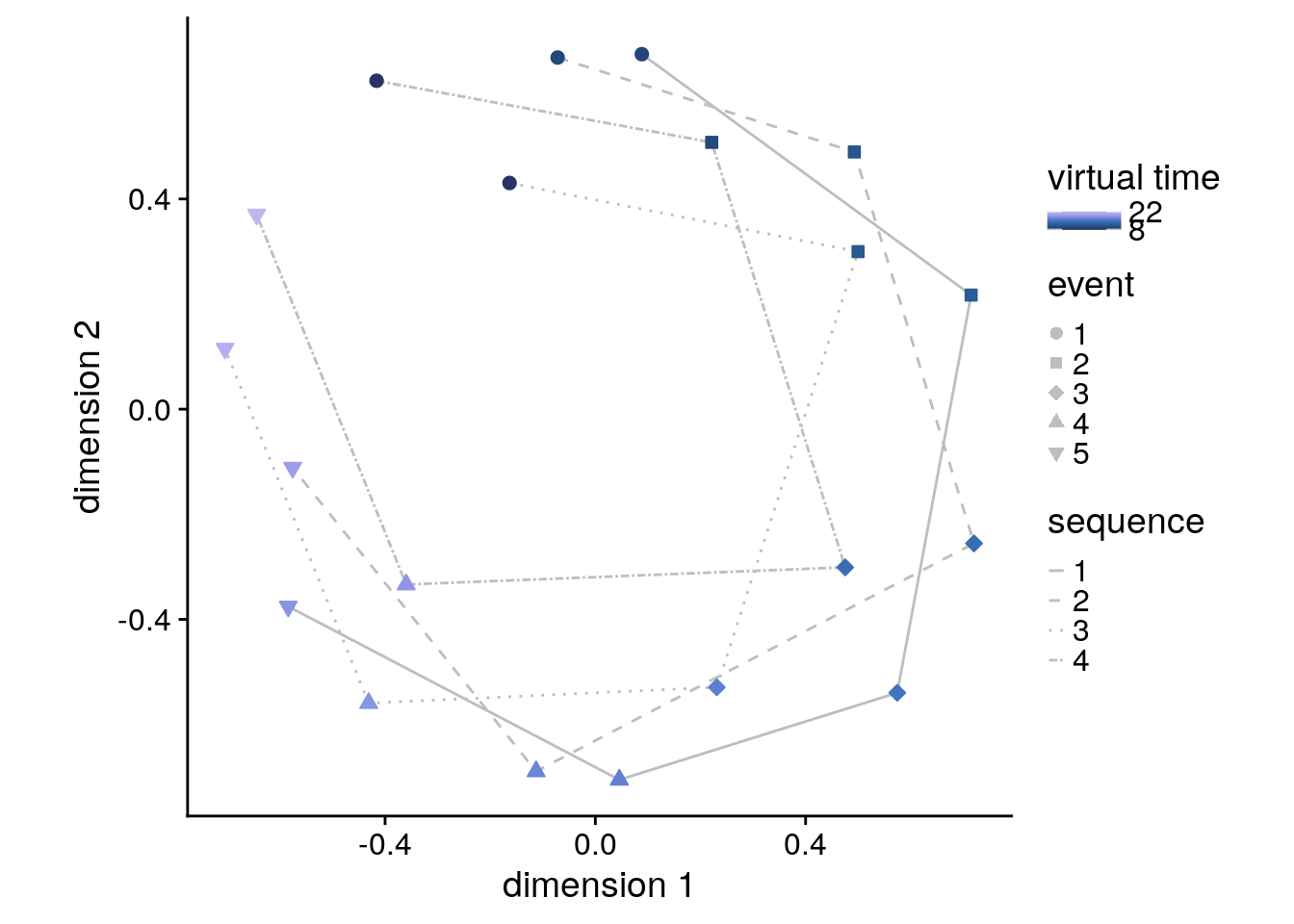#### Linear Mixed Effects As for the main analyses, we also want to run the same model using linear mixed effects. Due to singular fits we have to reduce the random effects structure until only random slopes are in the model. # extract the comparisons from the same day rsa_dat_diff_day <- rsa_dat_within_cluster %>% filter(same_day == FALSE) # define the full model with virtual time difference as # fixed effect and by-subject random slopes and random intercepts --> singular fit formula <- "ps_change ~ vir_time_diff + (1 + vir_time_diff | sub_id)" lmm_full <- lme4::lmer(formula, data = rsa_dat_diff_day, REML = FALSE) ## boundary (singular) fit: see ?isSingular # remove correlation --> still singular formula <- "ps_change ~ vir_time_diff + (1 + vir_time_diff || sub_id)" lmm_full <- lme4::lmer(formula, data = rsa_dat_diff_day, REML = FALSE) ## boundary (singular) fit: see ?isSingular # only random slopes set.seed(322) # set seed for reproducibility formula <- "ps_change ~ vir_time_diff + (0 + vir_time_diff | sub_id)" lmm_full <- lme4::lmer(formula, data = rsa_dat_diff_day, REML = FALSE) ## boundary (singular) fit: see ?isSingular summary(lmm_full, corr = FALSE) ## Linear mixed model fit by maximum likelihood ['lmerMod'] ## Formula: ps_change ~ vir_time_diff + (0 + vir_time_diff | sub_id) ## Data: rsa_dat_diff_day ## ## AIC BIC logLik deviance df.resid ## -23260.0 -23234.6 11634.0 -23268.0 4196 ## ## Scaled residuals: ## Min 1Q Median 3Q Max ## -4.0842 -0.6392 0.0084 0.6616 3.7002 ## ## Random effects: ## Groups Name Variance Std.Dev. ## sub_id vir_time_diff 0.0000000 0.00000 ## Residual 0.0002299 0.01516 ## Number of obs: 4200, groups: sub_id, 28 ## ## Fixed effects: ## Estimate Std. Error t value ## (Intercept) -9.718e-05 2.343e-04 -0.415 ## vir_time_diff -4.782e-04 2.341e-04 -2.043 ## convergence code: 0 ## boundary (singular) fit: see ?isSingular # tidy summary of the fixed effects that calculates 95% CIs lmm_full_bm <- broom.mixed::tidy(lmm_full, effects = "fixed", conf.int=TRUE, conf.method="profile", optimizer = 'nloptwrap') ## Computing profile confidence intervals ... # tidy summary of the random effects lmm_full_bm_re <- broom.mixed::tidy(lmm_full, effects = "ran_pars") # one way of testing for significance is by comparing the likelihood against a simpler model. # Here, we drop the effect of virtual time difference and run an ANOVA. See e.g. Bodo Winter tutorial set.seed(396) # set seed for reproducibility formula <- "ps_change ~ 1 + (0 + vir_time_diff | sub_id)" lmm_no_vir_time <- lme4::lmer(formula, data = rsa_dat_diff_day, REML = FALSE) lmm_aov <-anova(lmm_no_vir_time, lmm_full) Mixed Model: Fixed effect of virtual time across sequences in within-sequence searchlight peak $$\chi^2$$(1)=4.13, p=0.042 Make mixed model summary table that includes overview of fixed and random effects as well as the model comparison to the nested (reduced) model. fe_names <- c("intercept", "virtual time") re_groups <- c(rep("participant",1), "residual") re_names <- c("virtual time (SD)", "SD") lmm_hux <- make_lme_huxtable(fix_df=lmm_full_bm, ran_df = lmm_full_bm_re, aov_mdl = lmm_aov, fe_terms =fe_names, re_terms = re_names, re_groups = re_groups, lme_form = gsub(" ", "", paste0(deparse(formula(lmm_full)), collapse = "", sep="")), caption = "Mixed Model: Virtual time explains representational change for different-sequence events in the peak cluster of the same-sequence searchlight analysis") # convert the huxtable to a flextable for word export stable_lme_same_seq_cluster_virtime_diff_seq <- convert_huxtable_to_flextable(ht = lmm_hux) # print to screen theme_article(lmm_hux) fixed effects term estimate SE t-value -0.000097 0.000234 -0.41 -0.000557 0.000362 -0.000478 0.000234 -2.04 -0.000939 -0.000018 0.000000 0.015162 -23257.87 11631.93 -23260.00 11634.00 4.13 1 0.042 ### PLOTS lmm_full_bm <- lmm_full_bm %>% mutate(term=as.factor(term)) # dot plot of Fixed Effect Coefficients with CIs f7c <-ggplot(data = lmm_full_bm[2,], aes(x = term, color = term)) + geom_hline(yintercept = 0, colour="black", linetype="dotted") + geom_errorbar(aes(ymin = conf.low, ymax = conf.high, width = NA), size = 0.5) + geom_point(aes(y = estimate), shape = 16, size = 1) + scale_fill_manual(values = unname(aHPC_colors["across_main"])) + scale_color_manual(values = unname(aHPC_colors["across_main"]), labels = c("virtual time across sequences"), name = element_blank()) + labs(x = element_blank(), y = "fixed effect\nestimate") + guides(fill=FALSE, color=guide_legend(override.aes=list(fill=NA, alpha = 1, size=2, linetype=0))) + annotate(geom = "text", x = 1, y = 0.00003, label = "*", hjust = 0.5, size = 8/.pt, family=font2use) + theme_cowplot() + theme(legend.position = "none", axis.text.x=element_blank(), legend.justification = c(0.5,0)) f7c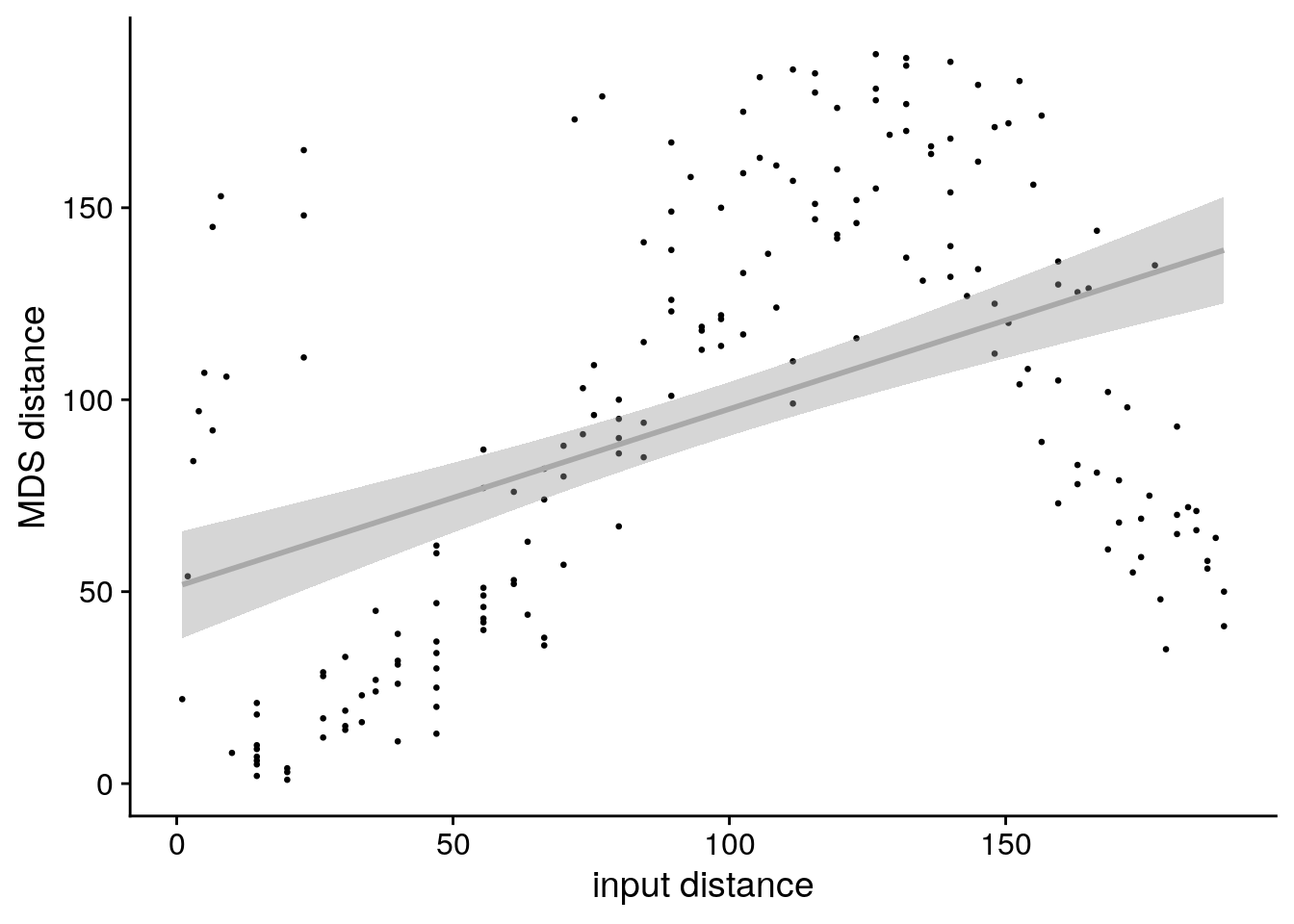# estimate marginal means for each model term by omitting the terms argument lmm_full_emm <- ggeffects::ggpredict(lmm_full, ci.lvl = 0.95) %>% ggeffects::get_complete_df() # plot marginal means f7d <- ggplot(data = lmm_full_emm[lmm_full_emm$group == "vir_time_diff",], aes(color = group)) +
geom_line(aes(x, predicted)) +
geom_ribbon(aes(x, ymin = conf.low, ymax = conf.high, fill = group), alpha = .2, linetype=0) +
scale_color_manual(values = unname(aHPC_colors["across_main"]),
name = element_blank(), labels = "virtual time") +
scale_fill_manual(values = unname(aHPC_colors["across_main"])) +
ylab('estimated\nmarginal means') +
xlab('z virtual time') +
#ggtitle("Estimated marginal means") +
guides(fill=FALSE, color=FALSE) +
theme_cowplot() +
theme(legend.position = "none")
f7d### Virtual Time Across Sequences

Next, we plot the results of the searchlight looking at the across-sequence comparisons. This effect fails to reach the significance threshold when correcting for multiple comparisons. For visualization, we show the effect at p<0.05 uncorrected (within FOV). We report strongest cluster after small volume correction.

# read the cluster file for corrected results
fn <- file.path(dirs$data4analysis, "searchlight_results", "diff_day_vir_time", "cluster_diff_day_vir_time_neg_svc_corrp_atlas.txt") corrp_df <- readr::read_tsv(fn, col_types = c("ccdddddddddd")) # print to screen cat("no significant clusters in across-day virtual time (negative) searchlight:", sprintf("\ncluster file has %d rows", nrow(corrp_df))) ## no significant clusters in across-day virtual time (negative) searchlight: ## cluster file has 0 rows # find and report peak voxel coordinates incl. p and t after SVC corrp_fn <- file.path(dirs$data4analysis, "searchlight_results","diff_day_vir_time",
"diff_day_vir_time_neg_randomise_svc_fwhm3_tfce_corrp_tstat1.nii.gz")
coords <- which(corrp_nii == max(c(corrp_nii)), arr.ind = TRUE)
mnicoords <- vox2mni(coords)

tvals_fn <- file.path(dirs$data4analysis, "searchlight_results","diff_day_vir_time", "diff_day_vir_time_neg_randomise_fov_fwhm3_tstat1.nii.gz") t_nii <- readNIfTI2(tvals_fn) cat("peak voxel in across-day virtual time (negative) searchlight:", sprintf("\nx=%d, y=%d, z=%d, t=%.2f, corrected p =%.3f", mnicoords, mnicoords, mnicoords, -t_nii[coords, coords, coords], 1-max(c(corrp_nii)))) ## peak voxel in across-day virtual time (negative) searchlight: ## x=-26, y=-19, z=-15, t=-3.96, corrected p =0.071 Report additional clusters from exploratory analysis with threshold p<0.001 uncorrected and a minimum of 30 voxels per cluster. # read the cluster file for uncorrected results fn <- file.path(dirs$data4analysis, "searchlight_results",
"diff_day_vir_time", "cluster_diff_day_vir_time_neg_fov_uncorrp_atlas.txt")

# quick print
#uncorrp_df %>% huxtable::as_huxtable() %>% theme_article()

# add column with p-values (not 1-p as given by FSL) and manual labels
uncorrp_df <- uncorrp_df %>%
mutate(
t_extr = t_extr*-1, # multiply t-values by minus 1 because we tested the negative contrast
p = 1-max_1minusp,
atlas_label = c(
"cerebellum",
"cerebellum",
"lingual gyrus"
)) %>%
select(-c(max_1minusp, "Harvard_Oxford_Cortical", "Harvard_Oxford_Subcortical", cluster_index)) %>%
relocate(atlas_label)

# add p-values because small values are reported as 0 by FSL cluster
uncorrp_fn <- file.path(dirs$data4analysis, "searchlight_results","diff_day_vir_time", "diff_day_vir_time_neg_randomise_fov_fwhm3_tfce_p_tstat1.nii.gz") uncorrp_nii <- readNIfTI2(uncorrp_fn) for (i in 1:nrow(uncorrp_df)){ coords <- mni2vox(c(uncorrp_df[i,]$x,uncorrp_df[i,]$y,uncorrp_df[i,]$z))
uncorrp_df[i,]$p <- 1-uncorrp_nii[coords, coords, coords] } Create pretty table that can be written to Word. uncorrp_ht <- uncorrp_df %>% as_huxtable() %>% huxtable::set_contents(., row=1, value =c("Atlas Label", "Voxel Extent", "x", "y", "z", "COG x", "COG y", "COG z", "t", "p")) %>% huxtable::insert_row(., rep("", ncol(.)), after=0) %>% huxtable::merge_across(.,1,1:ncol(.)) %>% huxtable::set_contents(., row=1, value ="Exploratory searchlight results, p-values uncorrected") %>% huxtable::set_header_rows(.,row=1, value=TRUE) %>% theme_article() uncorrp_ht Table 8.27: Exploratory searchlight results, p-values uncorrected Atlas LabelVoxel ExtentxyzCOG xCOG yCOG ztp cerebellum31419-68-3419.1 -66.3-29.6 -5.370.0002 cerebellum104-1-68-14-1.86-69.1-14.3 -3.440.0002 lingual gyrus100-1-704-2.68-70.54.56-3.730.0002 stable_srchlght_diff_seq <- uncorrp_ht %>% huxtable::add_footnote("x, y, z refer to MNI coordinates of minimum p-value in cluster, t denotes the most extreme t-value, COG: center of gravity", border = 0.5) %>% huxtable::as_flextable() %>% flextable::fontsize(size=10, part="all") %>% flextable::font(fontname = font2use) %>% flextable::style(i=c(1), pr_c = officer::fp_cell(border.bottom = fp_border(), border.top = fp_border())) %>% flextable::bg(i=1, bg="lightgrey", part="all") %>% flextable::padding(., padding = 3, part="all") %>% set_table_properties(., width=1, layout = "autofit") %>% flextable::set_caption(., caption = "Searchlight Analysis: Virtual time explains representational change for different-sequence events", style="Normal") # choose coordinates (these are chosen manually now) and get a data frame for labeling the plots coords <- mni2vox(c(-25,-19,-15)) label_df <- data.frame(col_ind = sprintf("%s=%d",c("x","y","z"), vox2mni(coords)), label = sprintf("%s=%d",c("x","y","z"), vox2mni(coords))) # get ggplot object for template as background ggTemplate<-getggTemplate(col_template=rev(RColorBrewer::brewer.pal(8, 'Greys')),brains=mni_nii, all_brain_and_time_inds_one=TRUE, mar=c(1,2,3), mar_ind=coords, row_ind = c(1,1,1), col_ind=sprintf("%s=%d",c("x","y","z"),vox2mni(coords)), center_coords=TRUE) # get uncorrected p-values in FOV pfov_fn <- file.path(dirs$data4analysis, "searchlight_results","diff_day_vir_time",
"diff_day_vir_time_neg_randomise_fov_fwhm3_tfce_p_tstat1.nii.gz")

# t-values to plot (FOV)
tvals_fn <- file.path(dirs$data4analysis, "searchlight_results","diff_day_vir_time", "diff_day_vir_time_neg_randomise_fov_fwhm3_tstat1.nii.gz") t_nii <- readNIfTI2(tvals_fn) # CAVE: because we are looking at the negative contrast, multiply the t-values by -1 to actually show # negative t-values (they have previously been multiplied by -1 to run randomise, now revert this) t_nii <- t_nii * -1 # threshold t-values for plotting thresh <- 0.05 t_nii[pfov_nii < 1-thresh] <- NA # get data frame for the t-values t_df <- getBrainFrame(brains=t_nii, mar=c(1,2,3), col_ind = sprintf("%s=%d",c("x","y","z"),vox2mni(coords)), mar_ind=coords, mask=NULL, center_coords=TRUE) f7e <- ggTemplate + geom_tile(data=t_df, aes(x=row,y=col,fill=value))+ facet_wrap(~col_ind, nrow=3, ncol=1, strip.position = "top", scales = "free") + scico::scale_fill_scico(palette = "lajolla", begin = 0.3, end=0.9, direction = -1, name = element_text("t"), limits = round(range(t_df$value), digits=2),
breaks = round(range(t_dfvalue), digits=2)) + ggtitle(label = "\ndifferent\nsequence") + theme_cowplot(line_size = NA) + theme(strip.background = element_blank(), strip.text.x = element_blank(), plot.title = element_text(hjust = 0.5), text = element_text(size=10), axis.text = element_text(size=8), legend.text=element_text(size=8), legend.title = element_text(size=8), legend.key.width = unit(0.01, "npc"), legend.key.height = unit(0.015, "npc"), legend.position = "bottom", legend.justification = c(0.5, 0), aspect.ratio = 1)+ guides(fill = guide_colorbar( direction = "horizontal", title.position = "top", title.hjust = 0.5, label.position = "bottom"))+ xlab(element_blank()) + ylab(element_blank()) + coord_cartesian(clip = "off") + geom_text(data = label_df, x=0, y=c(-84.7,-70.37,-90.69), aes(label = label), size = 8/.pt, family=font2use, vjust=1) + # y coords found via command below (to keep text aligned across panels): # ggplot_build(f6g)layout$panel_scales_y[]$range$range-diff(ggplot_build(f6g)$layout$panel_scales_y[]$range$range)/100*3 theme_no_ticks() print(f7e)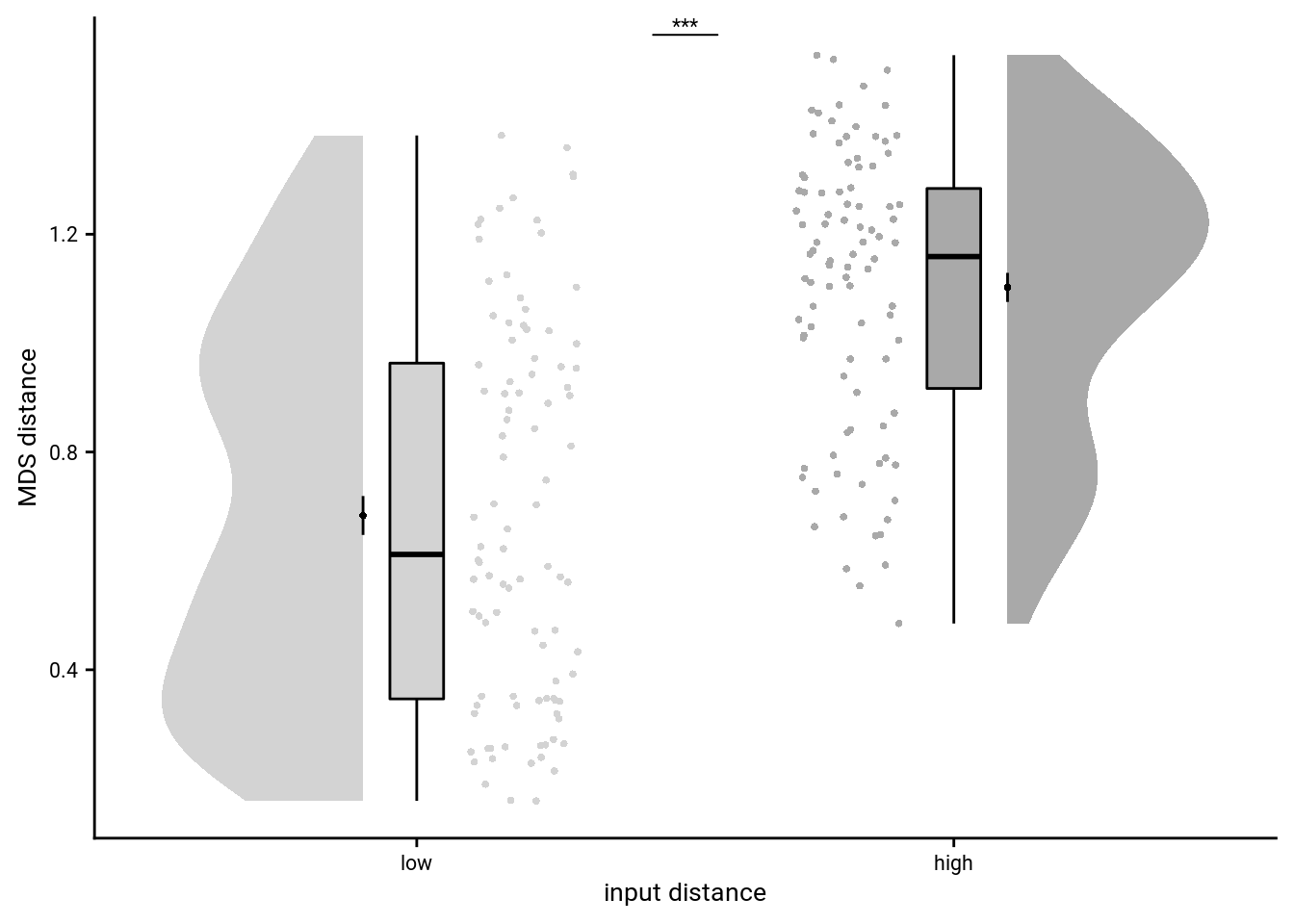### Visualization of Overlap To visualize the overlap between the within- and the between-sequence effects we next create an overlap plot on a zoomed-in plot of the hippocampal region. This is for visual purposes only, so we use a liberal threshold of p<0.05 within our small-volume-correction mask. # choose coordinates (these are chosen manually now) and get a data frame for labeling the plots coords <- mni2vox(c(-25,-18,-16)) label_df <- data.frame(col_ind = sprintf("%s=%d",c("x","y", "z"),vox2mni(coords)), label = sprintf("%s=%d",c("x","y", "z"),vox2mni(coords))) # for this plot we want to "zoom in", which we accomplish by masking out all but a small region of the brain box2remove<-array(1, dim = dim(mni_nii)) box2remove[c(1:51, 131:182),,] <- 0 box2remove[,c(1:68, 150:218),] <- 0 box2remove[,,c(1:21, 101:182)] <- 0 mni_zoom_nii <- mni_nii * box2remove mni_zoom_nii[mni_zoom_nii==0] <- NA # get ggplot object for template as background ggTemplate<-getggTemplate(col_template=rev(RColorBrewer::brewer.pal(8, 'Greys')),brains=mni_zoom_nii, all_brain_and_time_inds_one=TRUE, mar=c(1,2,3), mar_ind=coords, row_ind = c(1,1,1), col_ind=sprintf("%s=%d",c("x","y","z"),vox2mni(coords)), center_coords=TRUE) thresh <- 0.05 # get uncorrected p-values in FOV fn <- file.path(dirs$data4analysis, "searchlight_results","same_day_vir_time",
"same_day_vir_time_randomise_svc_fwhm3_tfce_p_tstat1.nii.gz")
sameday_nii[sameday_nii > 1-thresh] = 1
sameday_nii[sameday_nii < 1-thresh] = 0

fn <- file.path(dirsdata4analysis, "searchlight_results","diff_day_vir_time", "diff_day_vir_time_neg_randomise_svc_fwhm3_tfce_p_tstat1.nii.gz") diffday_nii <- readNIfTI2(fn) diffday_nii[diffday_nii > 1-thresh] = 2 diffday_nii[diffday_nii < 1-thresh] = 0 overlap_nii <- sameday_nii + diffday_nii overlap_nii[overlap_nii < 1] = NA # get data frame for the t-values o_df <- getBrainFrame(brains=overlap_nii, mar=c(1,2,3), col_ind = sprintf("%s=%d",c("x","y","z"),vox2mni(coords)), mar_ind=coords, mask=NULL, center_coords=TRUE) %>% mutate(value = as.factor(value), value = recode_factor(value, 1="same", 2="different", 3="overlap")) f7f <- ggTemplate + geom_tile(data=o_df, aes(x=row,y=col,fill=value))+ facet_wrap(~col_ind, nrow=3, ncol=1, strip.position = "bottom", scales = "free") + scale_fill_manual(values = unname(c(aHPC_colors["within_main"], aHPC_colors["across_main"], day_time_int_color)), guide = guide_legend(override.aes=list( alpha = 1, shape=16, size=2, linetype=0), direction = "vertical", title=element_blank(), label.position = "right")) + ggtitle(label = "\noverlap\n") + theme_cowplot(line_size = NA) + theme(strip.background = element_blank(), strip.text.x = element_blank(), plot.title = element_text(hjust = 0.5), text = element_text(size=10), axis.text = element_text(size=8), legend.text=element_text(size=8), legend.title = element_text(size=8), legend.key.size = unit(0.02, "npc"), legend.position = "bottom", legend.justification = c(0.5, 0), aspect.ratio = 1)+ xlab(element_blank()) + ylab(element_blank()) + coord_cartesian(clip = "off") + geom_text(data = label_df, x=0, y=c(-71.87,-67.75,-42.93), aes(label = label), size = 8/.pt, family=font2use, vjust=1) + # y coords found via command below (to keep text aligned across panels): # ggplot_build(f6g)layout$panel_scales_y[]$range$range-diff(ggplot_build(f6g)$layout$panel_scales_y[]$range$range)/100*3 theme_no_ticks() print(f7f)### Virtual Time & Sequence Interaction In our searchlight analyses, we also directly looked for voxels where the effect of virtual time differs depending on whether we are looking at events from the same sequence or from different sequences, i.e. an interaction of virtual temporal distances and a sequence factor (same/different). Report significant clusters after small volume correction. # read the cluster file for corrected results fn <- file.path(dirs$data4analysis, "searchlight_results",
"day_time_interaction", "cluster_day_time_interaction_svc_corrp_atlas.txt")

# quick print
#corrp_df %>% huxtable::as_huxtable() %>% theme_article()

# add column with p-values (not 1-p as given by FSL) and manual labels
corrp_df <- corrp_df %>%
mutate(
p = 1-max_1minusp,
atlas_label = c("left hippocampus", "right hippocampus")) %>%
select(-c(max_1minusp, "Harvard_Oxford_Cortical", "Harvard_Oxford_Subcortical", cluster_index)) %>%
relocate(atlas_label)

# print to screen
cat("significant clusters in day-time interaction searchlight:",
sprintf("\n%s, peak voxel at x=%d, y=%d, z=%d; t=%.2f, corrected p=%.3f",
corrp_df$atlas_label, corrp_df$x, corrp_df$y, corrp_df$z, corrp_df$t_extr, corrp_df$p))
## significant clusters in day-time interaction searchlight:
## left hippocampus, peak voxel at x=-26, y=-20, z=-15; t=4.15, corrected p=0.014
## right hippocampus, peak voxel at x=31, y=-16, z=-21; t=4.25, corrected p=0.007

Report additional clusters from exploratory analysis with threshold p<0.001 uncorrected and a minimum of 30 voxels per cluster.

# read the cluster file for uncorrected results
fn <- file.path(dirs$data4analysis, "searchlight_results", "day_time_interaction", "cluster_day_time_interaction_fov_uncorrp_atlas.txt") uncorrp_df <- readr::read_tsv(fn, col_types = c("ccdddddddddd")) # quick print #uncorrp_df %>% huxtable::as_huxtable() %>% theme_article() # add column with p-values (not 1-p as given by FSL) and manual labels uncorrp_df <- uncorrp_df %>% mutate( p = 1-max_1minusp, atlas_label = c( "left hippocampus", "right hippocampus", "occipital pole", "lingual gyrus", "frontal pole", "frontal pole", "temporal fusiform cortex", "intracalcarine sulcus")) %>% select(-c(max_1minusp, "Harvard_Oxford_Cortical", "Harvard_Oxford_Subcortical", cluster_index)) %>% filter(!str_detect(atlas_label, "hippocampus")) %>% # remove hippocampal clusters (already reported with SVC) relocate(atlas_label) # add p-values because small values are reported as 0 by FSL cluster uncorrp_fn <- file.path(dirs$data4analysis, "searchlight_results","day_time_interaction",
"day_time_interaction_randomise_fov_fwhm3_tfce_p_tstat1.nii.gz")
for (i in 1:nrow(uncorrp_df)){
coords <- mni2vox(c(uncorrp_df[i,]$x,uncorrp_df[i,]$y,uncorrp_df[i,]$z)) uncorrp_df[i,]$p <- 1-uncorrp_nii[coords, coords, coords]
}

Make pretty overview table that can be saved to Word.

corrp_ht <- corrp_df %>%
as_huxtable() %>%
huxtable::set_contents(., row=1, value =c("Atlas Label", "Voxel Extent", "x", "y", "z", "COG x", "COG y", "COG z", "t", "p")) %>%
huxtable::insert_row(., rep("", ncol(.)), after=0) %>%
huxtable::merge_across(.,1,1:ncol(.)) %>%
huxtable::set_contents(., row=1, value ="Searchlight results in a priori regions of interest, p-values corrected using small volume correction") %>%
theme_article()
corrp_ht
Table 8.28:
Searchlight results in a priori regions of interest, p-values corrected using small volume correction
Atlas LabelVoxel ExtentxyzCOG xCOG yCOG ztp
left hippocampus359-26-20-15-23.4-15.5-18.64.150.014
right hippocampus33531-16-2130.7-15.1-20.14.250.007
uncorrp_ht <- uncorrp_df %>%
as_huxtable() %>%
huxtable::set_contents(., row=1, value =c("Atlas Label", "Voxel Extent", "x", "y", "z", "COG x", "COG y", "COG z", "t", "p")) %>%
huxtable::insert_row(., rep("", ncol(.)), after=0) %>%
huxtable::merge_across(.,1,1:ncol(.)) %>%
huxtable::set_contents(., row=1, value ="Exploratory searchlight results, p-values uncorrected") %>%
theme_article()
uncorrp_ht
Table 8.28:
Exploratory searchlight results, p-values uncorrected
Atlas LabelVoxel ExtentxyzCOG xCOG yCOG ztp
occipital pole10317-91-817.7 -90.6-6.624.080.0002
lingual gyrus102-5-735-3.59-70.45.013.720.0002
frontal pole9643431845.4 43.419.7 4.310.0006
frontal pole4535431737   43.218.5 3.810.0006
temporal fusiform cortex40-25-10-45-25.3 -10.3-42.9 3.140.0004
intracalcarine sulcus33-4-7711-2.85-75.811.5 3.560.0002
stable_srchlght_interaction <- rbind(corrp_ht, uncorrp_ht) %>%
huxtable::add_footnote("x, y, z refer to MNI coordinates of minimum p-value in cluster, t denotes the most extreme t-value, COG: center of gravity", border = 0.5) %>%
huxtable::as_flextable() %>%
flextable::fontsize(size=10, part="all") %>%
flextable::font(fontname = font2use) %>%
flextable::style(i=c(1,nrow(corrp_ht)+1),
pr_c = officer::fp_cell(border.bottom = fp_border(),
border.top = fp_border())) %>%
flextable::bg(i=c(1,nrow(corrp_ht)+1), bg="lightgrey", part="all") %>%
flextable::set_table_properties(., width=1, layout = "autofit") %>%
flextable::set_caption(., caption = "Searchlight Analysis: Interaction of virtual time and sequence membership",
style="Normal")

As for the within-day effect, we show the t-values at p<0.01 uncorrected (based on field of view) and outline voxels surviving corrections (small volume correction) in black.

# choose coordinates (these are chosen manually now) and get a data frame for labeling the plots
coords <- mni2vox(c(31,-15,-19))
label_df <- data.frame(col_ind = sprintf("%s=%d",c("x","y", "z"),vox2mni(coords)),
label = sprintf("%s=%d",c("x","y", "z"),vox2mni(coords)))

# get ggplot object for template as background
ggTemplate<-getggTemplate(col_template=rev(RColorBrewer::brewer.pal(8, 'Greys')),brains=mni_nii,
all_brain_and_time_inds_one=TRUE,
mar=c(1,2,3), mar_ind=coords, row_ind = c(1,1,1),
col_ind=sprintf("%s=%d",c("x","y","z"),vox2mni(coords)), center_coords=TRUE)

# get uncorrected p-values in FOV
pfov_fn <- file.path(dirs$data4analysis, "searchlight_results","day_time_interaction", "day_time_interaction_randomise_fov_fwhm3_tfce_p_tstat1.nii.gz") pfov_nii <- readNIfTI2(pfov_fn) # t-values to plot (FOV) tvals_fn <- file.path(dirs$data4analysis, "searchlight_results","day_time_interaction",
"day_time_interaction_randomise_fov_fwhm3_tstat1.nii.gz")

# threshold t-values for plotting
thresh <- 0.01
t_nii[pfov_nii < 1-thresh] <- NA

# get data frame for the t-values
t_df <- getBrainFrame(brains=t_nii, mar=c(1,2,3),
col_ind = sprintf("%s=%d",c("x","y","z"),vox2mni(coords)),

outl_fn <- file.path(dirs$data4analysis, "searchlight_results","day_time_interaction", "day_time_interaction_randomise_svc_fwhm3_tfce_corrp_outline.nii.gz") outl_nii <- readNIfTI2(outl_fn) # remove zero voxels and get dataframe at coordinates outl_nii[outl_nii == 0] <- NA o_df <- getBrainFrame(brains=outl_nii, mar=c(1,2,3), col_ind = sprintf("%s=%d",c("x","y","z"),vox2mni(coords)), mar_ind=coords, mask=NULL, center_coords=TRUE) f7g <- ggTemplate + geom_tile(data=t_df, aes(x=row,y=col,fill=value))+ geom_tile(data=o_df, aes(x=row,y=col), fill="black")+ facet_wrap(~col_ind, nrow=3, ncol=1, strip.position = "bottom", scales = "free") + scico::scale_fill_scico(palette = "buda", begin = 0, end=1, name = element_text("t"), limits = round(range(t_df$value), digits=2),
breaks = round(range(t_dfvalue), digits=2)) + ggtitle(label = "\nsame vs.\ndifferent") + theme_cowplot(line_size = NA) + theme(strip.background = element_blank(), strip.text.x = element_blank(), plot.title = element_text(hjust = 0.5), text = element_text(size=10), axis.text = element_text(size=8), legend.text=element_text(size=8), legend.title = element_text(size=8), legend.key.width = unit(0.01, "npc"), legend.key.height = unit(0.015, "npc"), legend.position = "bottom", legend.justification = c(0.5, 0), aspect.ratio = 1)+ guides(fill = guide_colorbar( direction = "horizontal", title.position = "top", title.hjust = 0.5, label.position = "bottom"))+ xlab(element_blank()) + ylab(element_blank()) + coord_cartesian(clip = "off") + geom_text(data = label_df, x=0, y=c(-84.64,-67.25,-86.51), aes(label = label), size = 8/.pt, family=font2use, vjust=1) + # y coords found via command below (to keep text aligned across panels): # ggplot_build(f6g)layout$panel_scales_y[]$range$range-diff(ggplot_build(f6g)$layout$panel_scales_y[]$range\$range)/100*3
theme_no_ticks()
print(f7g)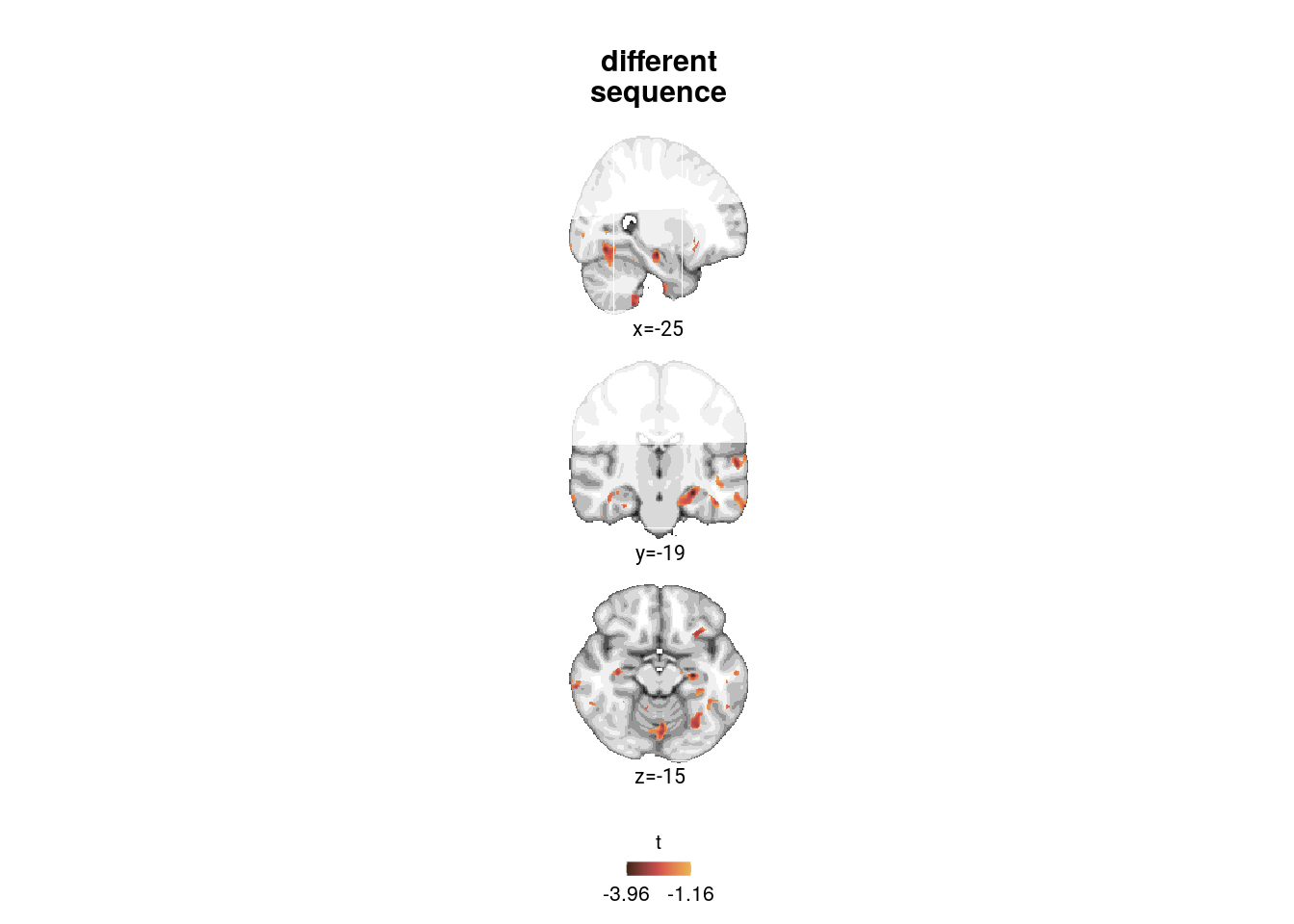Let’s compose figure 7.

layoutBD <- "
AB
AC
"

f7bd <- f7b+f7c+f7d +
plot_layout(design = layoutBD, guides = "collect") &
theme(text = element_text(size=10, family=font2use),
axis.text = element_text(size=8),
legend.text=element_text(size=8),
legend.title=element_text(size=8),
legend.spacing.x = unit(1, 'mm'),
legend.key.size = unit(3,"mm"),
legend.position = 'none')

layout <- "
ABBEFG
ABBEFG"

f7 <- f7a+f7bd+f7e+f7f+f7g +
plot_layout(design = layout, guides = "keep") &
plot_annotation(theme = theme(plot.margin = margin(t=0, r=-8, b=0, l=-19, unit="pt")),
tag_levels = 'A') &
theme(plot.tag = element_text(size = 10, face="bold"),
#plot.tag.position = "topleft",
#plot.margin=grid::unit(c(5,8,5,0), "pt"),
text = element_text(size=10, family=font2use),
plot.title = element_text(family=font2use, size=10, face="plain"))

f7[] <- f7[] +
theme(legend.position = c(0.5,-0.18),